nlmixr

Building on the first simple example, we can be more ambitious, and try a full PBPK model. This one was published for mavoglurant (Wendling et al. 2016).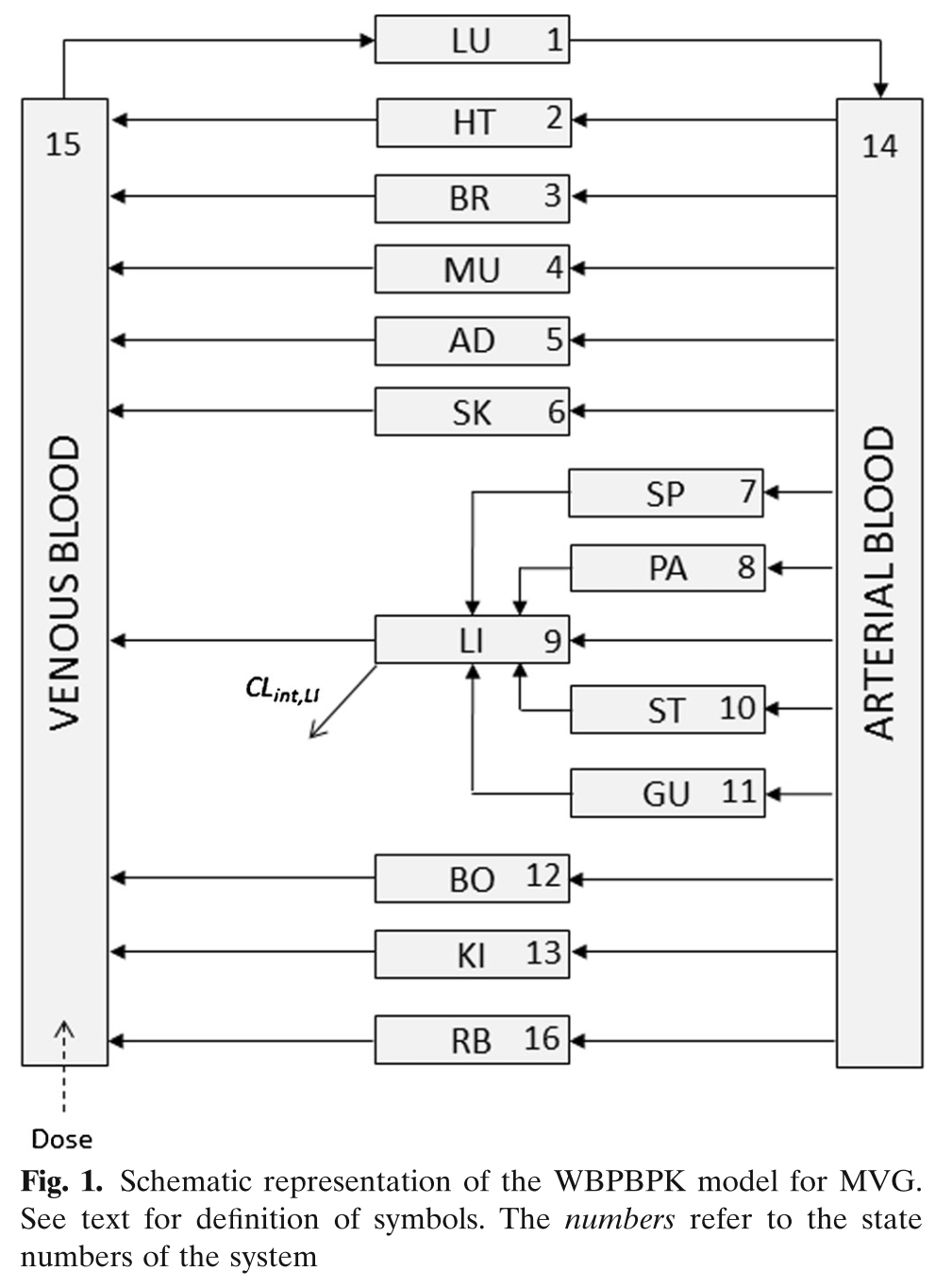Model Schematic

# nlmixr model

library(nlmixr)
## These options cache the models and the model simulations in R
## To run the actual models on your system, take the save options off.
options(nlmixr.save=TRUE,
nlmixr.save.dir=system.file(package="nlmixr.examples"));

## Note to get the datasets you can use:
## remotes::install_github("nlmixrdevelopment/nlmixr.examples")
library(nlmixr.examples) ## For nlmixr.examples data

library(xpose)
#> Loading required package: ggplot2
#>
#> Attaching package: 'xpose'
#> The following object is masked from 'package:nlmixr':
#>
#>     vpc
#> The following object is masked from 'package:stats':
#>
#>     filter
library(xpose.nlmixr)
#>
#> Attaching package: 'xpose.nlmixr'
#> The following object is masked from 'package:nlmixr':
#>
#>     vpc
library(ggplot2)

pbpk <- function(){
ini({
##theta=exp(c(1.1, .3, 2, 7.6, .003, .3))
lKbBR = 1.1
lKbMU = 0.3
lKbAD = 2
lCLint = 7.6
lKbBO = 0.03
lKbRB = 0.3
eta.LClint ~ 4
add.err <- 1
prop.err <- 10
})
model({
KbBR = exp(lKbBR)
KbMU = exp(lKbMU)
KbAD = exp(lKbAD)
CLint= exp(lCLint + eta.LClint)
KbBO = exp(lKbBO)
KbRB = exp(lKbRB)

## Regional blood flows
CO  = (187.00*WT^0.81)*60/1000;         # Cardiac output (L/h) from White et al (1968)
QHT = 4.0 *CO/100;
QBR = 12.0*CO/100;
QMU = 17.0*CO/100;
QAD = 5.0 *CO/100;
QSK = 5.0 *CO/100;
QSP = 3.0 *CO/100;
QPA = 1.0 *CO/100;
QLI = 25.5*CO/100;
QST = 1.0 *CO/100;
QGU = 14.0*CO/100;
QHA = QLI - (QSP + QPA + QST + QGU); # Hepatic artery blood flow
QBO = 5.0 *CO/100;
QKI = 19.0*CO/100;
QRB = CO - (QHT + QBR + QMU + QAD + QSK + QLI + QBO + QKI);
QLU = QHT + QBR + QMU + QAD + QSK + QLI + QBO + QKI + QRB;

## Organs' volumes = organs' weights / organs' density
VLU = (0.76 *WT/100)/1.051;
VHT = (0.47 *WT/100)/1.030;
VBR = (2.00 *WT/100)/1.036;
VMU = (40.00*WT/100)/1.041;
VAD = (21.42*WT/100)/0.916;
VSK = (3.71 *WT/100)/1.116;
VSP = (0.26 *WT/100)/1.054;
VPA = (0.14 *WT/100)/1.045;
VLI = (2.57 *WT/100)/1.040;
VST = (0.21 *WT/100)/1.050;
VGU = (1.44 *WT/100)/1.043;
VBO = (14.29*WT/100)/1.990;
VKI = (0.44 *WT/100)/1.050;
VAB = (2.81 *WT/100)/1.040;
VVB = (5.62 *WT/100)/1.040;
VRB = (3.86 *WT/100)/1.040;

## Fixed parameters
BP = 0.61;      # Blood:plasma partition coefficient
fup = 0.028;    # Fraction unbound in plasma
fub = fup/BP;   # Fraction unbound in blood

KbLU = exp(0.8334);
KbHT = exp(1.1205);
KbSK = exp(-.5238);
KbSP = exp(0.3224);
KbPA = exp(0.3224);
KbLI = exp(1.7604);
KbST = exp(0.3224);
KbGU = exp(1.2026);
KbKI = exp(1.3171);

##-----------------------------------------
S15 = VVB*BP/1000;
C15 = Venous_Blood/S15

##-----------------------------------------
d/dt(Lungs) = QLU*(Venous_Blood/VVB - Lungs/KbLU/VLU);
d/dt(Heart) = QHT*(Arterial_Blood/VAB - Heart/KbHT/VHT);
d/dt(Brain) = QBR*(Arterial_Blood/VAB - Brain/KbBR/VBR);
d/dt(Muscles) = QMU*(Arterial_Blood/VAB - Muscles/KbMU/VMU);
d/dt(Adipose) = QAD*(Arterial_Blood/VAB - Adipose/KbAD/VAD);
d/dt(Skin) = QSK*(Arterial_Blood/VAB - Skin/KbSK/VSK);
d/dt(Spleen) = QSP*(Arterial_Blood/VAB - Spleen/KbSP/VSP);
d/dt(Pancreas) = QPA*(Arterial_Blood/VAB - Pancreas/KbPA/VPA);
d/dt(Liver) = QHA*Arterial_Blood/VAB + QSP*Spleen/KbSP/VSP + QPA*Pancreas/KbPA/VPA + QST*Stomach/KbST/VST + QGU*Gut/KbGU/VGU - CLint*fub*Liver/KbLI/VLI - QLI*Liver/KbLI/VLI;
d/dt(Stomach) = QST*(Arterial_Blood/VAB - Stomach/KbST/VST);
d/dt(Gut) = QGU*(Arterial_Blood/VAB - Gut/KbGU/VGU);
d/dt(Bones) = QBO*(Arterial_Blood/VAB - Bones/KbBO/VBO);
d/dt(Kidneys) = QKI*(Arterial_Blood/VAB - Kidneys/KbKI/VKI);
d/dt(Arterial_Blood) = QLU*(Lungs/KbLU/VLU - Arterial_Blood/VAB);
d/dt(Venous_Blood) = QHT*Heart/KbHT/VHT + QBR*Brain/KbBR/VBR + QMU*Muscles/KbMU/VMU + QAD*Adipose/KbAD/VAD + QSK*Skin/KbSK/VSK + QLI*Liver/KbLI/VLI + QBO*Bones/KbBO/VBO + QKI*Kidneys/KbKI/VKI + QRB*Rest_of_Body/KbRB/VRB - QLU*Venous_Blood/VVB;
d/dt(Rest_of_Body) = QRB*(Arterial_Blood/VAB - Rest_of_Body/KbRB/VRB);

C15 ~ add(add.err) + prop(prop.err)
})
}

dat = read.csv("Mavoglurant_A2121_nmpk.csv")
dat$occ = unlist(with(dat, tapply(EVID, ID, function(x) cumsum(x>0)))) dat = subset(dat, occ==1) dat = subset(dat, ID<812) ## First 20 dat = subset(dat, EVID>0 | DV>0) dat$CMT[dat$CMT == 0] <- 1; dat$CMT[dat$EVID == 1] <- "Venous_Blood" ## Compartment dosed to is Venous Blood dat$CMT[dat$EVID != 1] <- "C15" ## Observing C15 gofs <- function(fit){ ################################################################################ ## Standard plots ################################################################################ plot(fit); fit <- addCwres(fit); xpdb <- xpose_data_nlmixr(fit) ## Convert to nlmixr object print(dv_vs_pred(xpdb) + ylab("Observed Mavoglurant Concentrations (ng/mL)") + xlab("Population Predicted Mavoglurant Concentrations (ng/mL)")); print(dv_vs_ipred(xpdb) + ylab("Observed Mavoglurant Concentrations (ng/mL)") + xlab("Individual Predicted Mavoglurant Concentrations (ng/mL)")); print(res_vs_pred(xpdb) + ylab("Conditional Weighted Residuals") + xlab("Population Predicted Mavoglurant Concentrations (ng/mL)")); print(res_vs_idv(xpdb) + ylab("Conditional Weighted Residuals") + xlab("Time (h)")); if (!is.null(fit$saem)){
print(prm_vs_iteration(xpdb));
}

print(absval_res_vs_idv(xpdb, res = 'IWRES') +
ylab("Individual Weighted Residuals") +
xlab("Time (h)"))

print(absval_res_vs_pred(xpdb, res = 'IWRES') +
ylab("Individual Weighted Residuals") +
xlab("Population Predicted Mavoglurant Concentrations (ng/mL)"))

print(ind_plots(xpdb, nrow=3, ncol=4) +
ylab("Predicted and Observed Mavoglurant Concentrations (ng/mL)") +
xlab("Time (h)"))

print(res_distrib(xpdb) +
ylab("Density") +
xlab("Conditional Weighted Residuals"));
##Visual Predictive Checks
f1 <- vpc.ui(fit,n=500,stratify=c("dose"), show=list(obs_dv=T), log_y=TRUE,
bins = c(0, 2, 4, 6, 8, 10, 20, 30, 40, 50),
ylab = "Mavoglurant Concentrations (ng/mL)",
xlab = "Time (hours)")
f2 <- vpc.ui(fit,n=500, show=list(obs_dv=T), bins = c(0, 2, 4, 6, 8, 10, 20, 30, 40, 50), log_y=TRUE,
ylab = "Mavoglurant Concentrations (ng/mL)", xlab = "Time (hours)")
plot(f1)
plot(f2)
}

# SAEM

## Fit add+prop SAEM

fit.addProp.S <- nlmixr(pbpk, dat, est="saem")
#> Loading model already run (/home/matt/R/x86_64-pc-linux-gnu-library/3.5/nlmixr.examples/nlmixr-pbpk-dat-saem-6e7e039679a37634c1367f96b7c46c93.rds)

gofs(fit.addProp.S);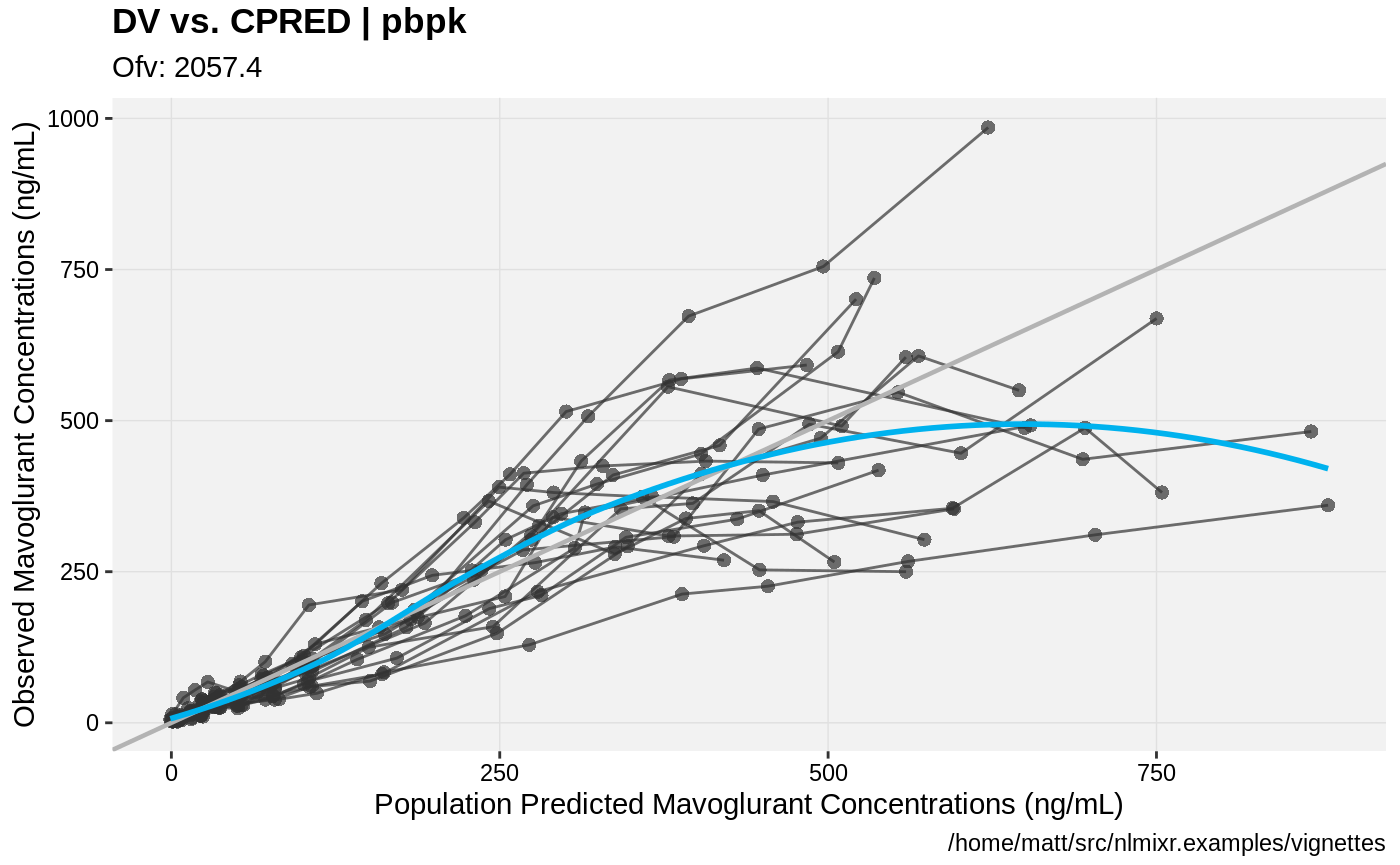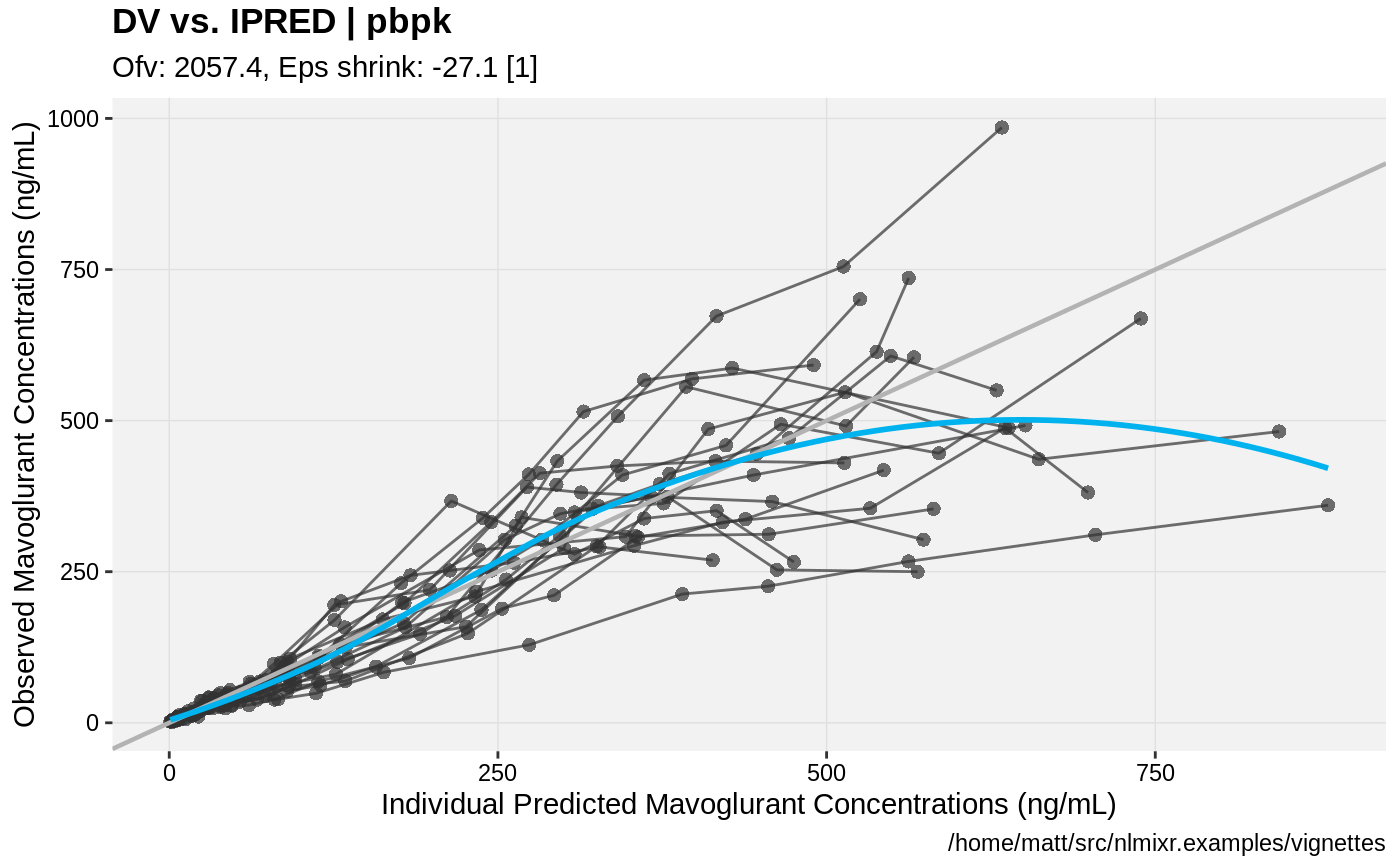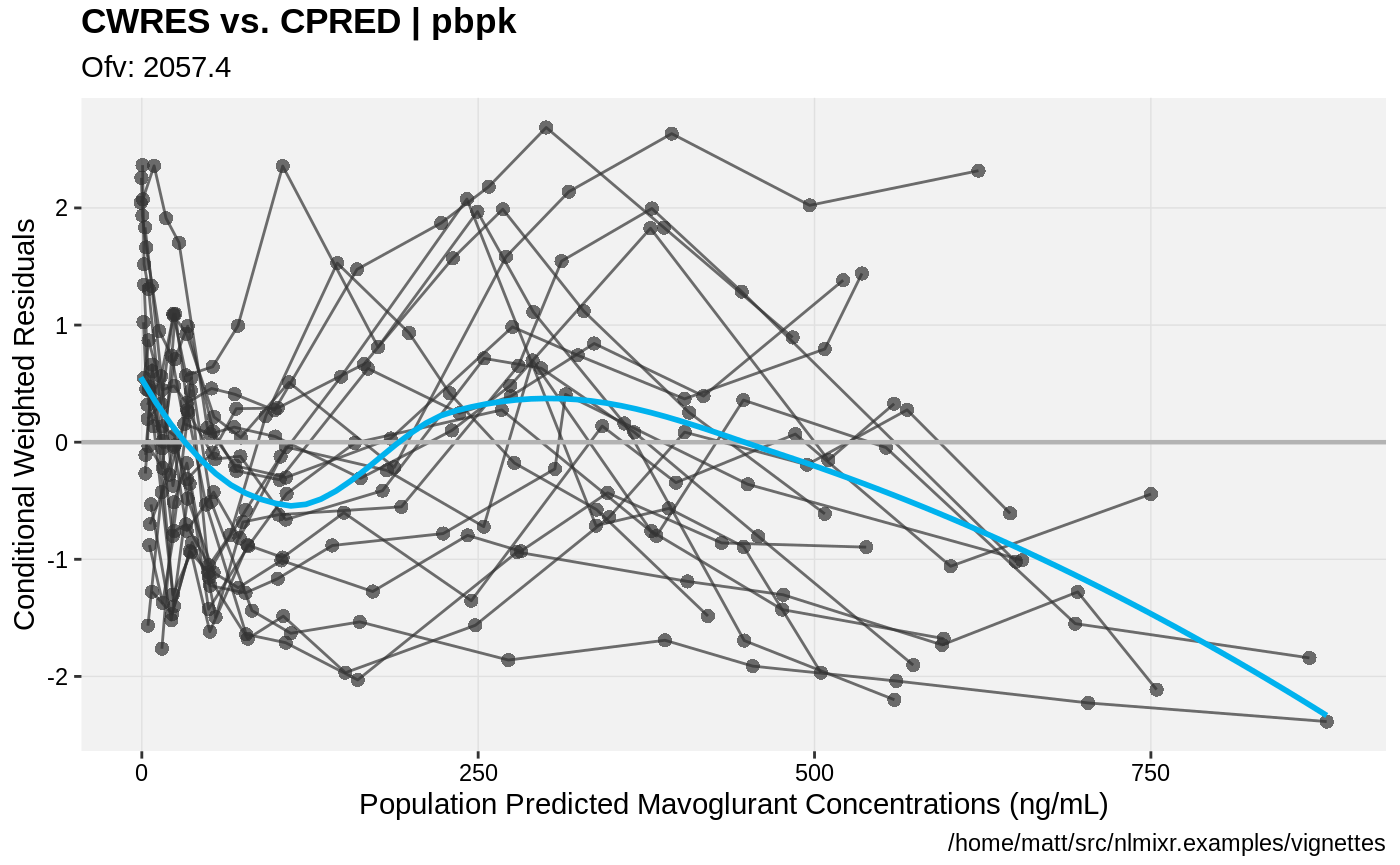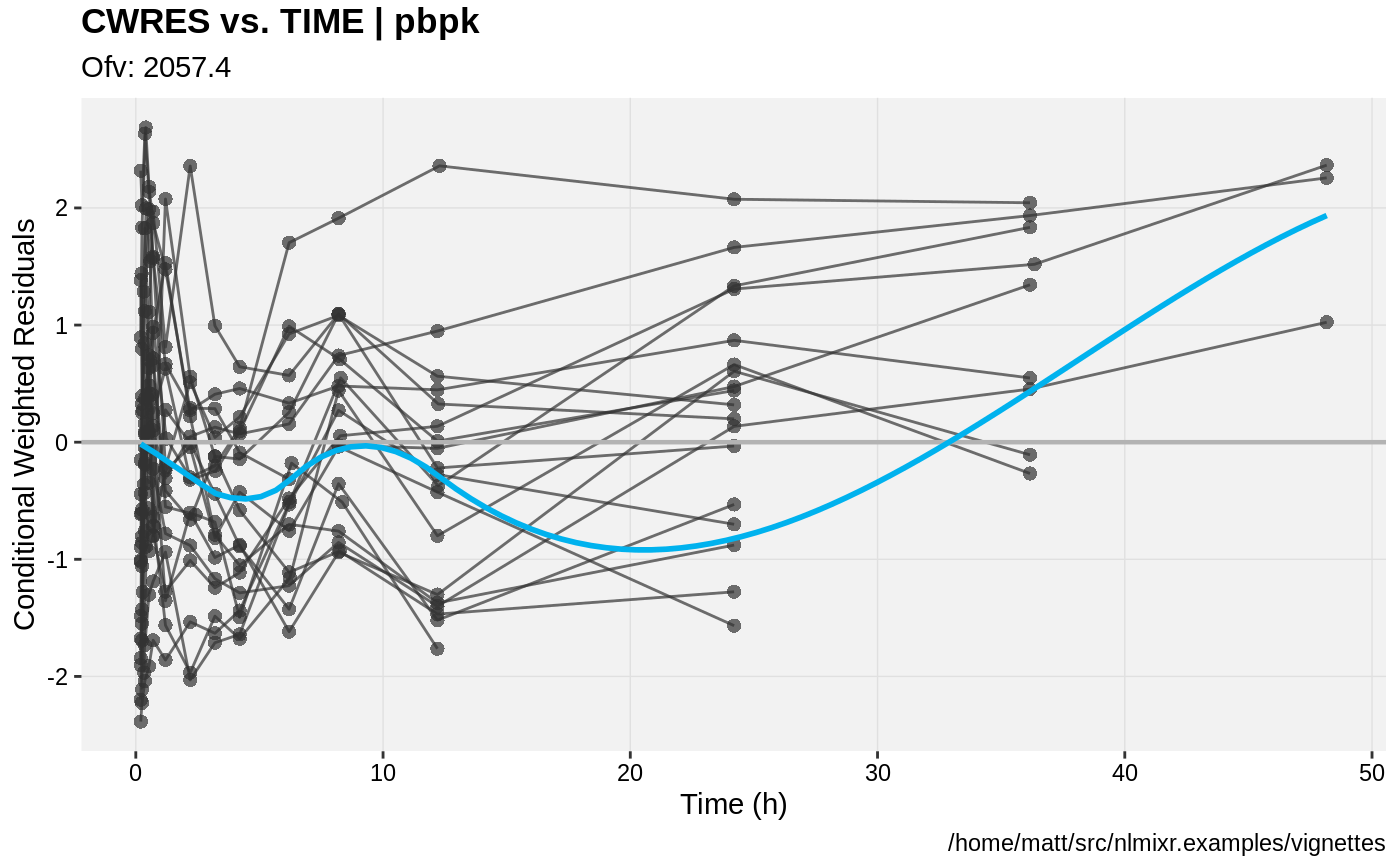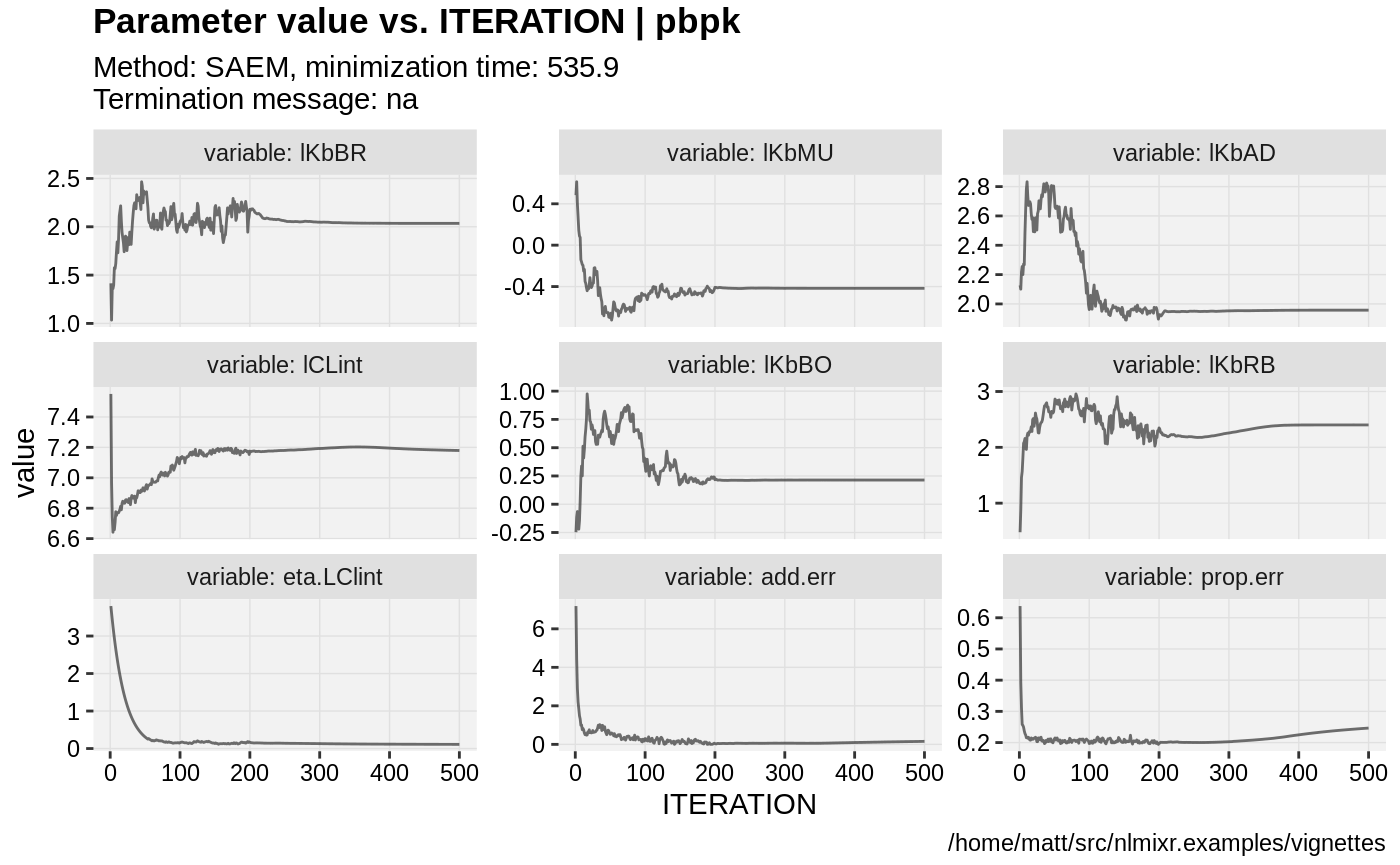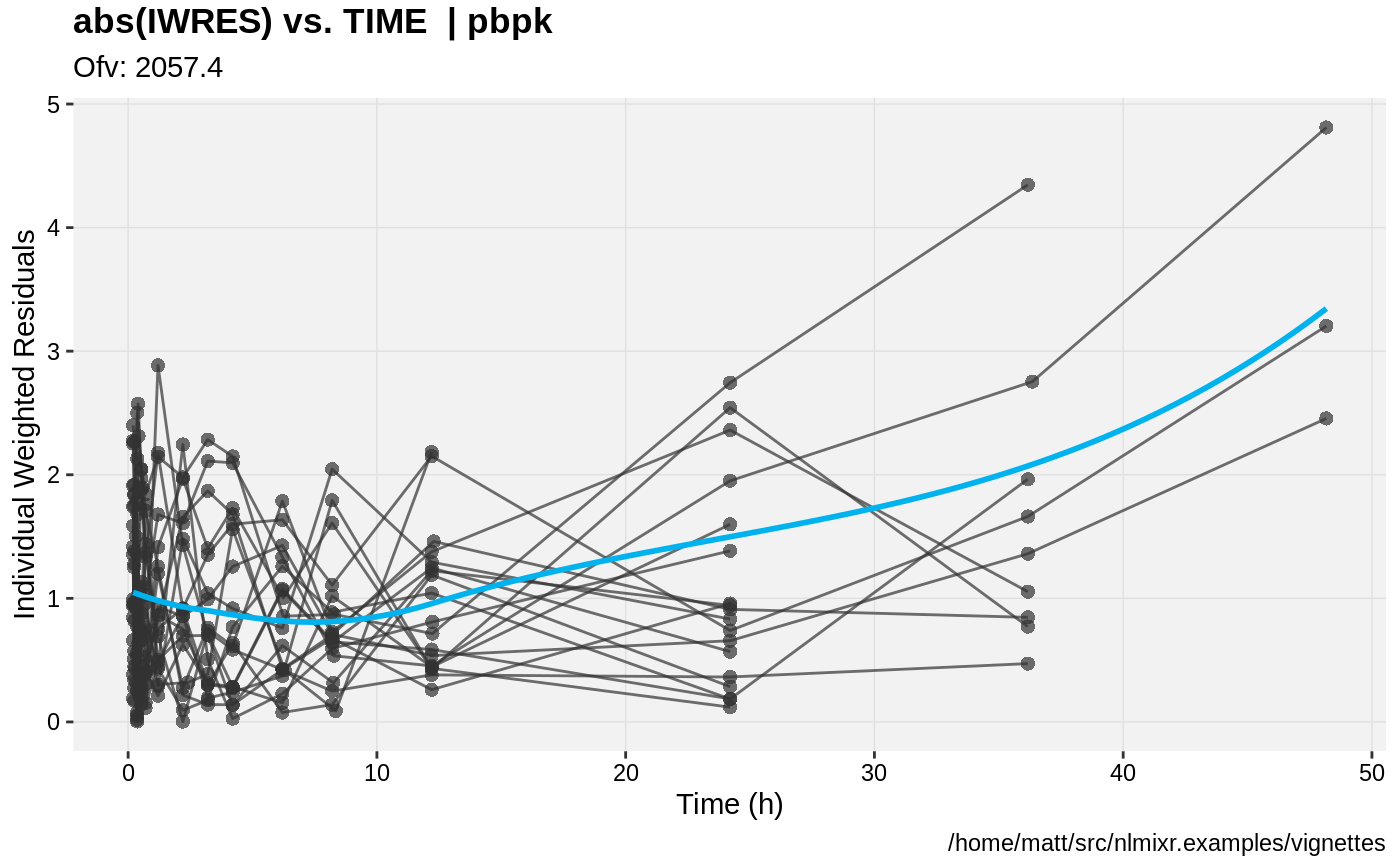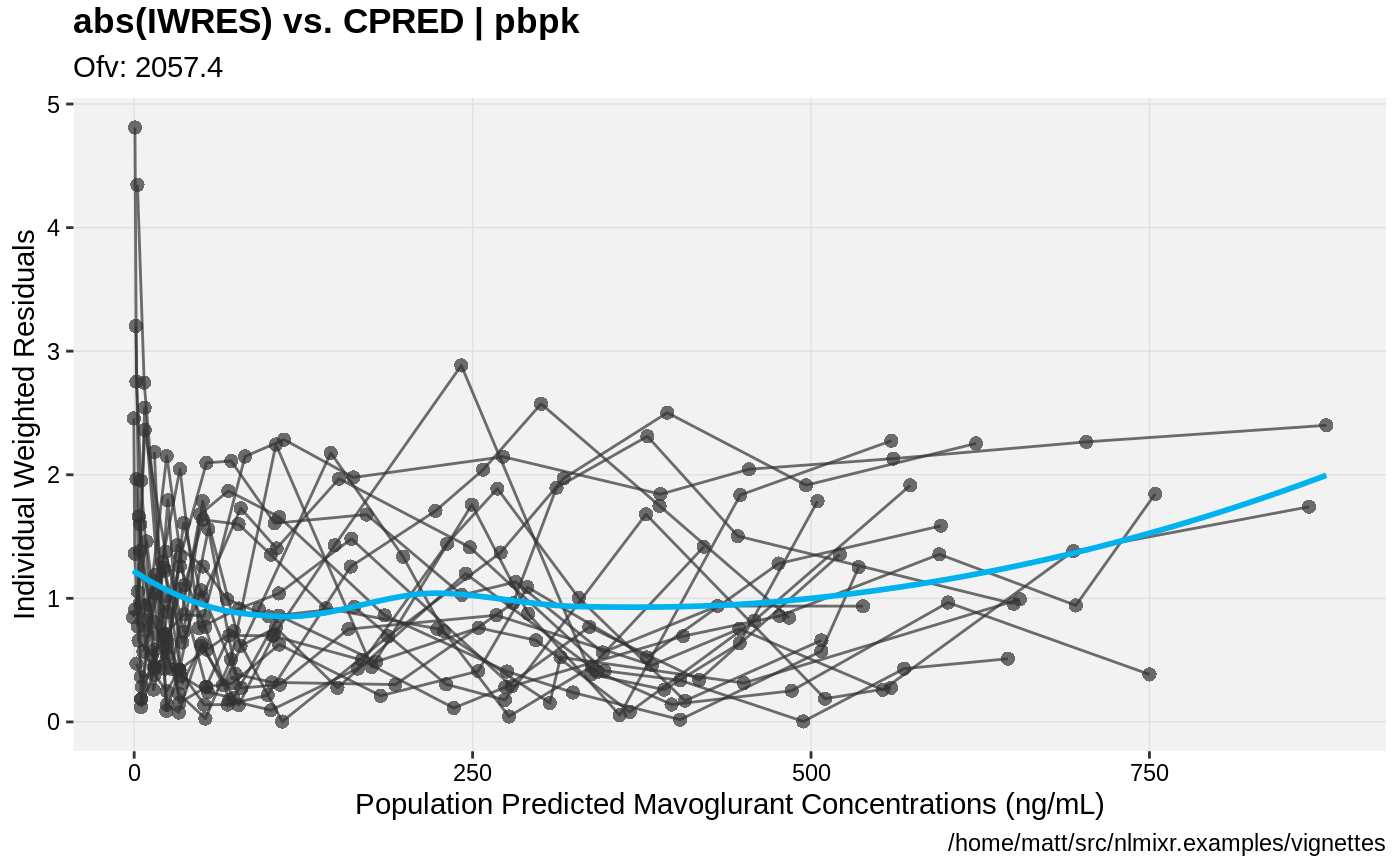#> Needed Covariates:
#>  "WT"
#> Calculating residuals/tables
#> done.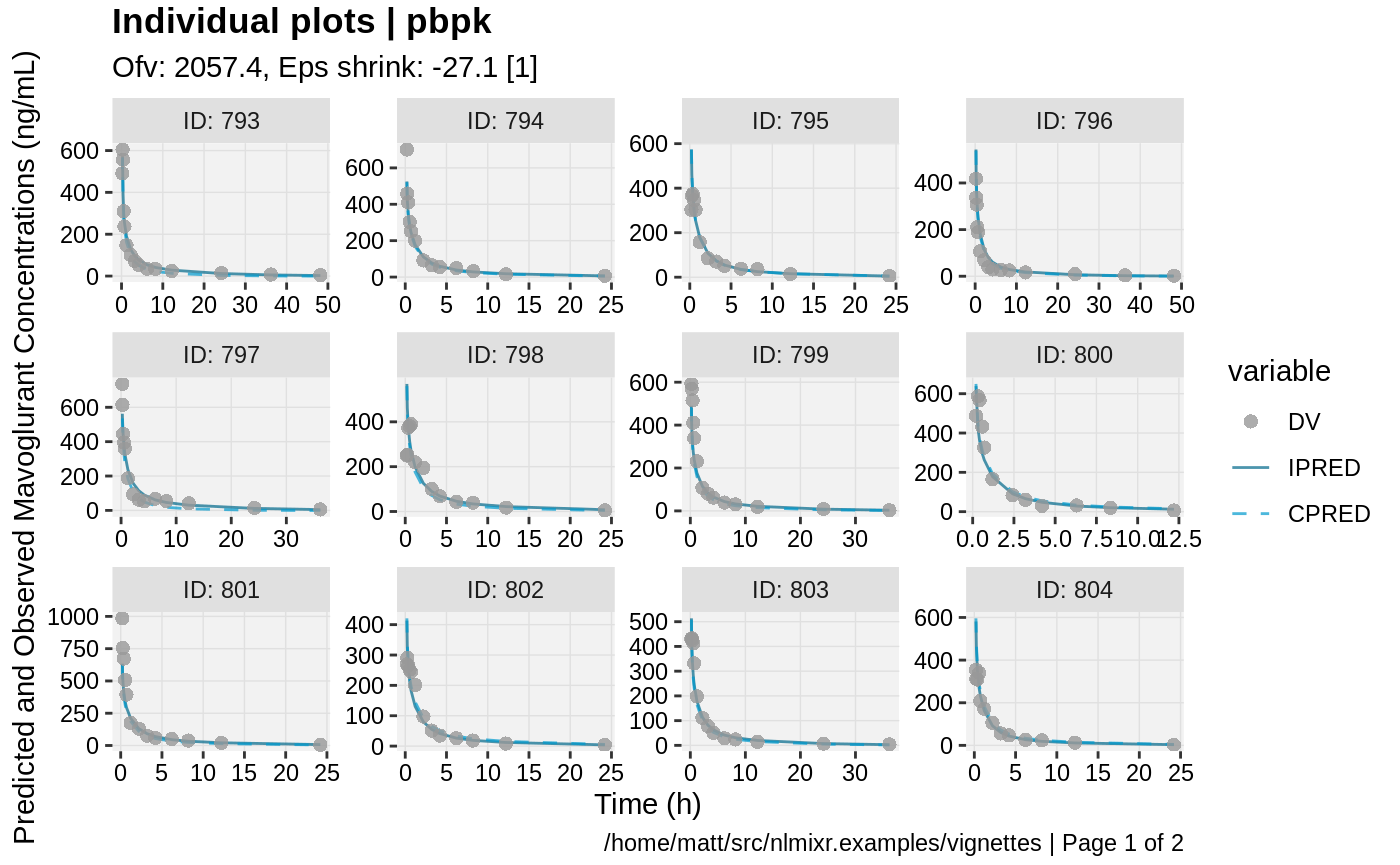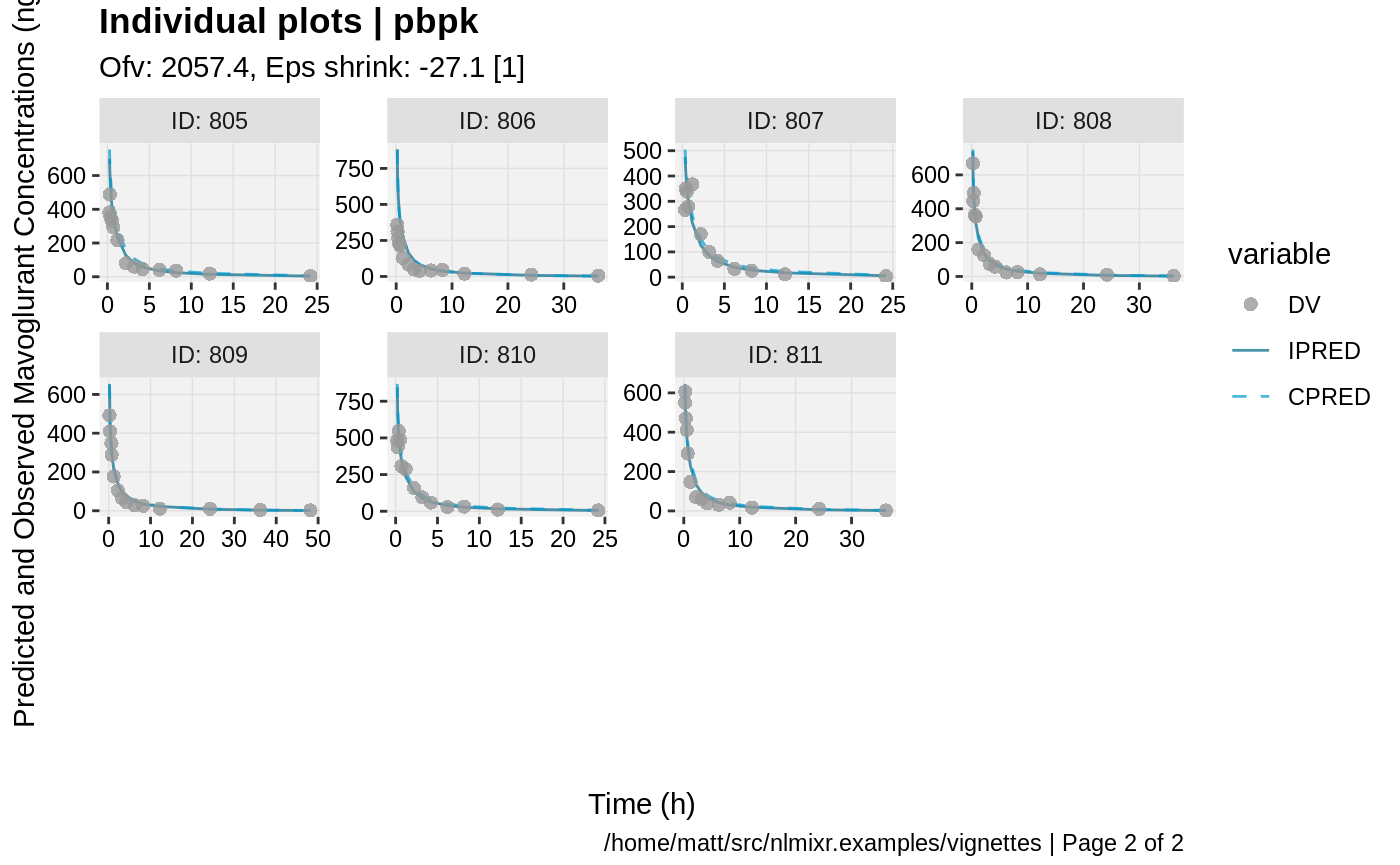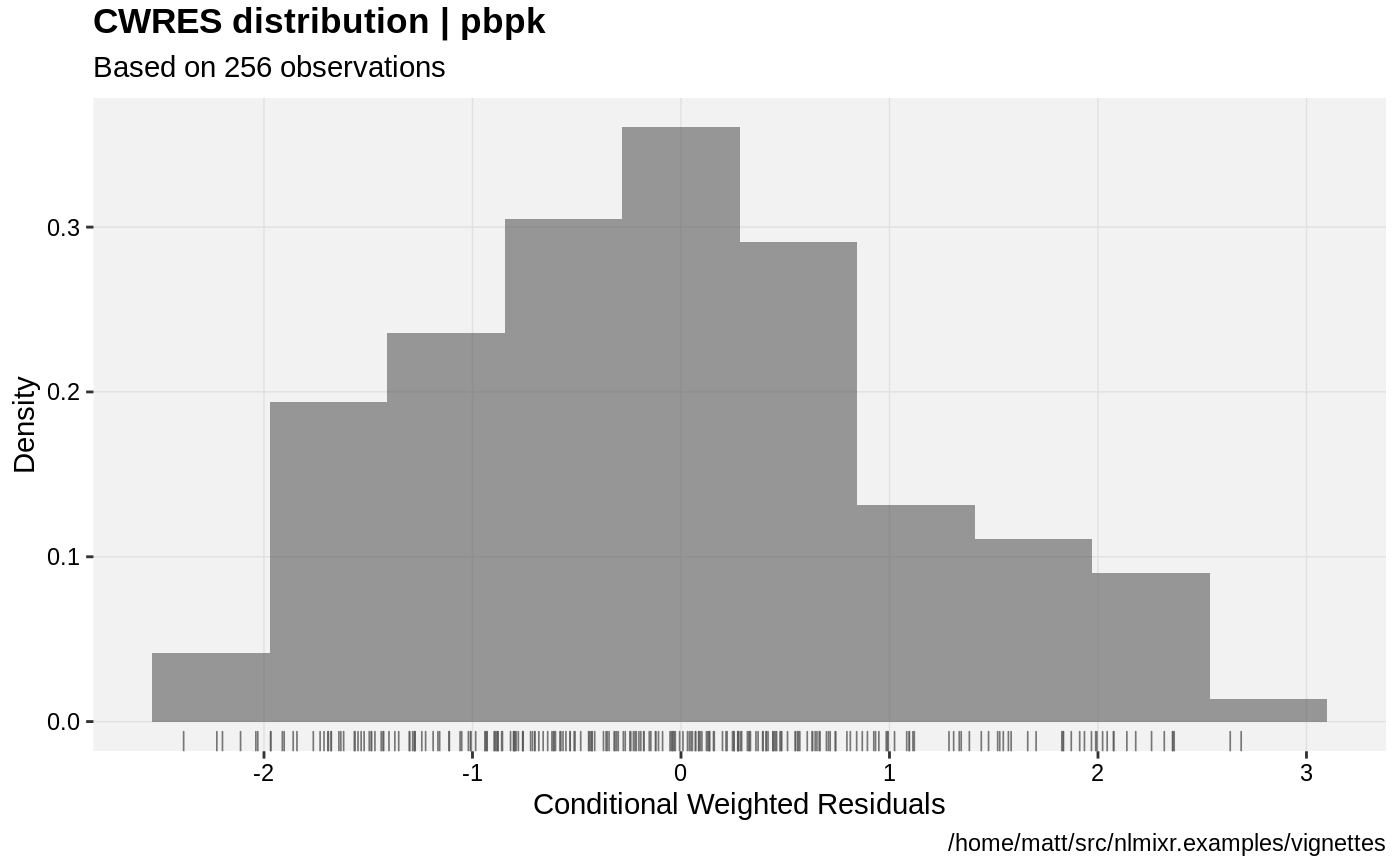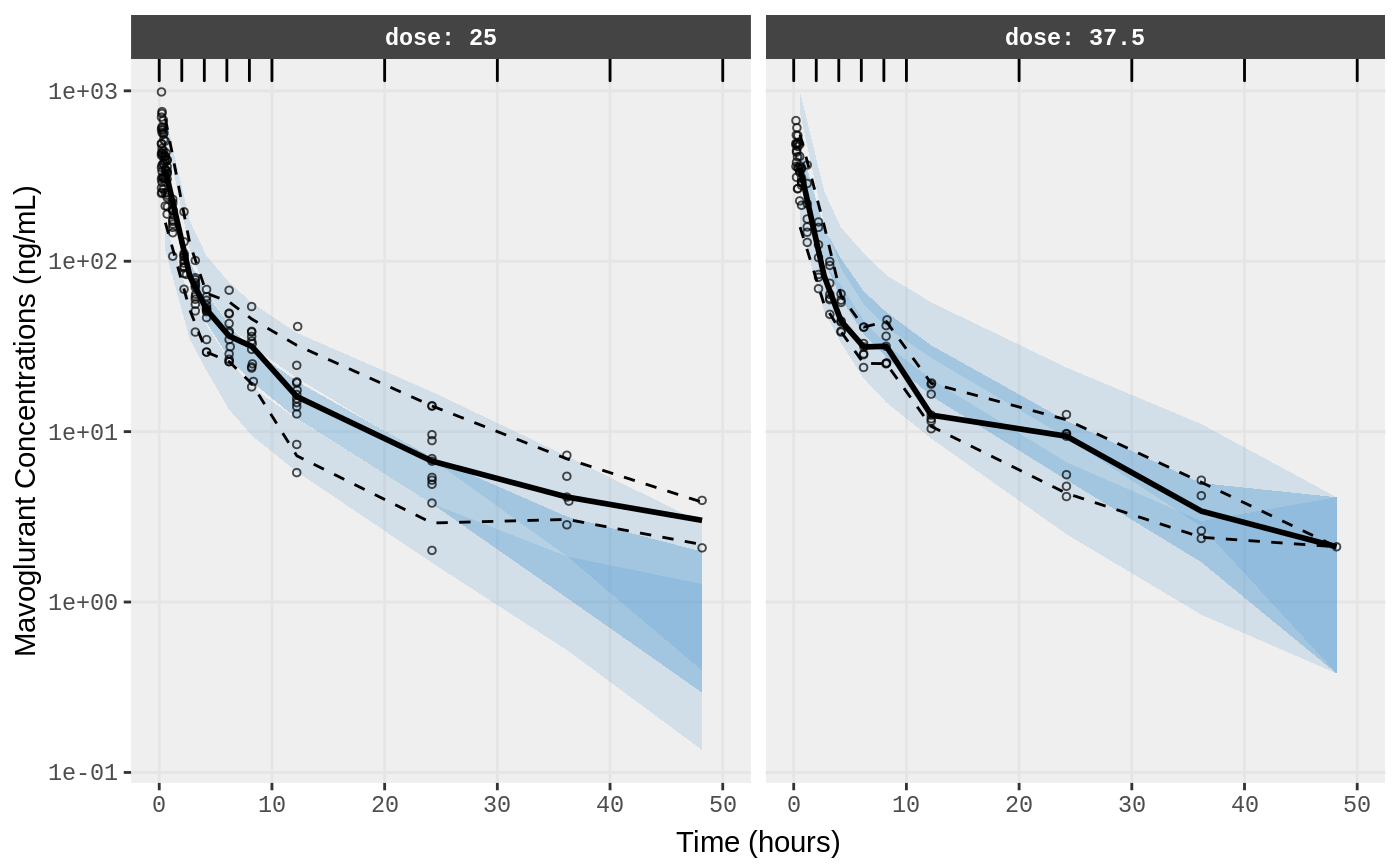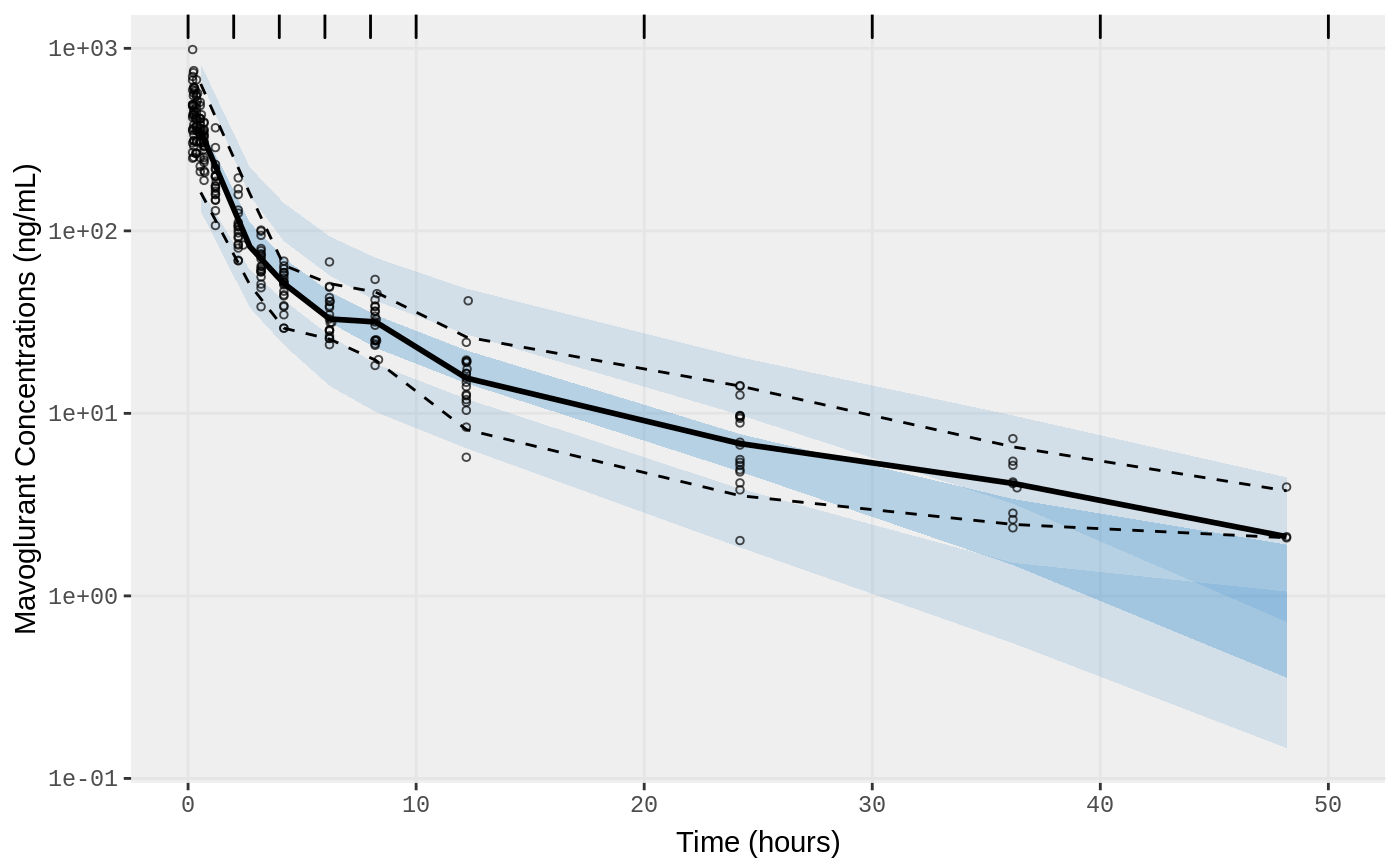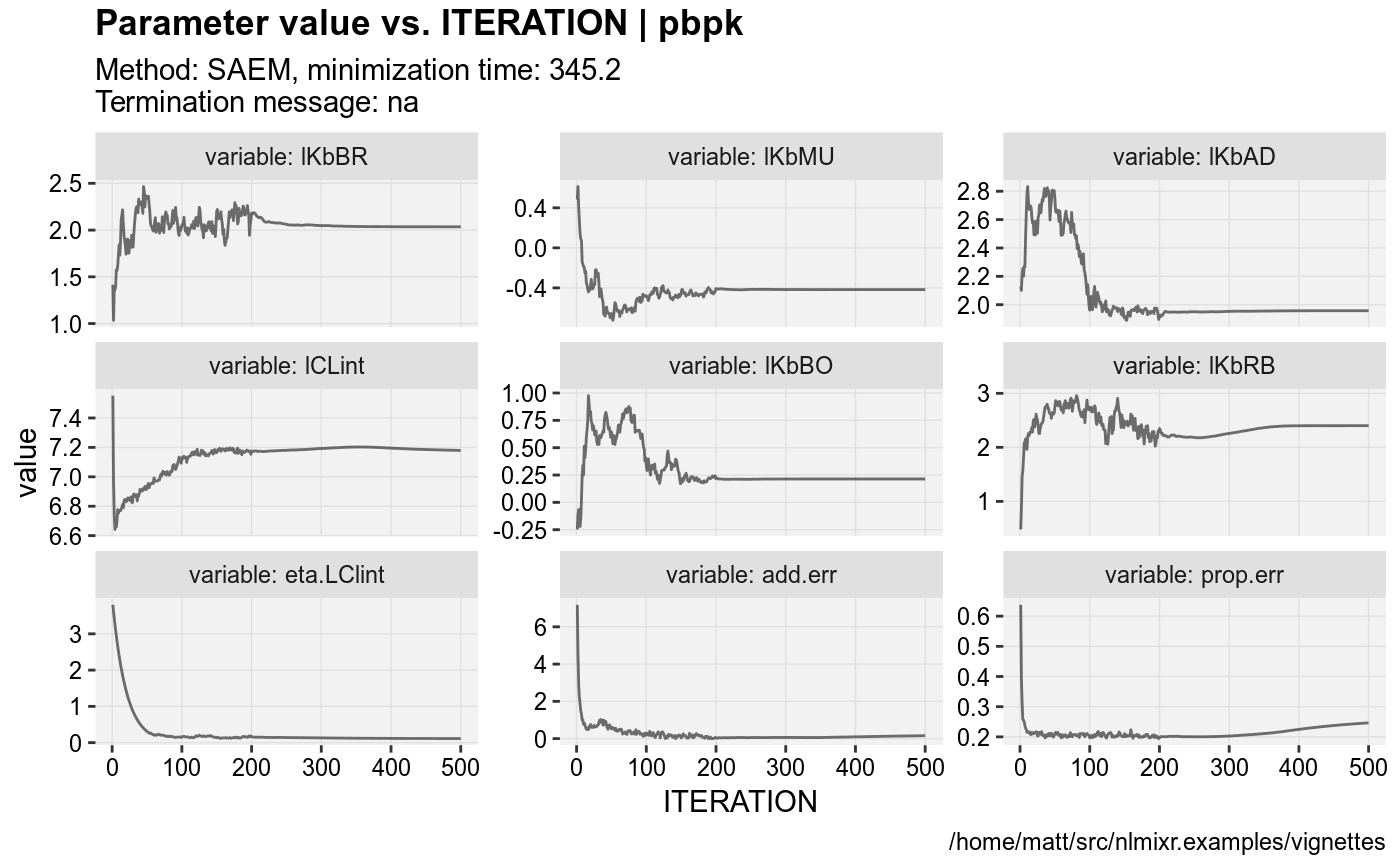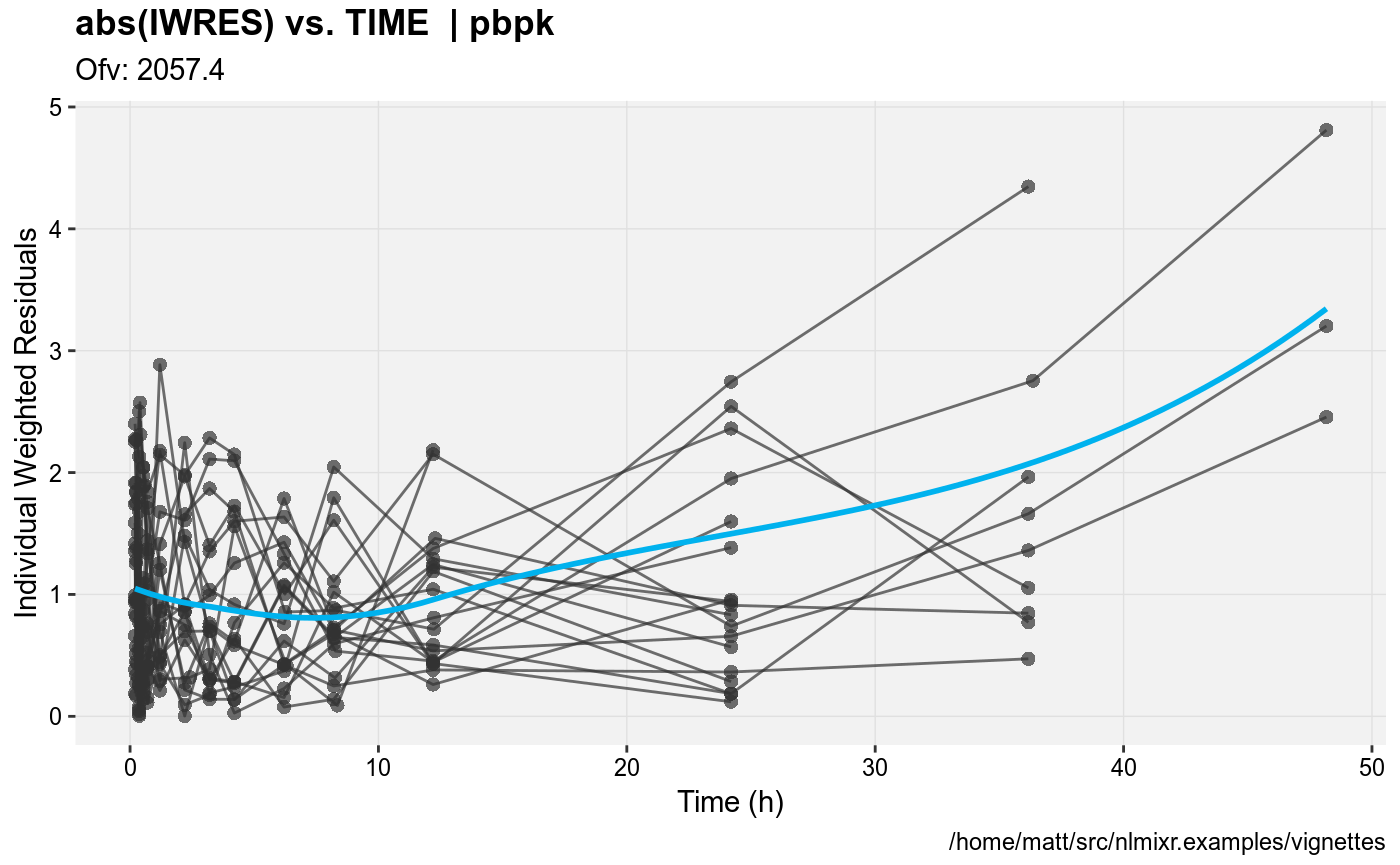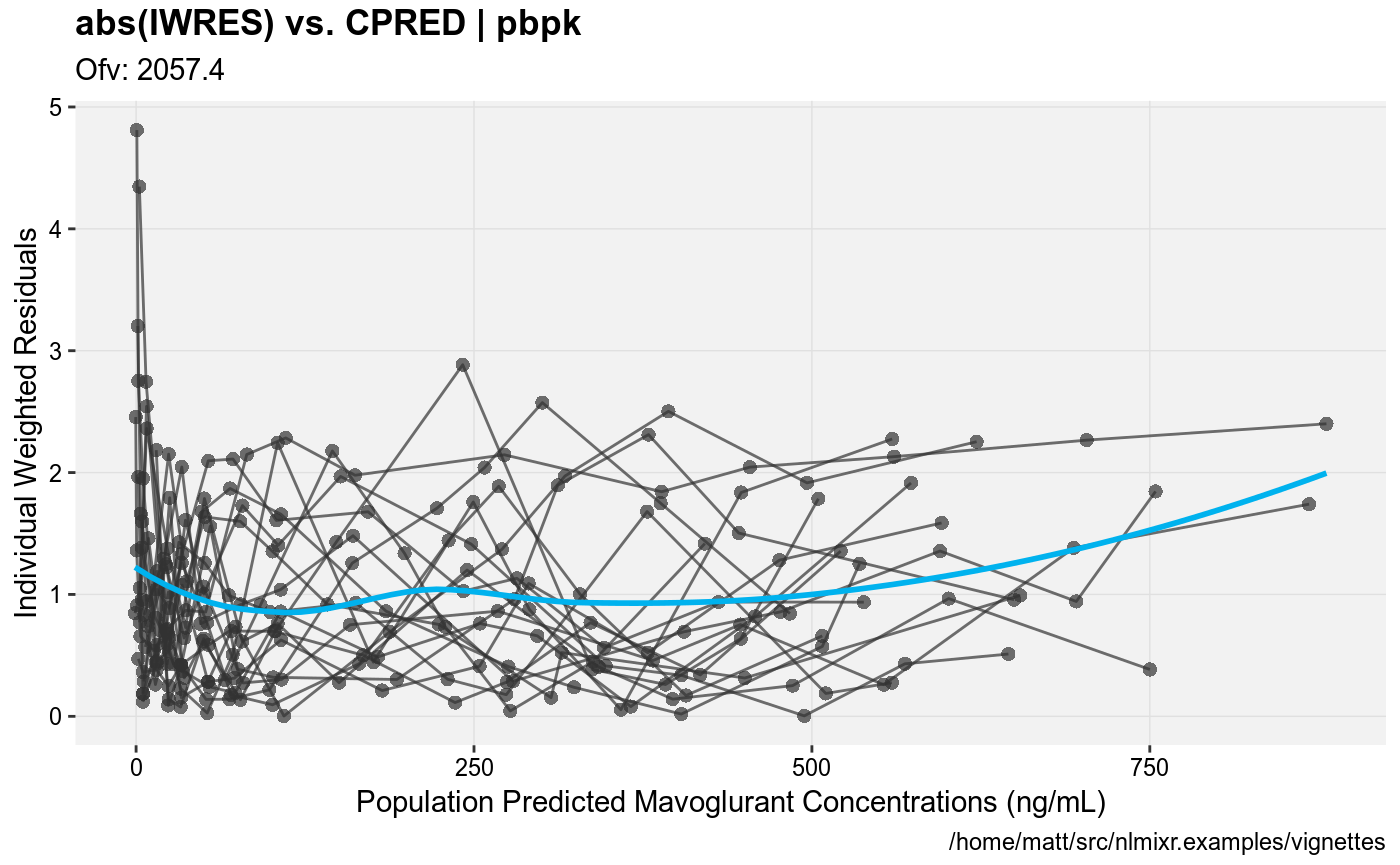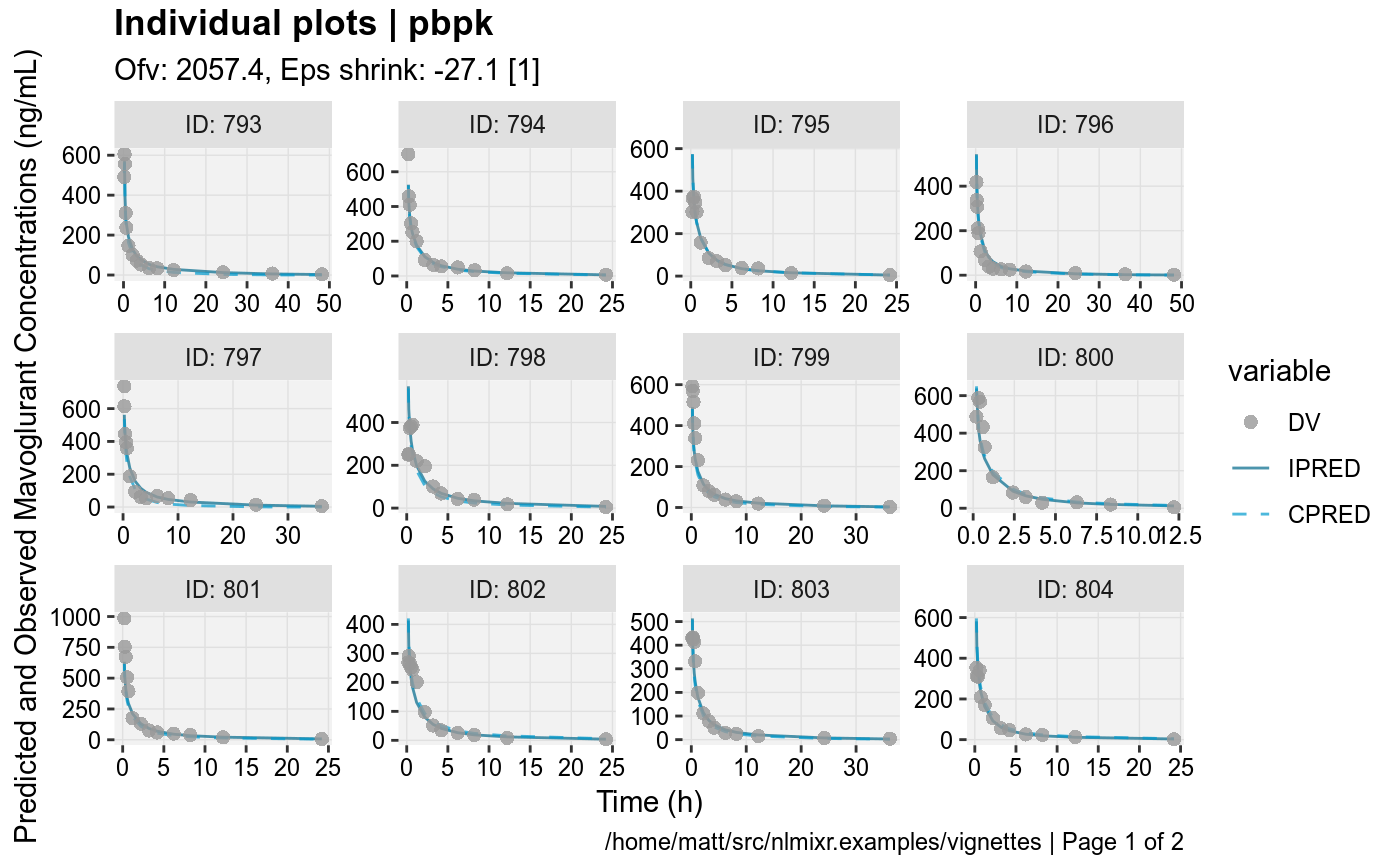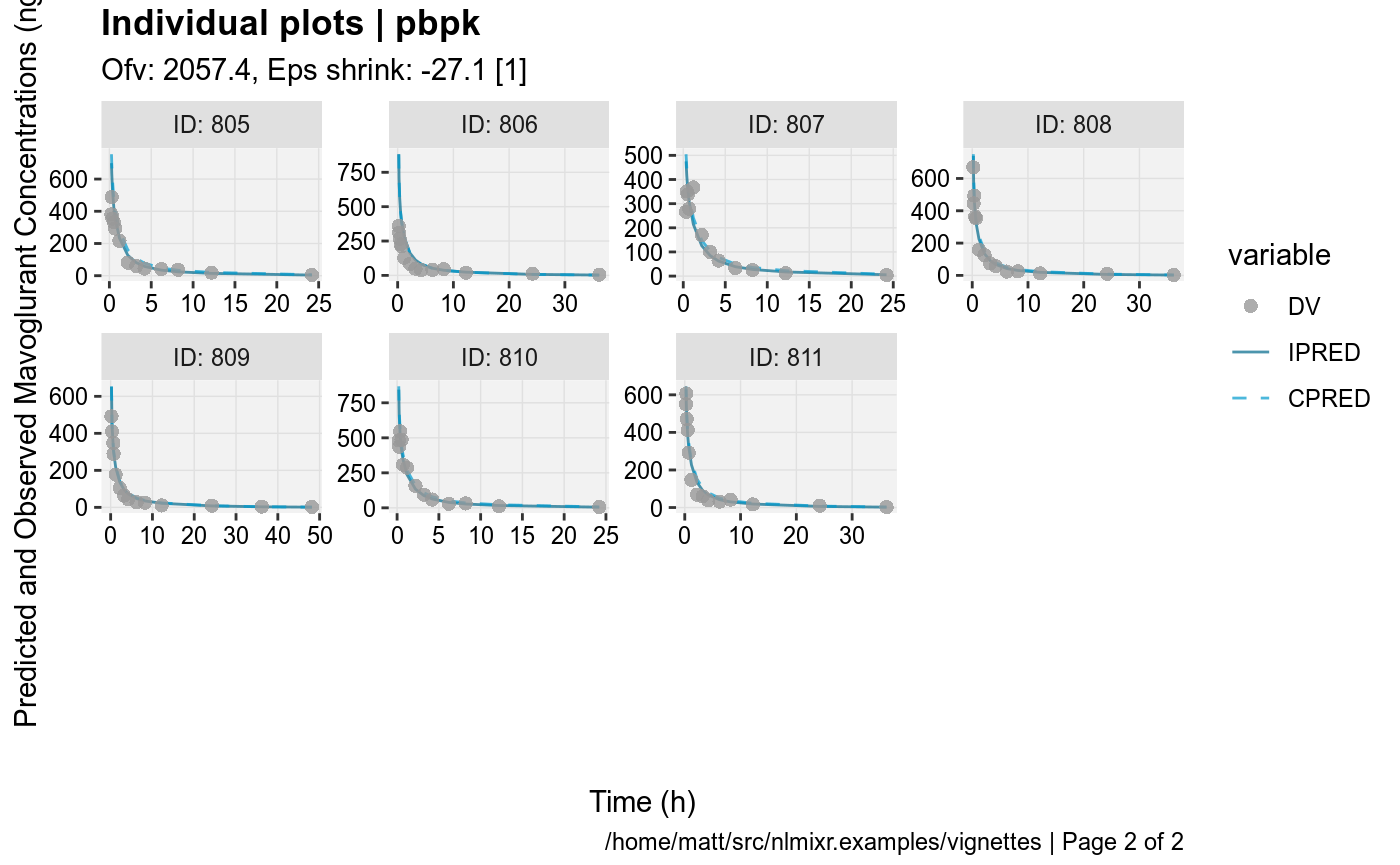#> Compiling VPC model...
#> done
#> done (4.10 sec)
#> Warning in data.frame(..., check.names = FALSE): row names were found from
#> a short variable and have been discarded
#> Compiling VPC model...
#> done
#> done (4.47 sec)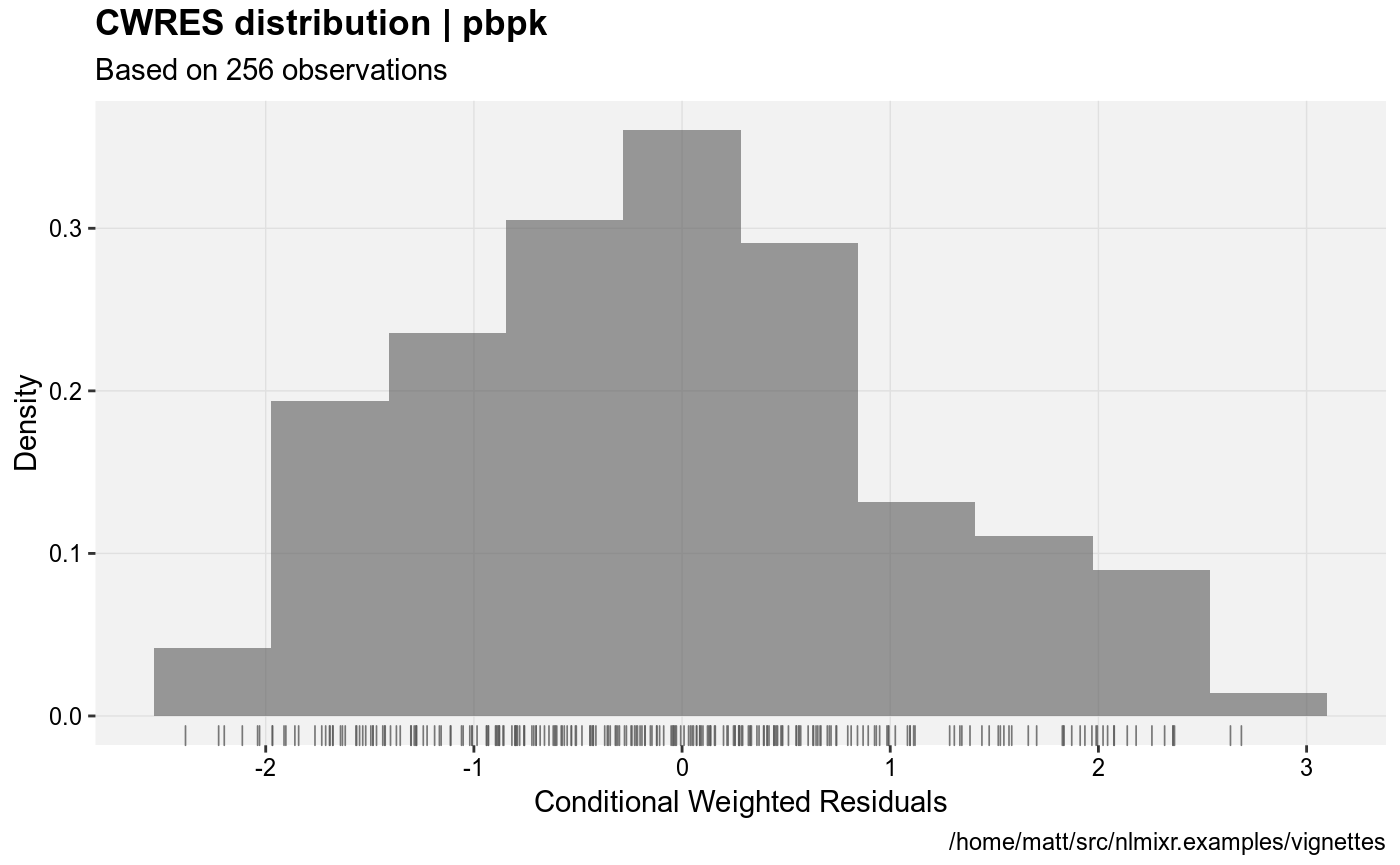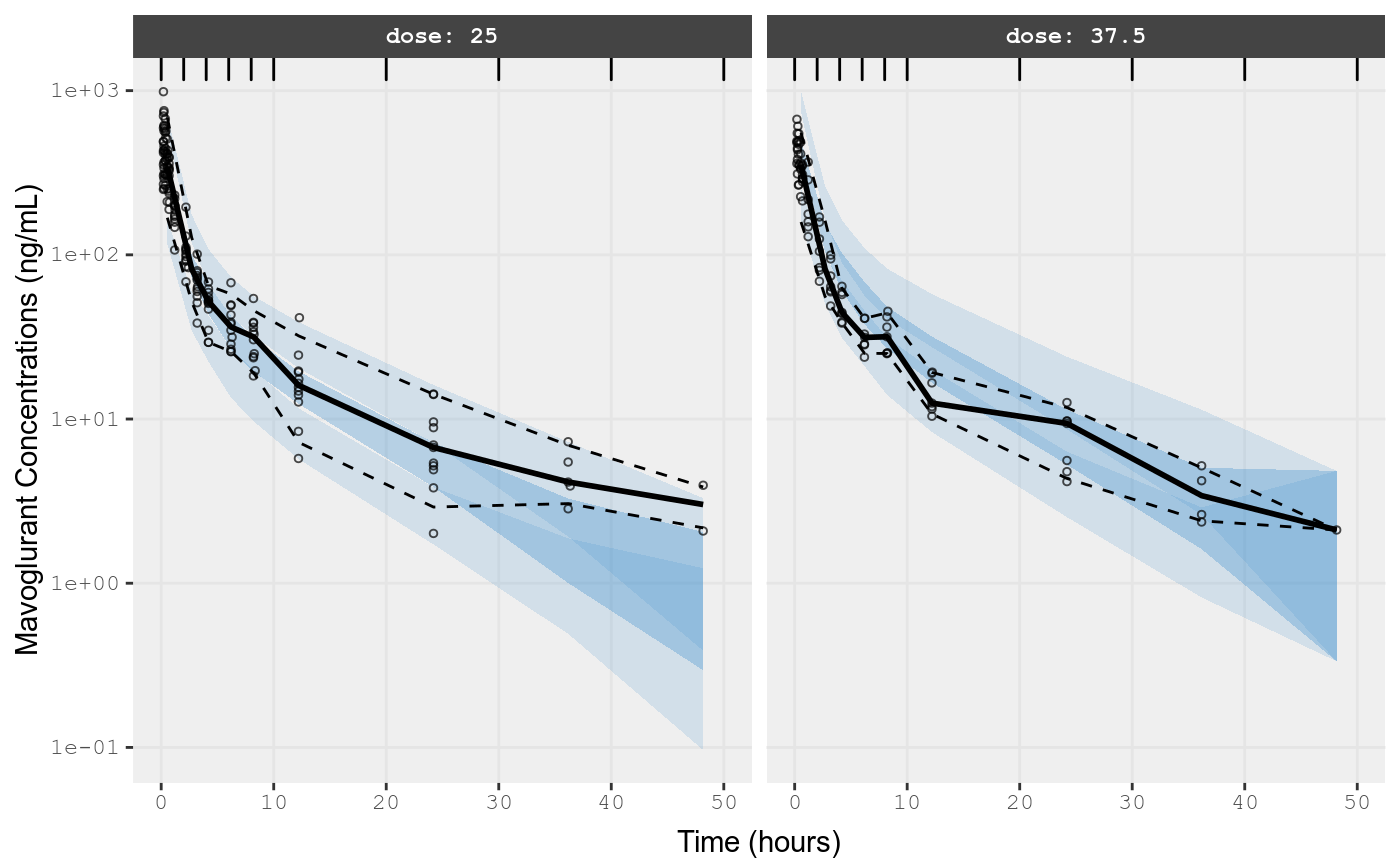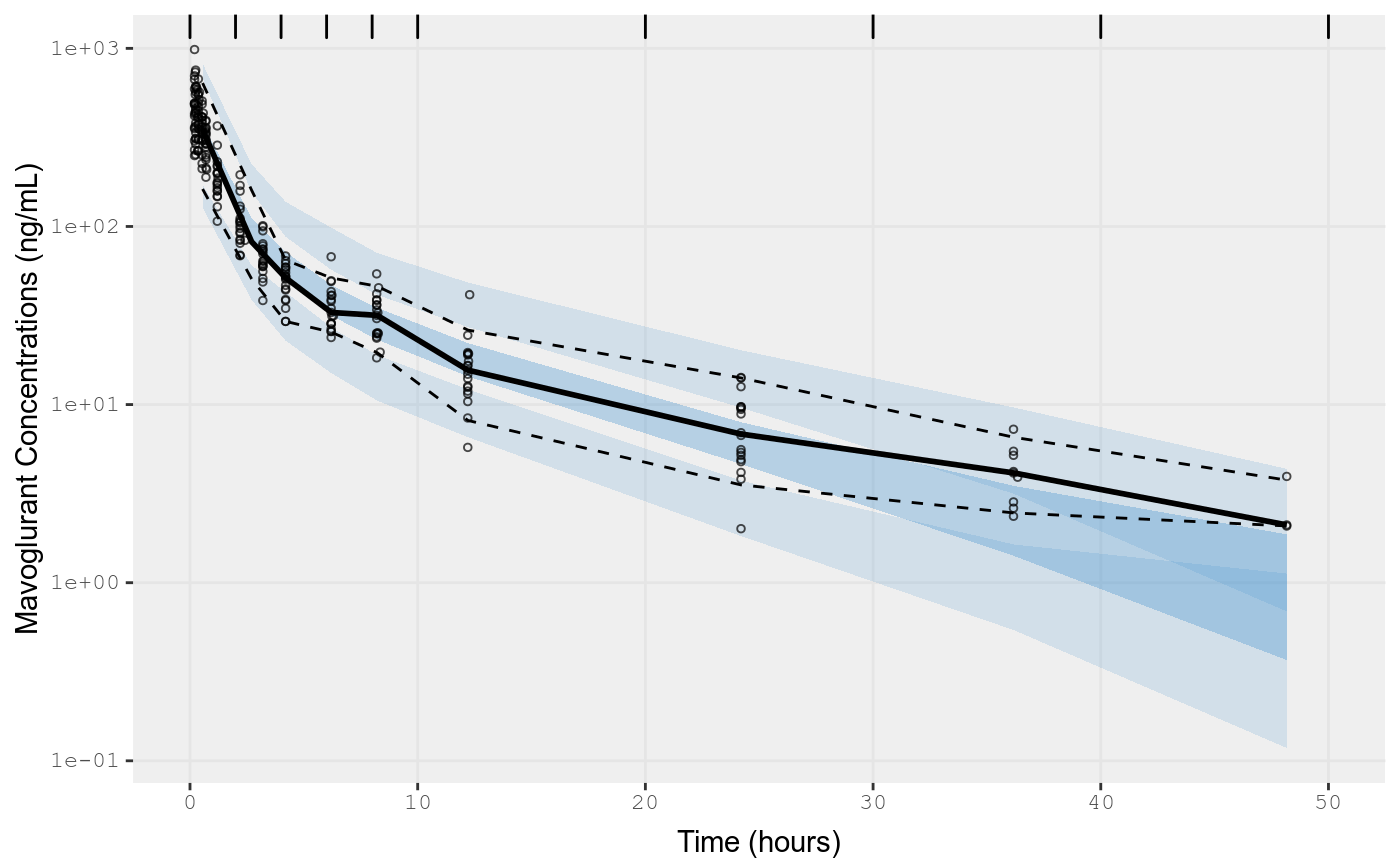## Change error to lognormal

fit.lnorm.S <- pbpk %>%
model({C15 ~ lnorm(lnorm.err)}) %>% # Change C15 to be log-normally distributed
nlmixr(dat,est="saem") # Requires data since pining from pbpk model
#> Loading model already run (/home/matt/R/x86_64-pc-linux-gnu-library/3.5/nlmixr.examples/nlmixr-saem-c1f116cb15f985d19f0fcfc6ca548931.rds)

NOTE: lognormal distribution AIC/loglik/etc is on normal scale. Therefore, you can compare the AICs between fit.lnorm and fit.addProp since they are calculated on the same scale.

In this case you can see that the AIC for the log-normal model is better than the AIC for the addProp model.

gofs(fit.lnorm.S);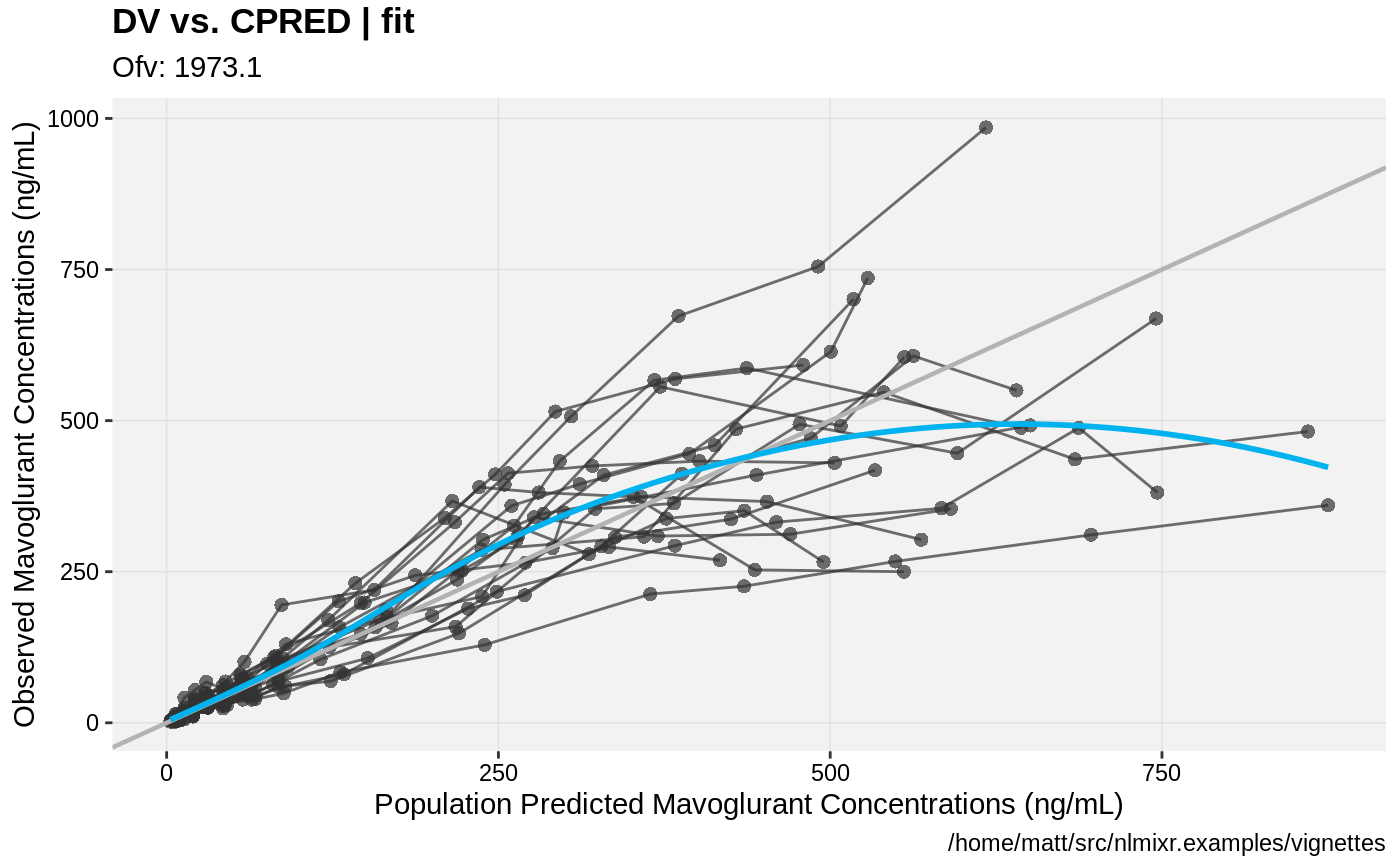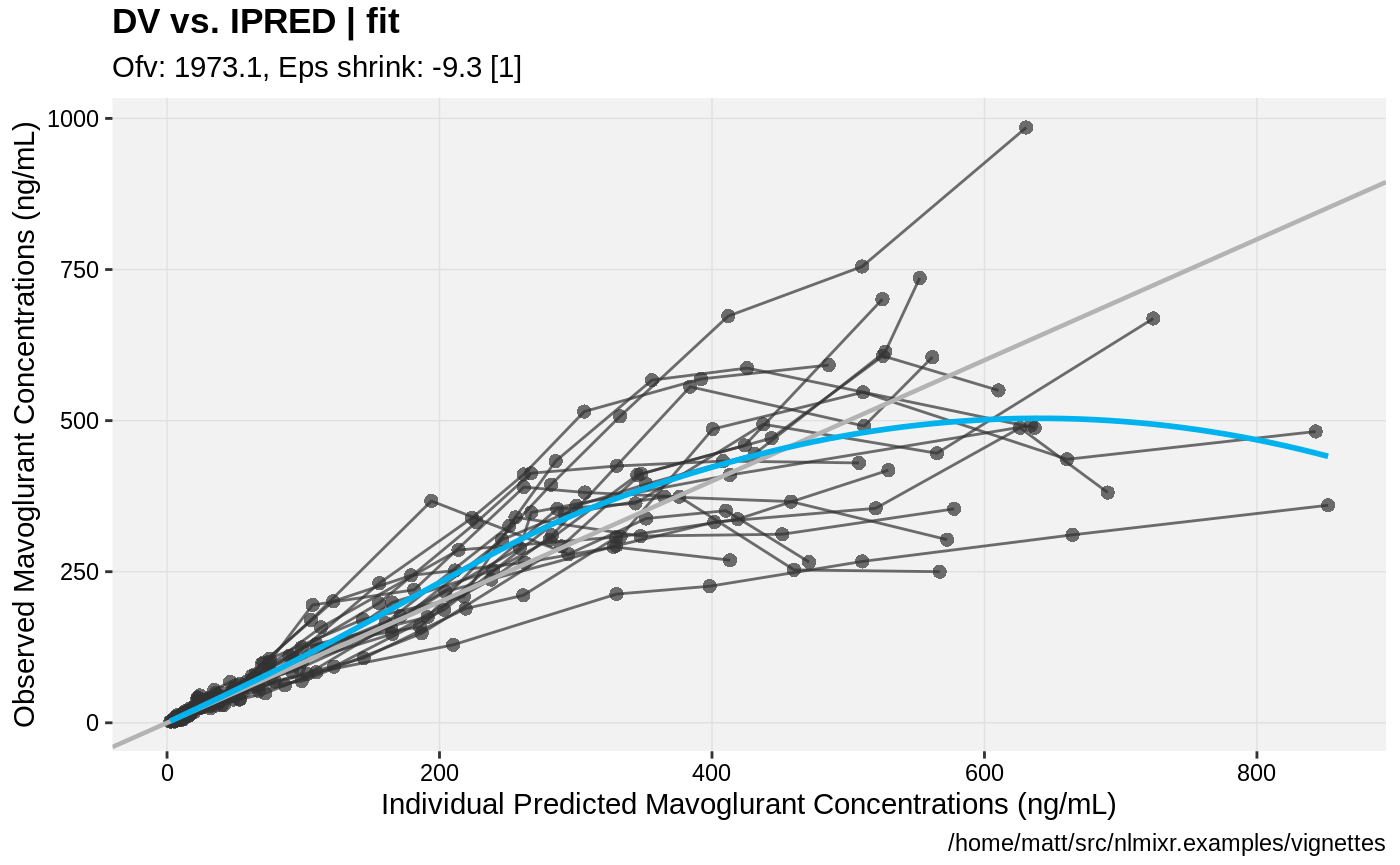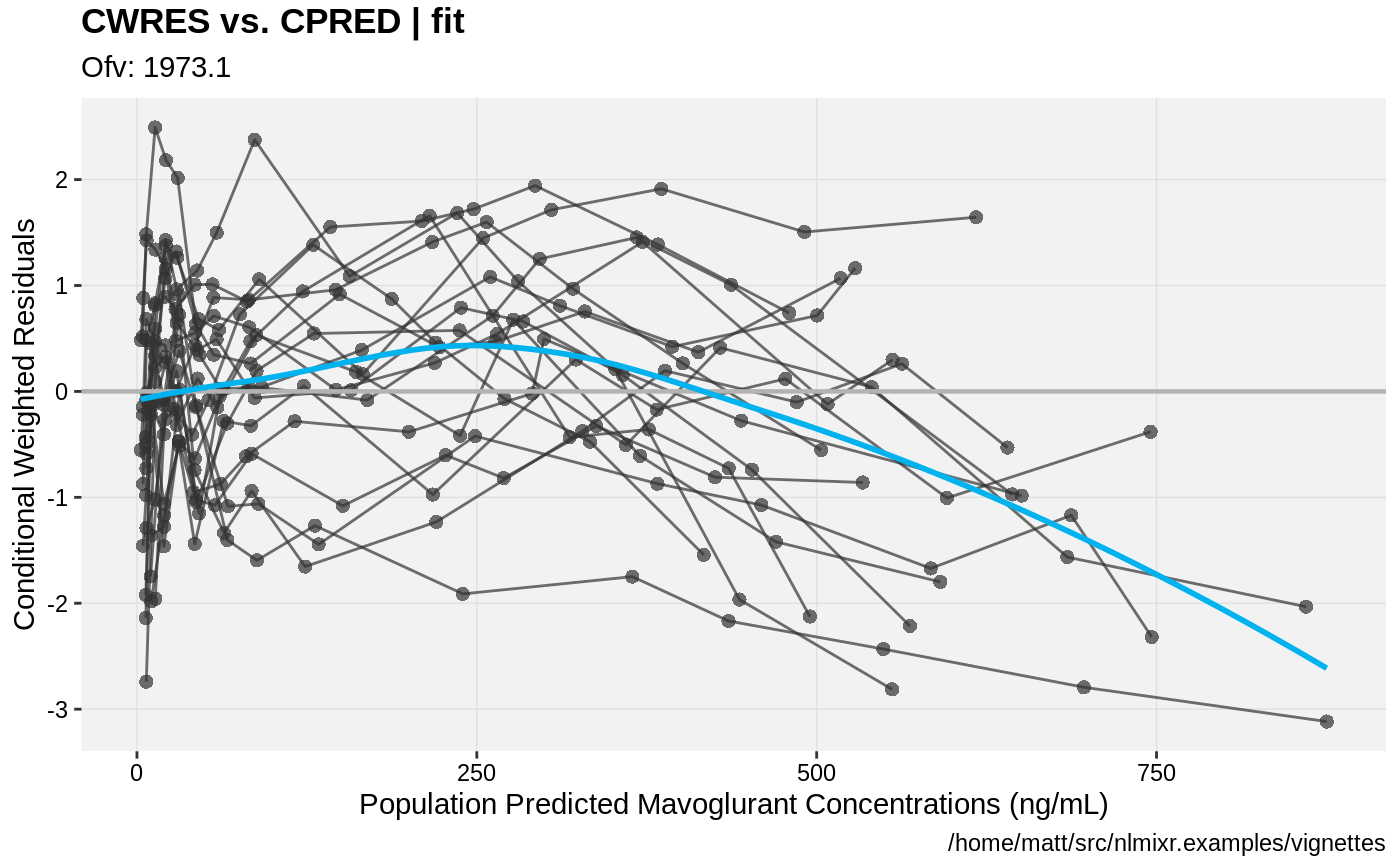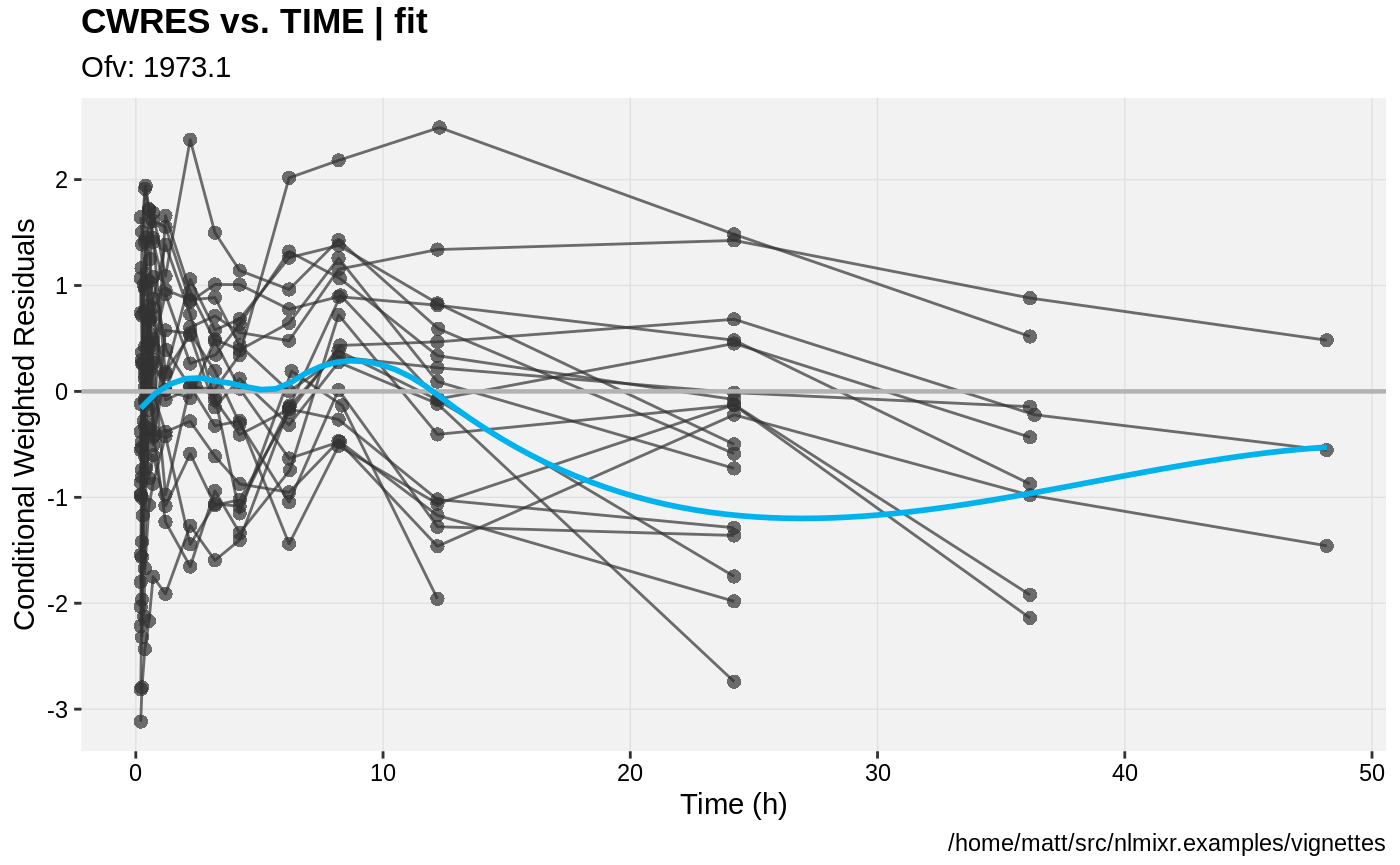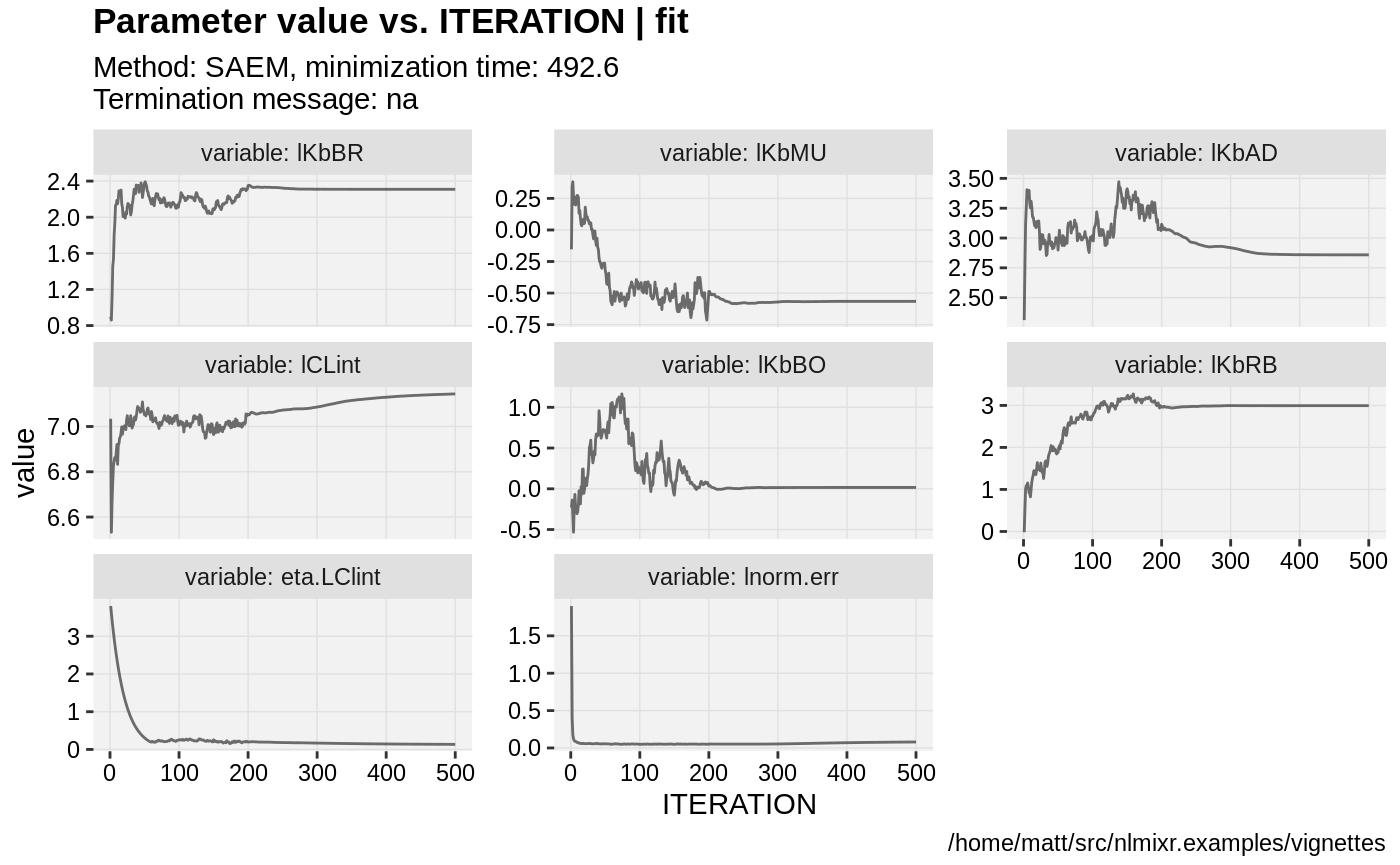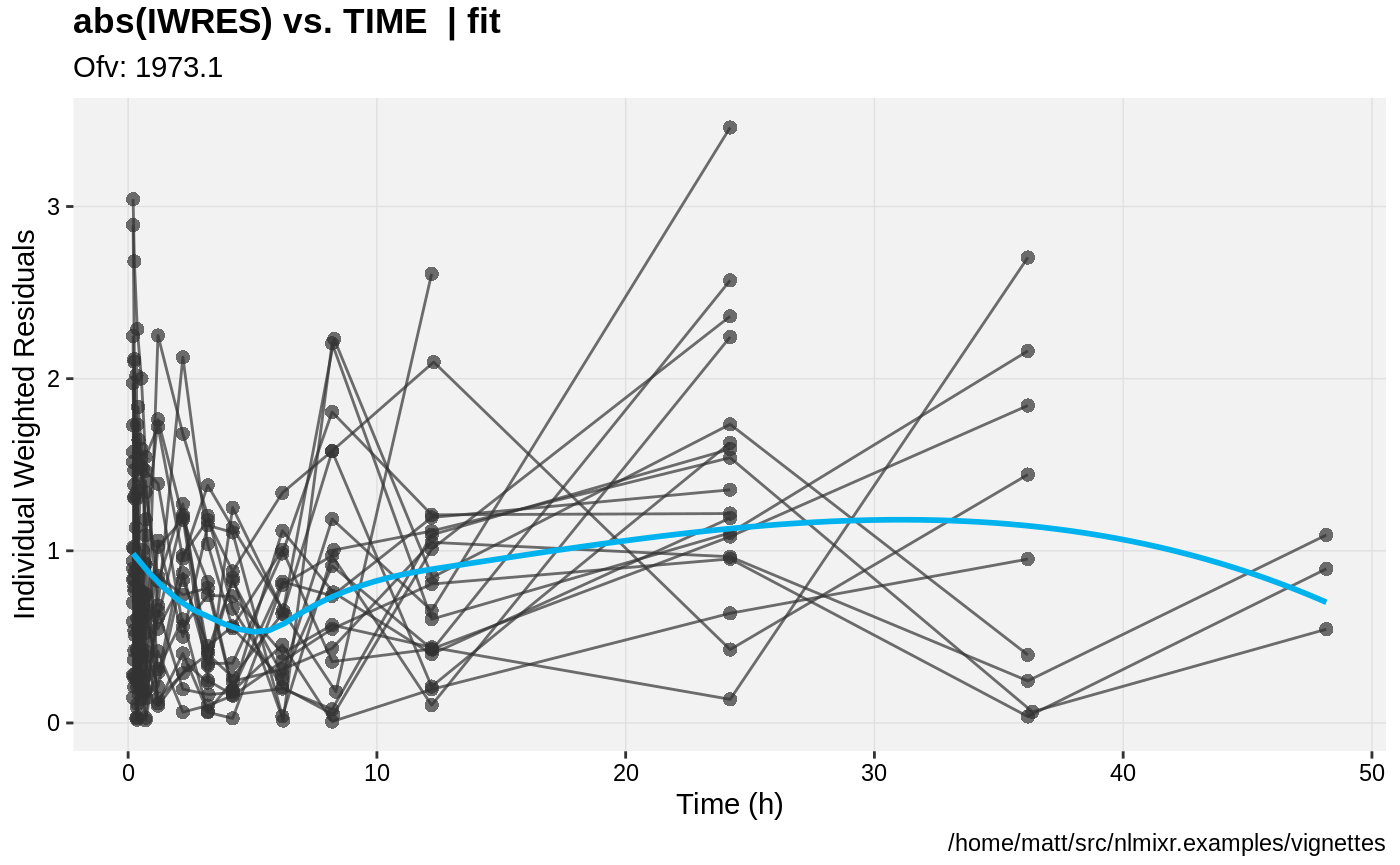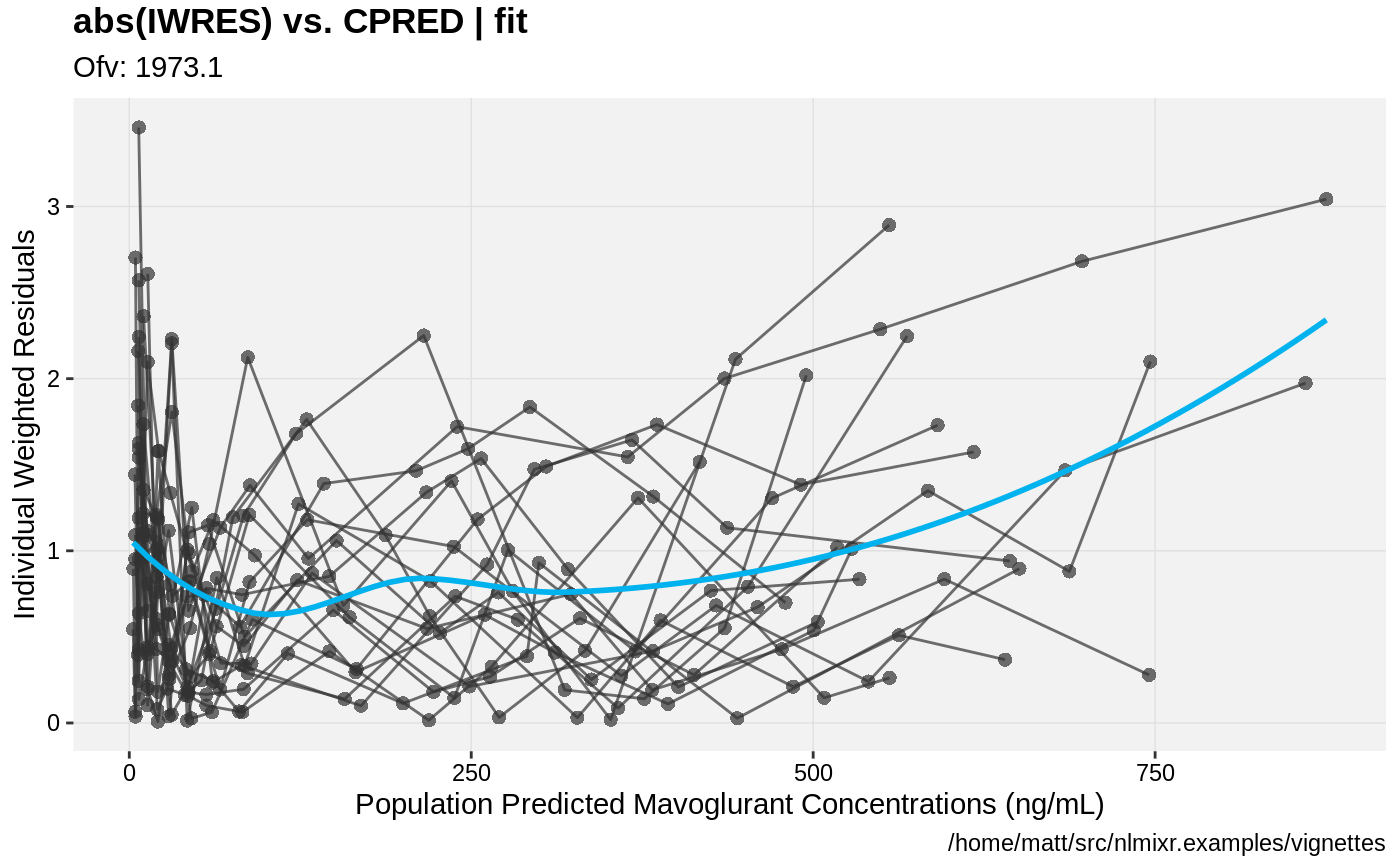#> Needed Covariates:
#>  "WT"
#> Calculating residuals/tables
#> done.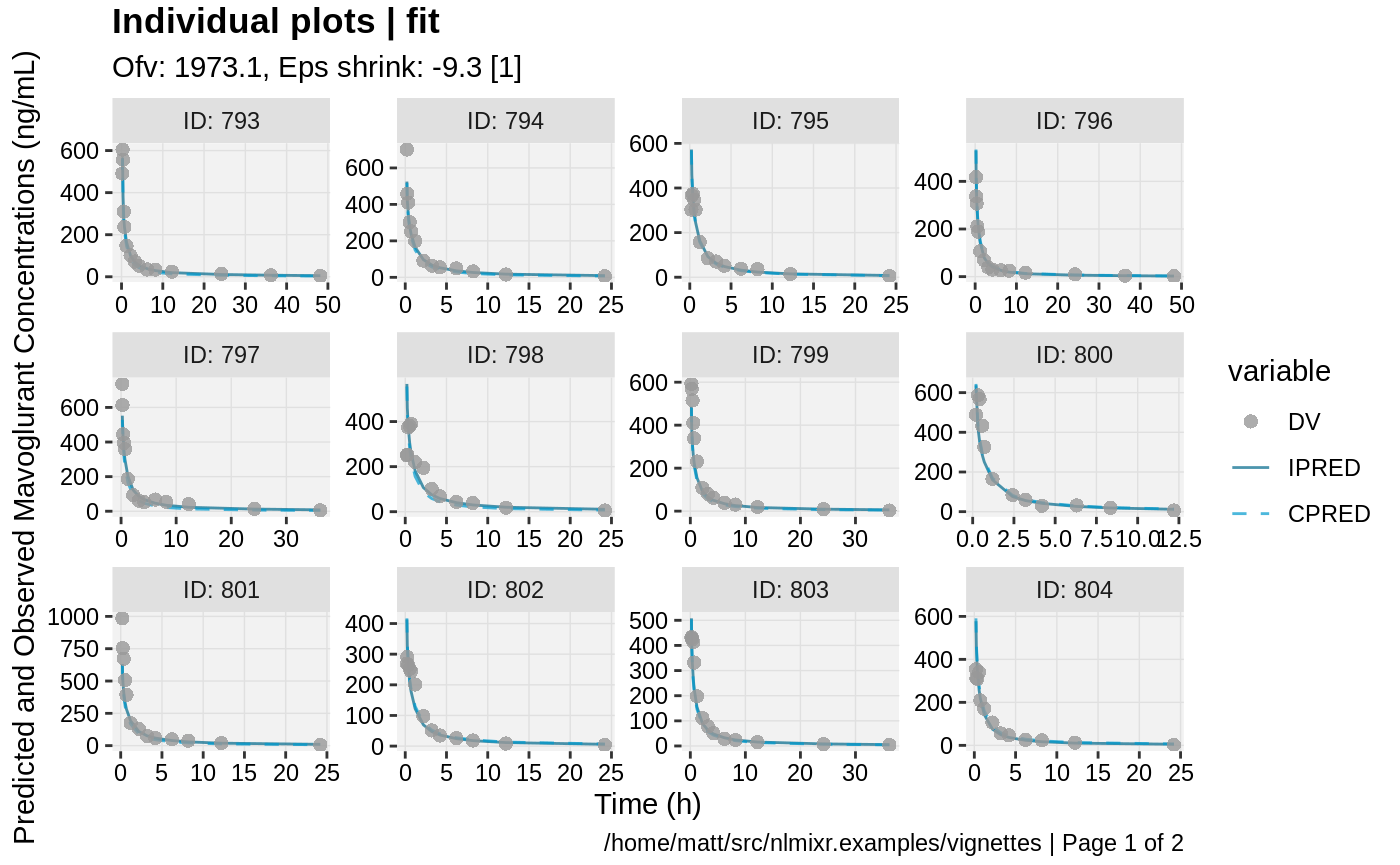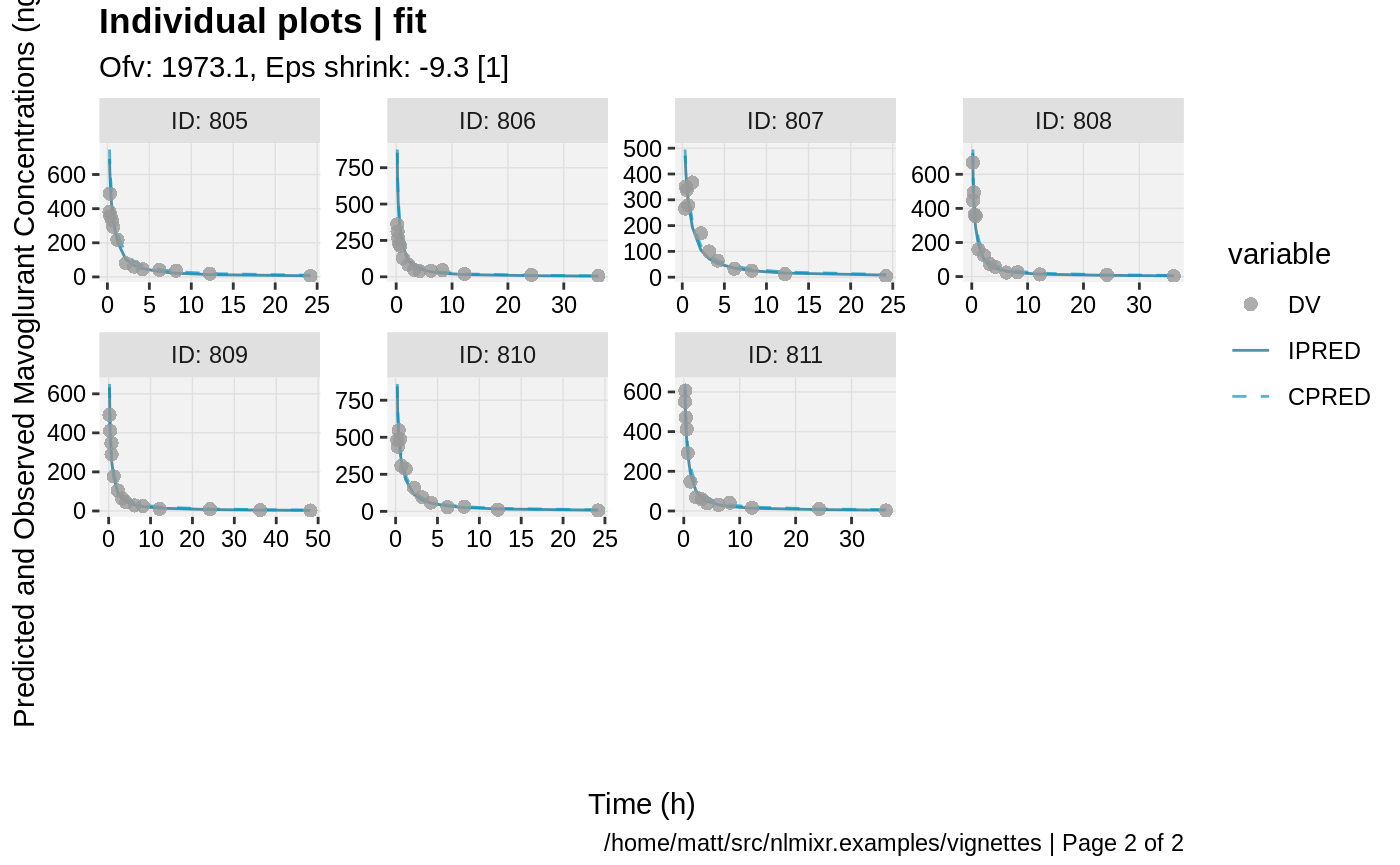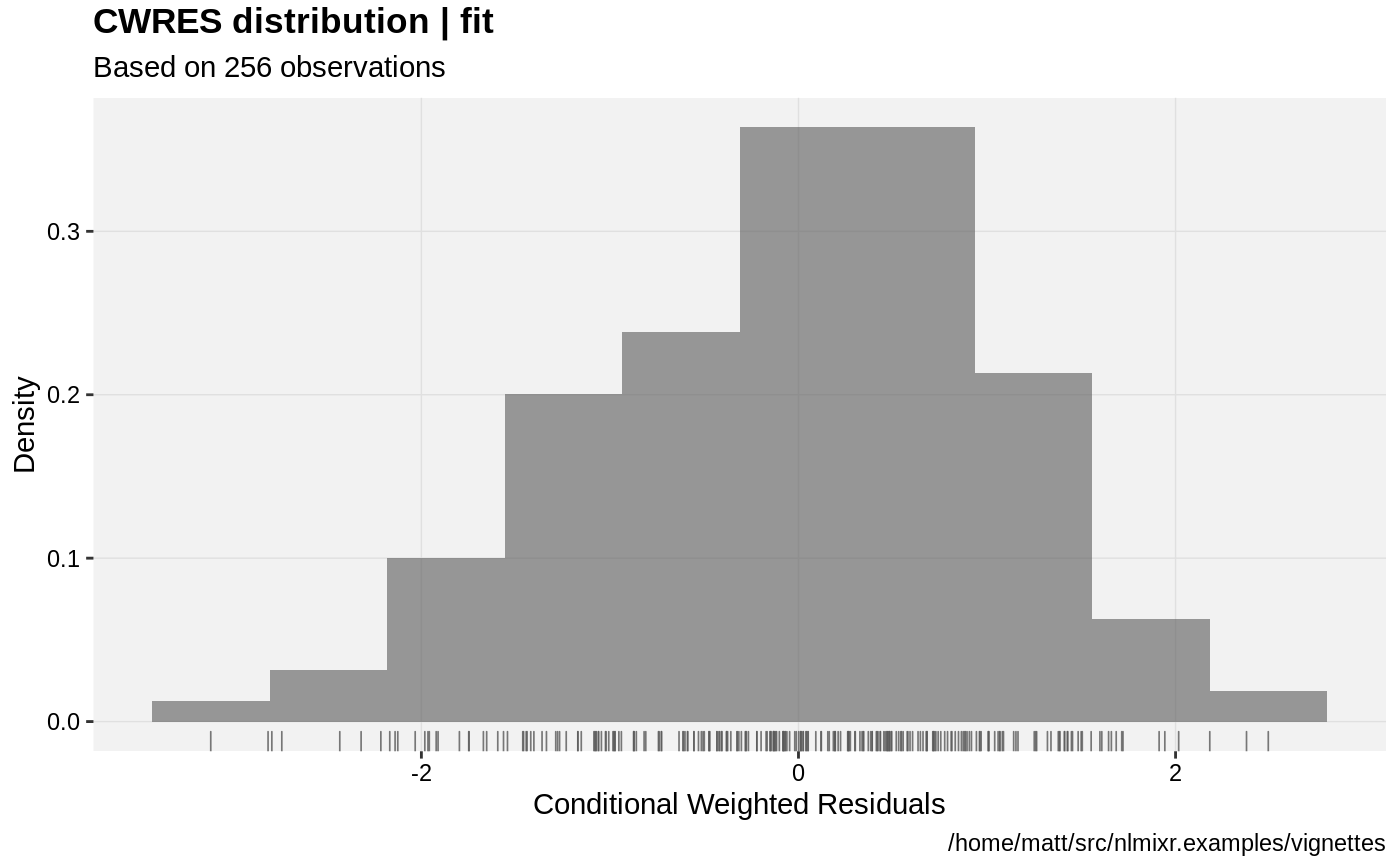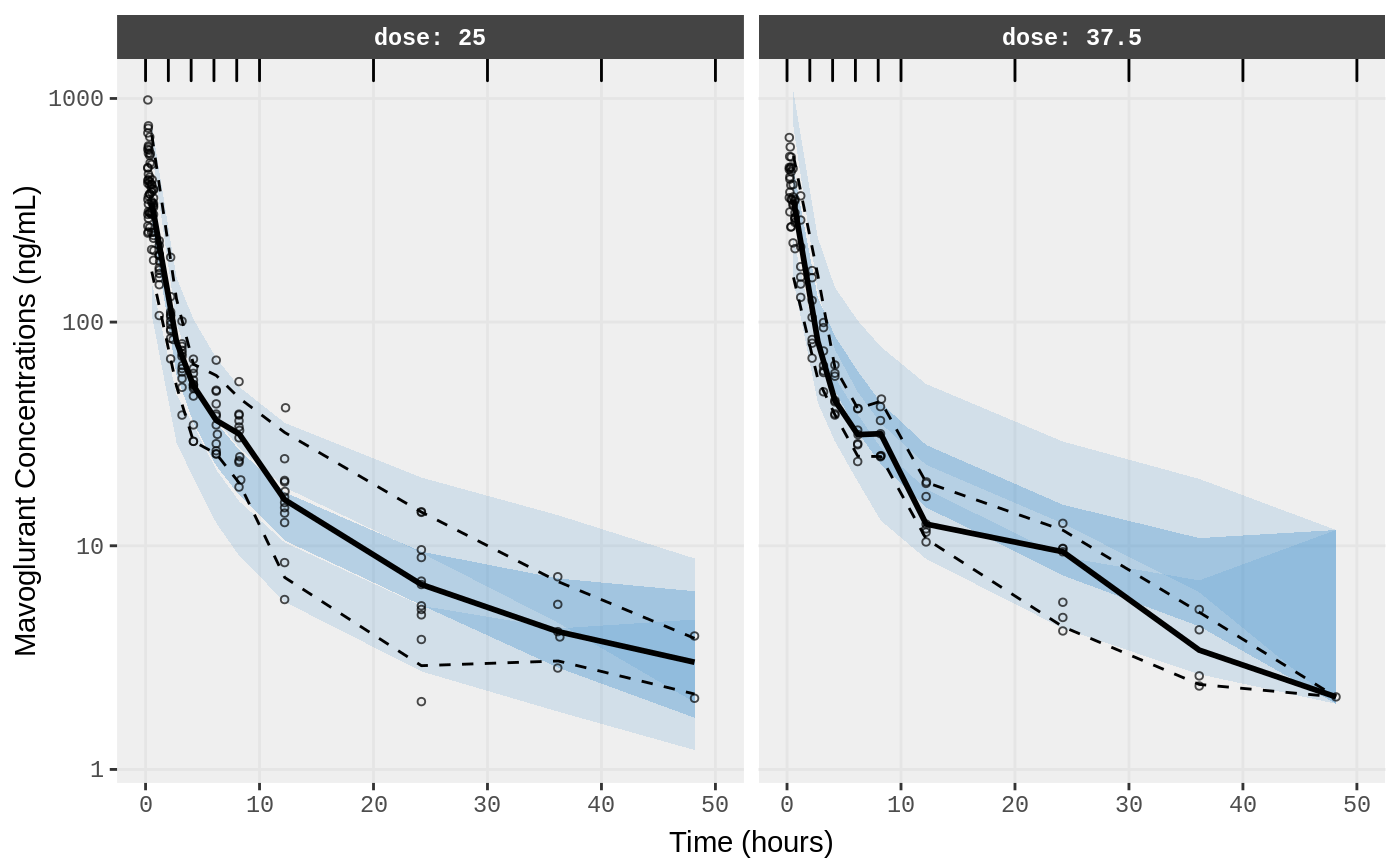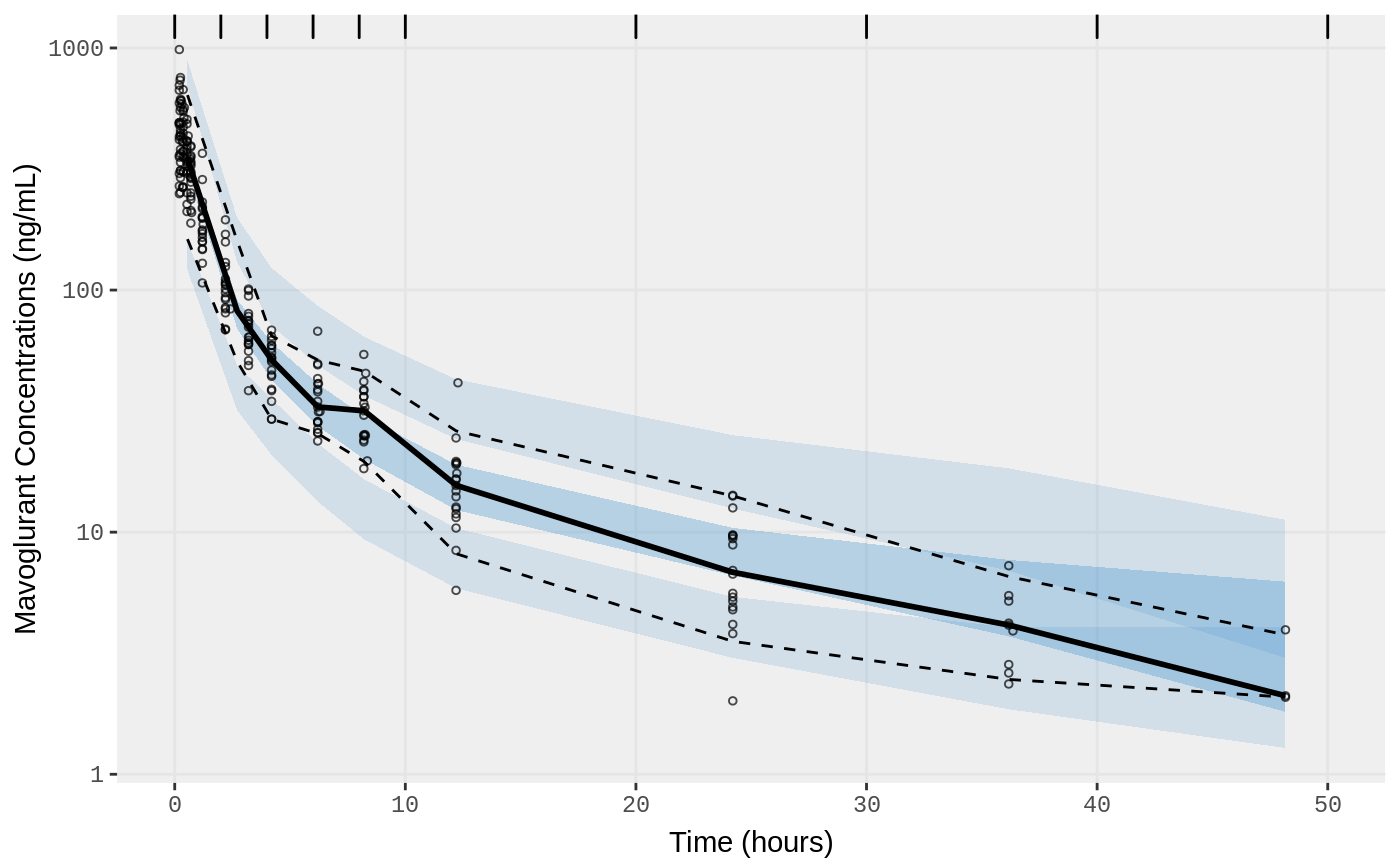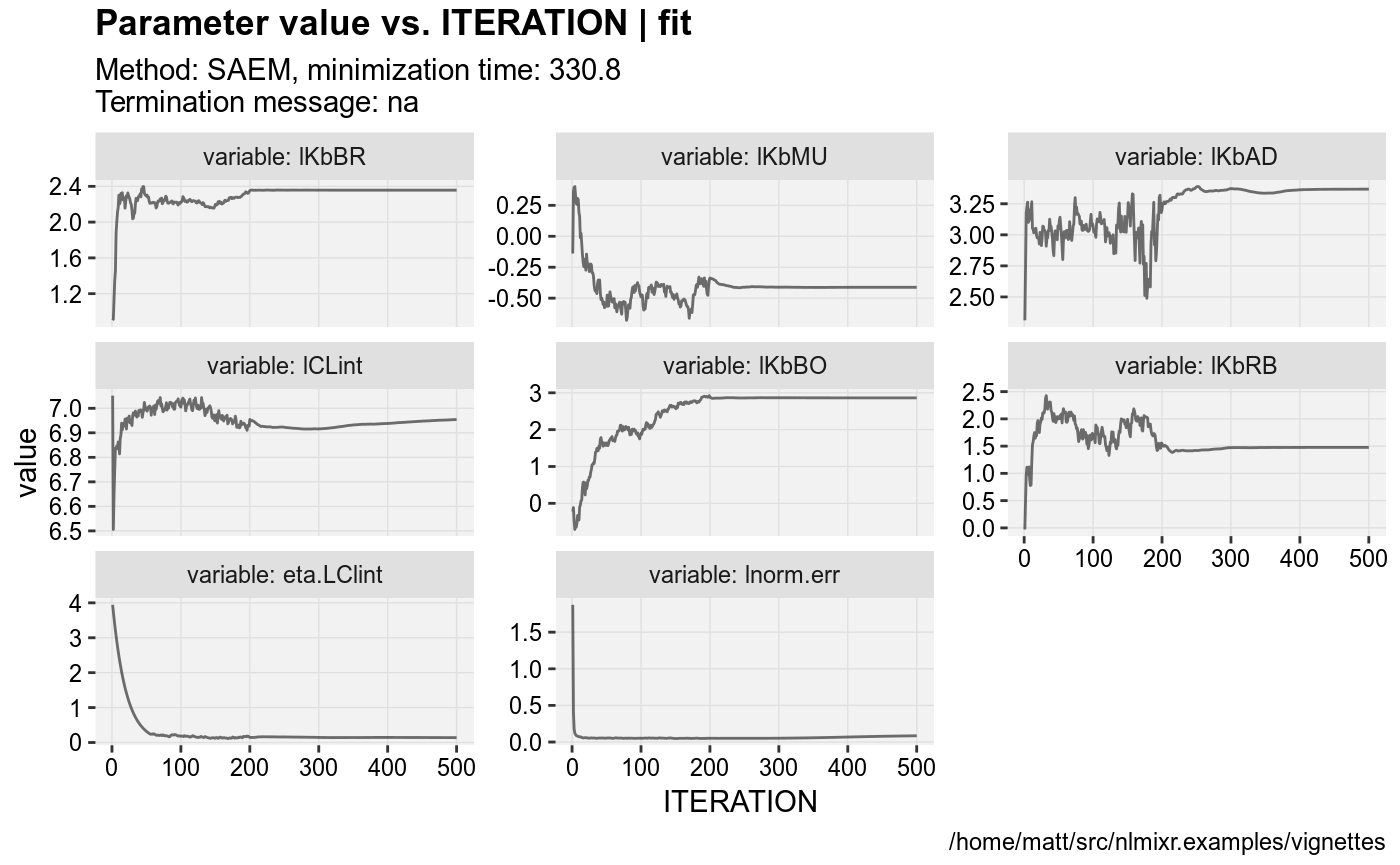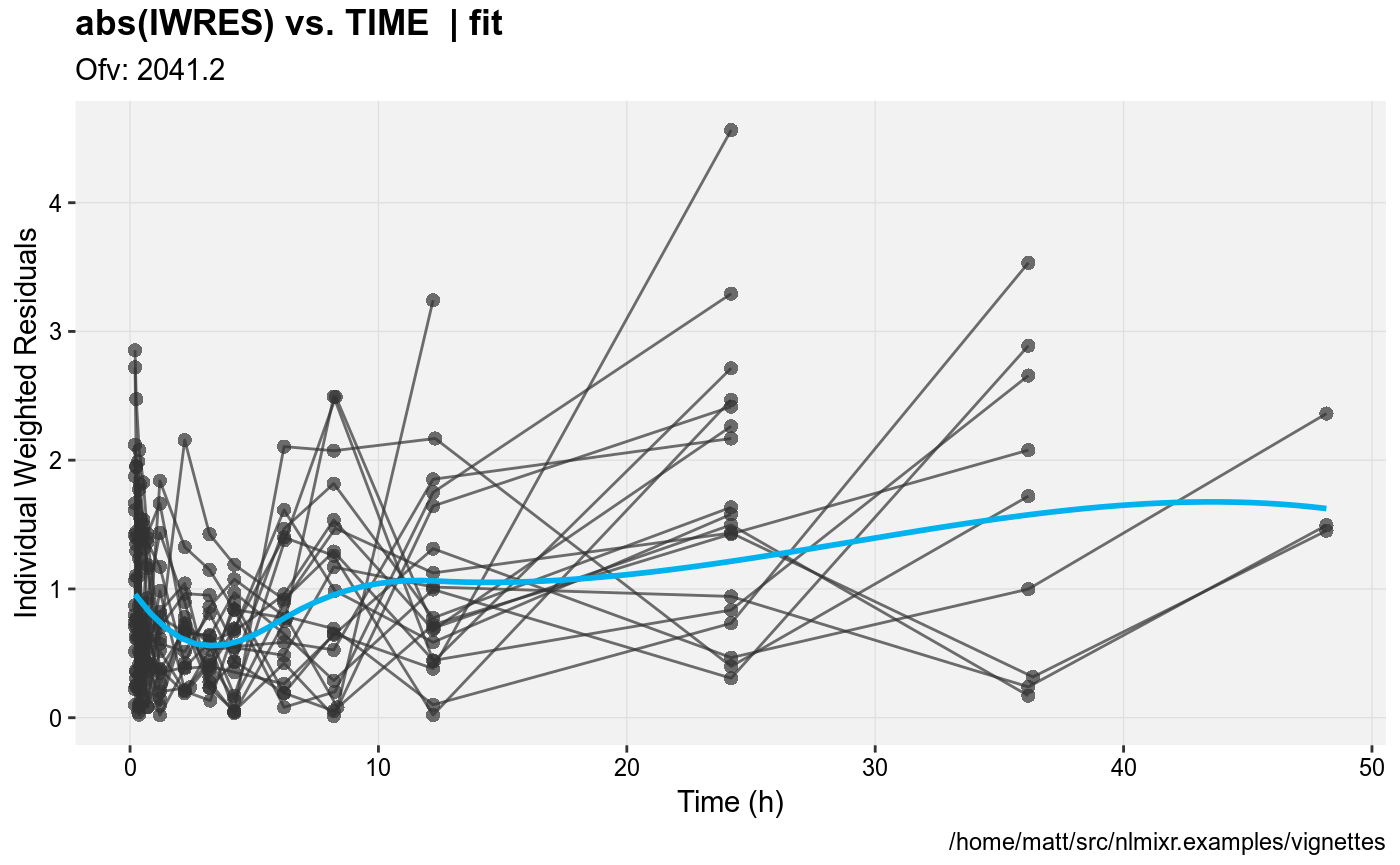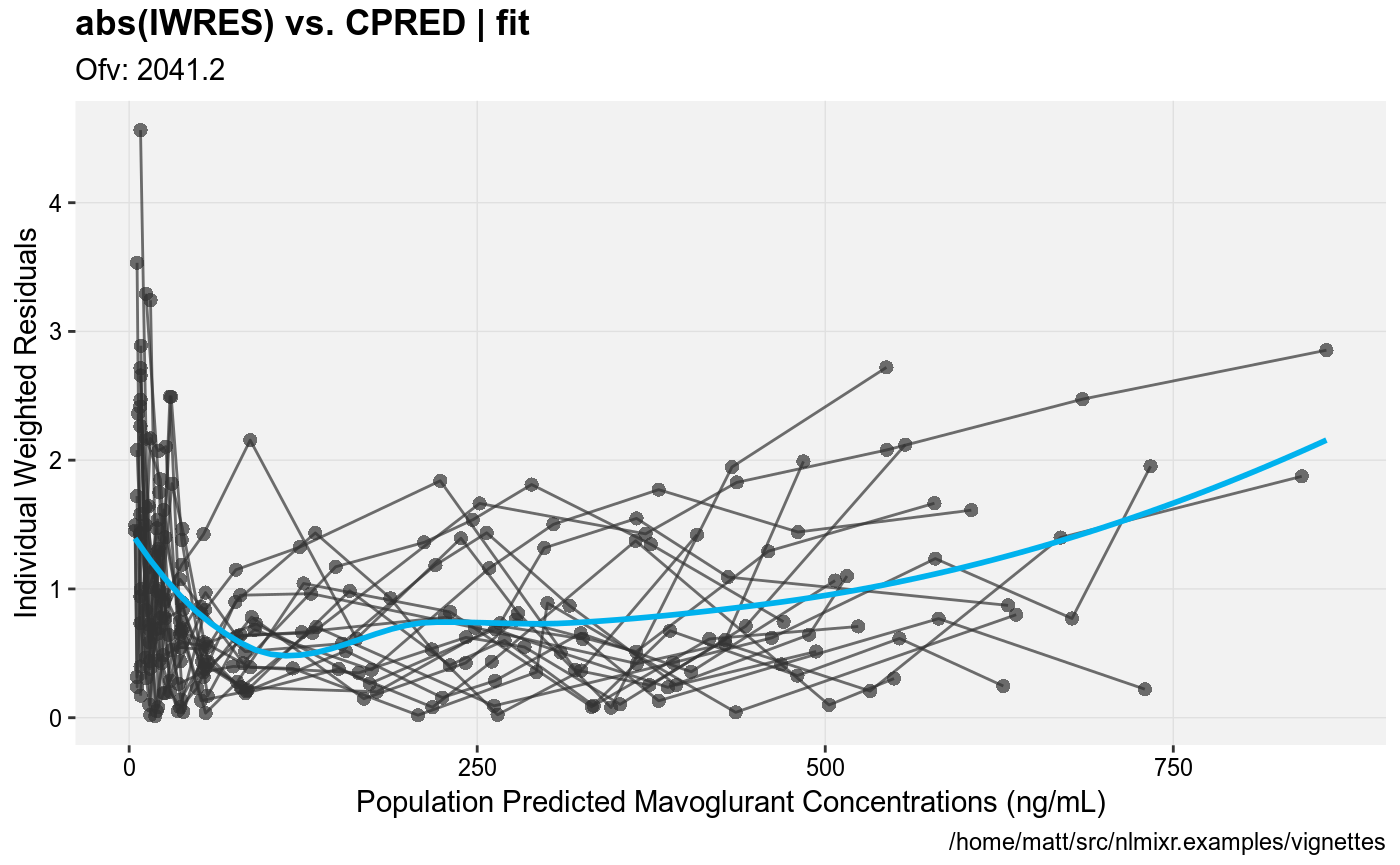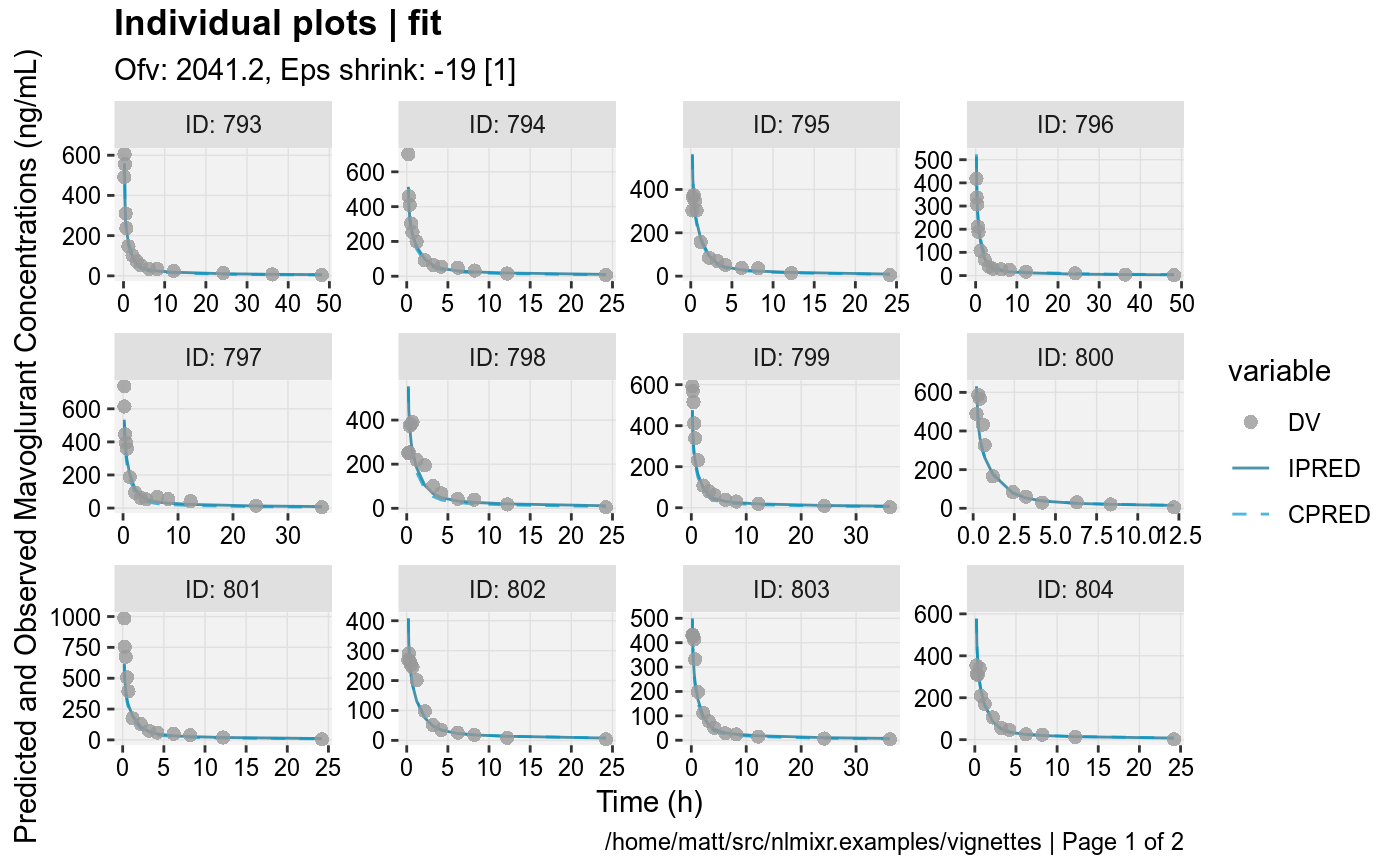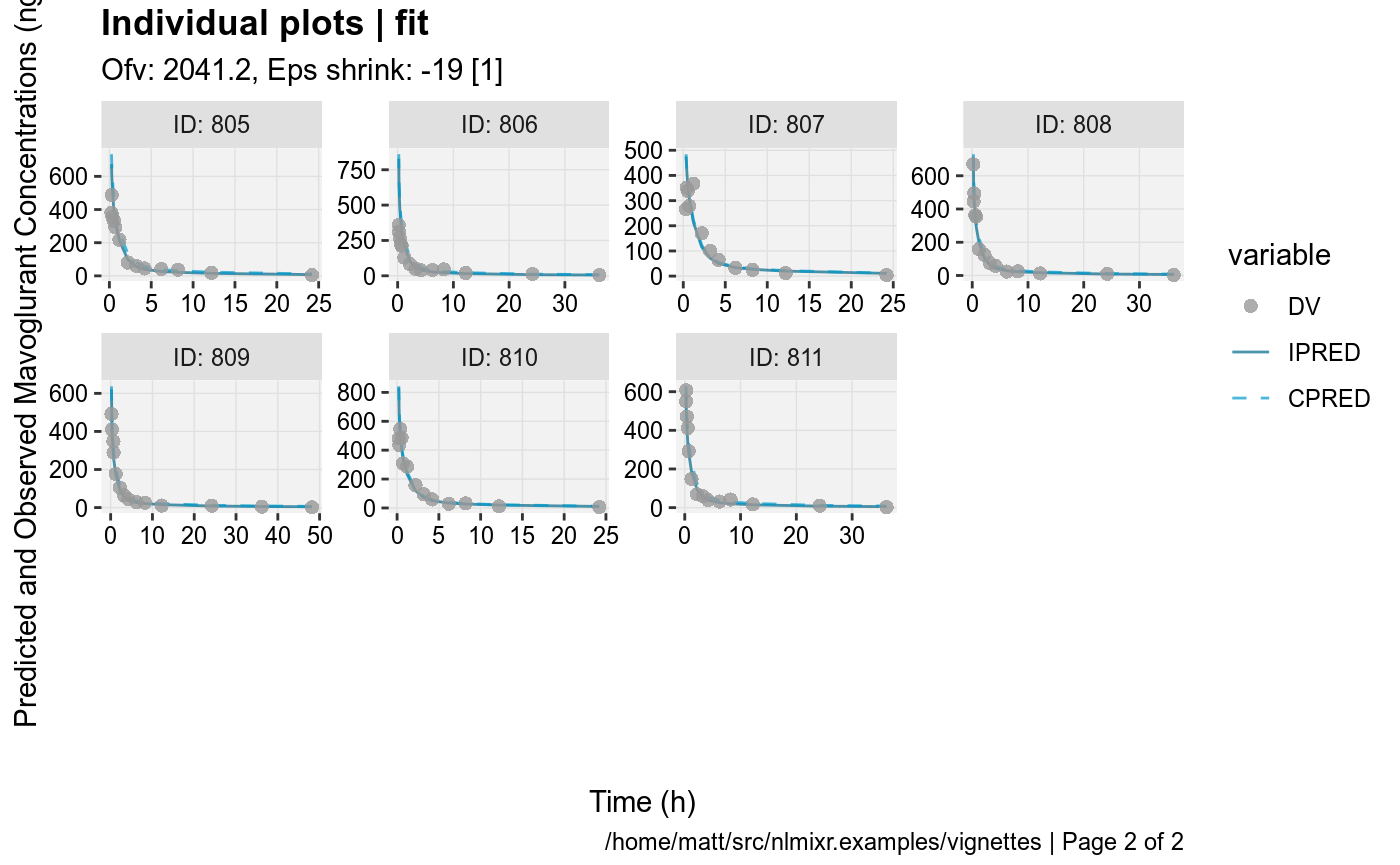#> Compiling VPC model...
#> done
#> done (4.83 sec)
#> Warning in data.frame(..., check.names = FALSE): row names were found from
#> a short variable and have been discarded
#> Compiling VPC model...
#> done
#> done (4.89 sec)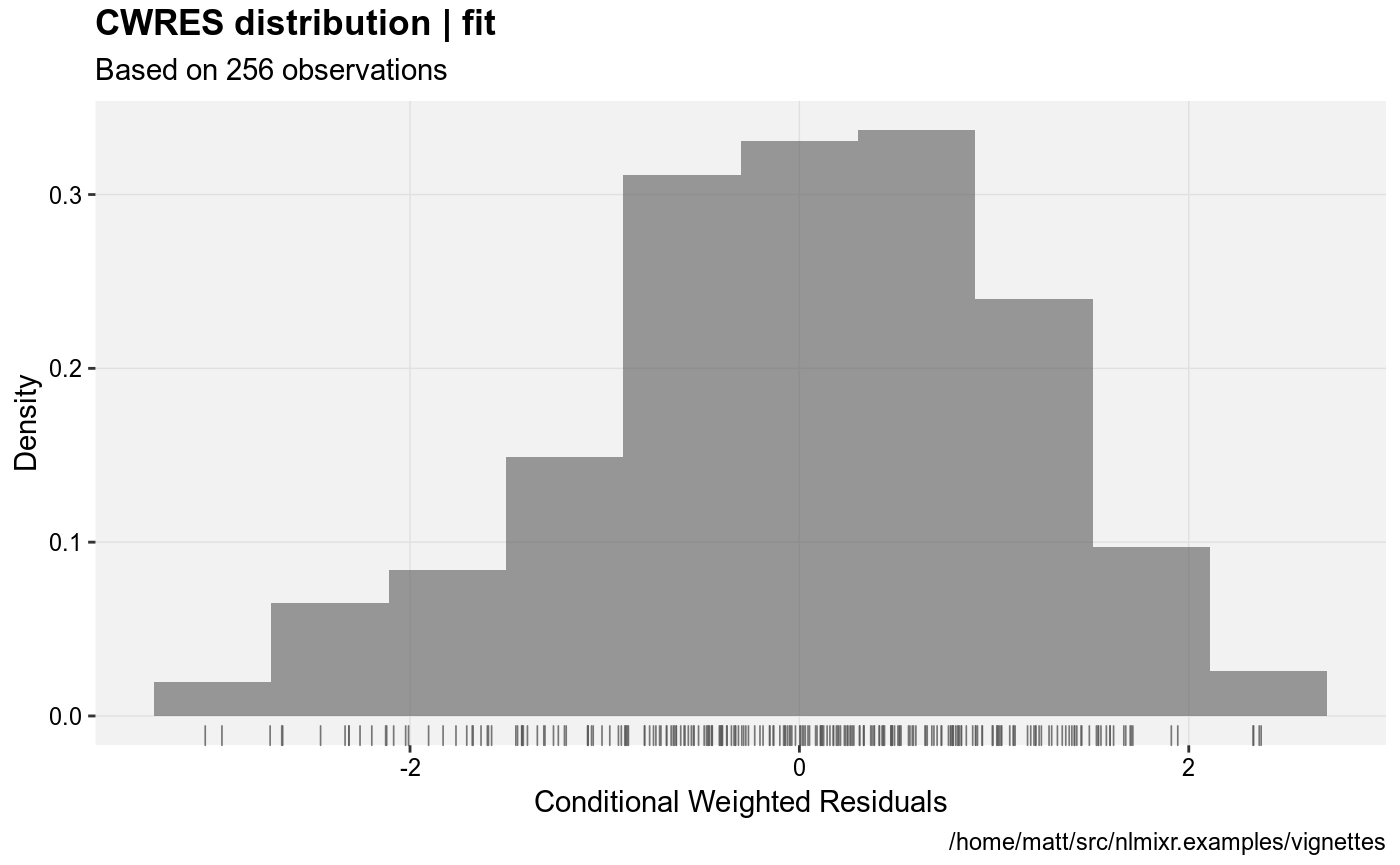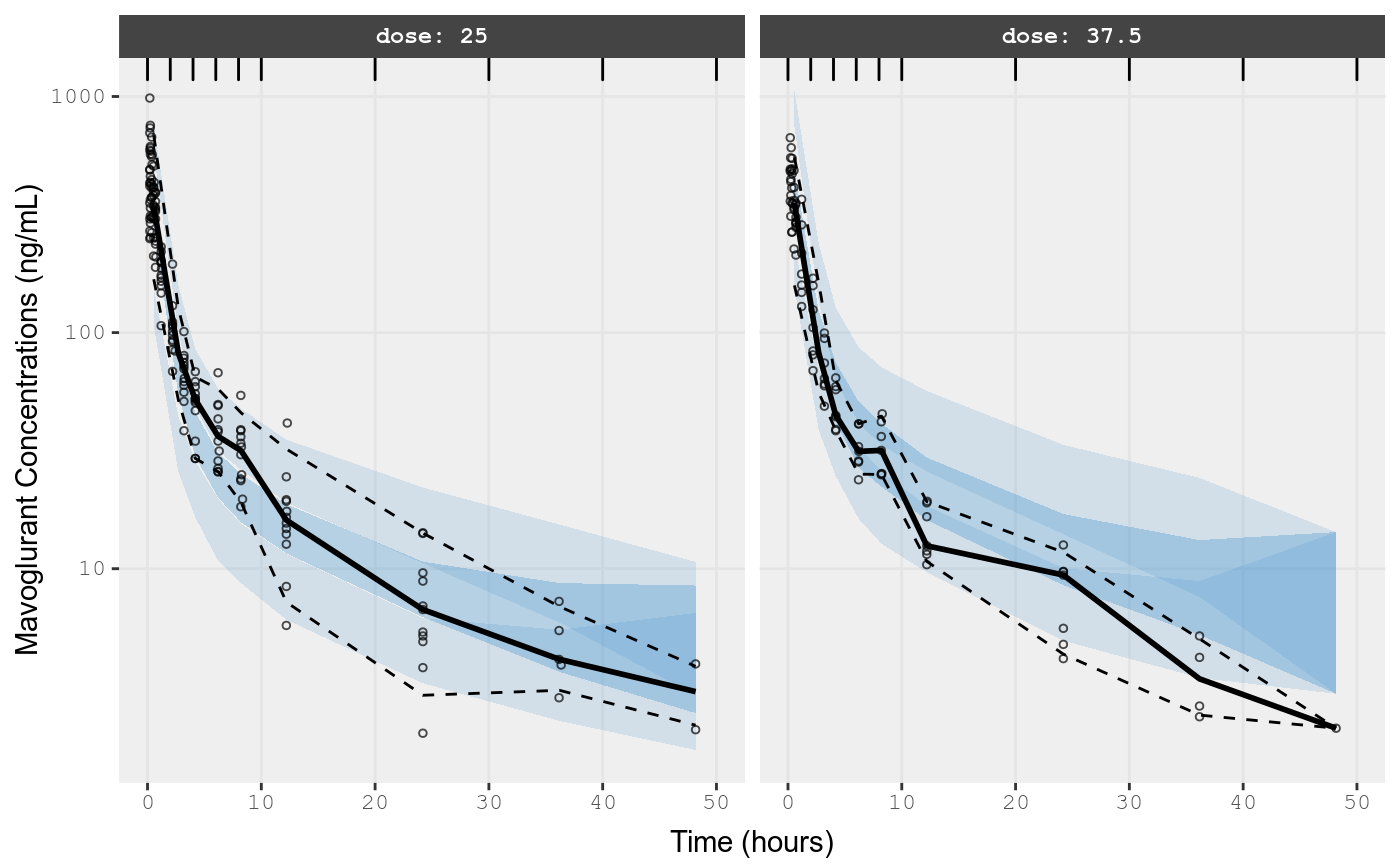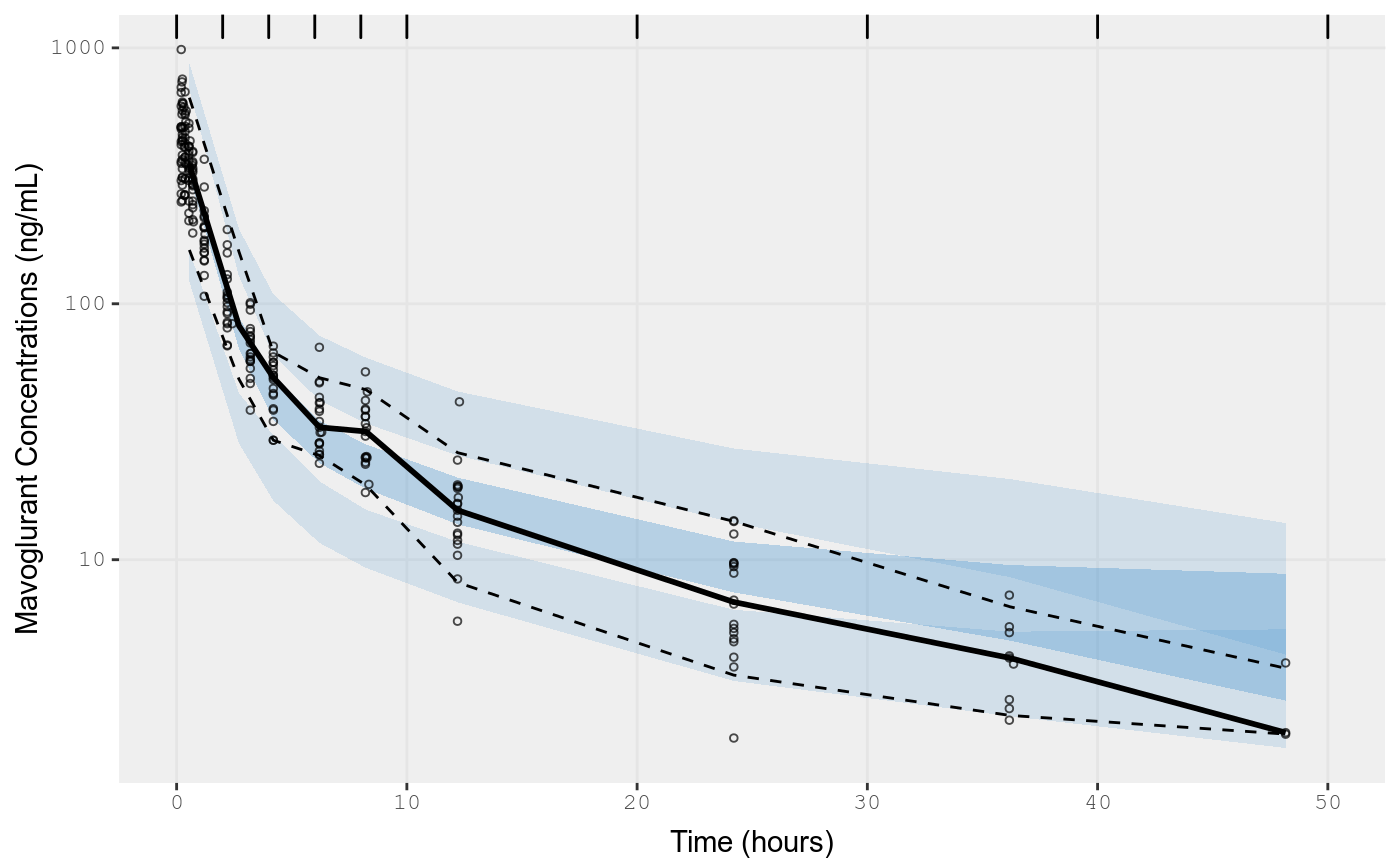# Piping to FOCEi

You can pipe models from different estimation methods to new estimation methods.

## Additive + Proportional

fit.addProp.F <- fit.addProp.S %>%
nlmixr(est="focei"); ## Since this was a model pipline, the data
#> Loading model already run (/home/matt/R/x86_64-pc-linux-gnu-library/3.5/nlmixr.examples/nlmixr-pbpk-dat-focei-2360797054932d150b0bc8507a2c4685.rds)
## remains the same as the last fit.
gofs(fit.addProp.F);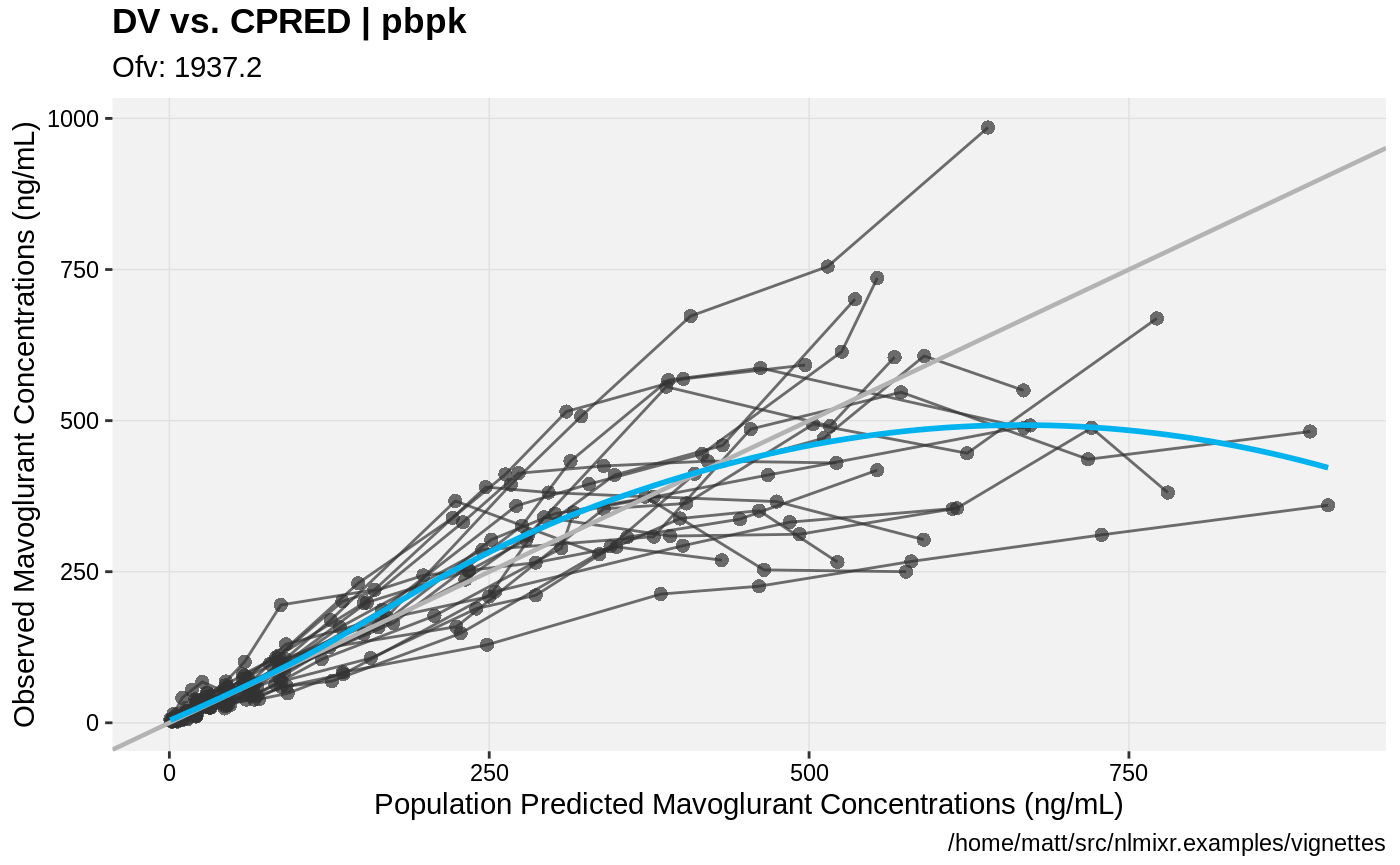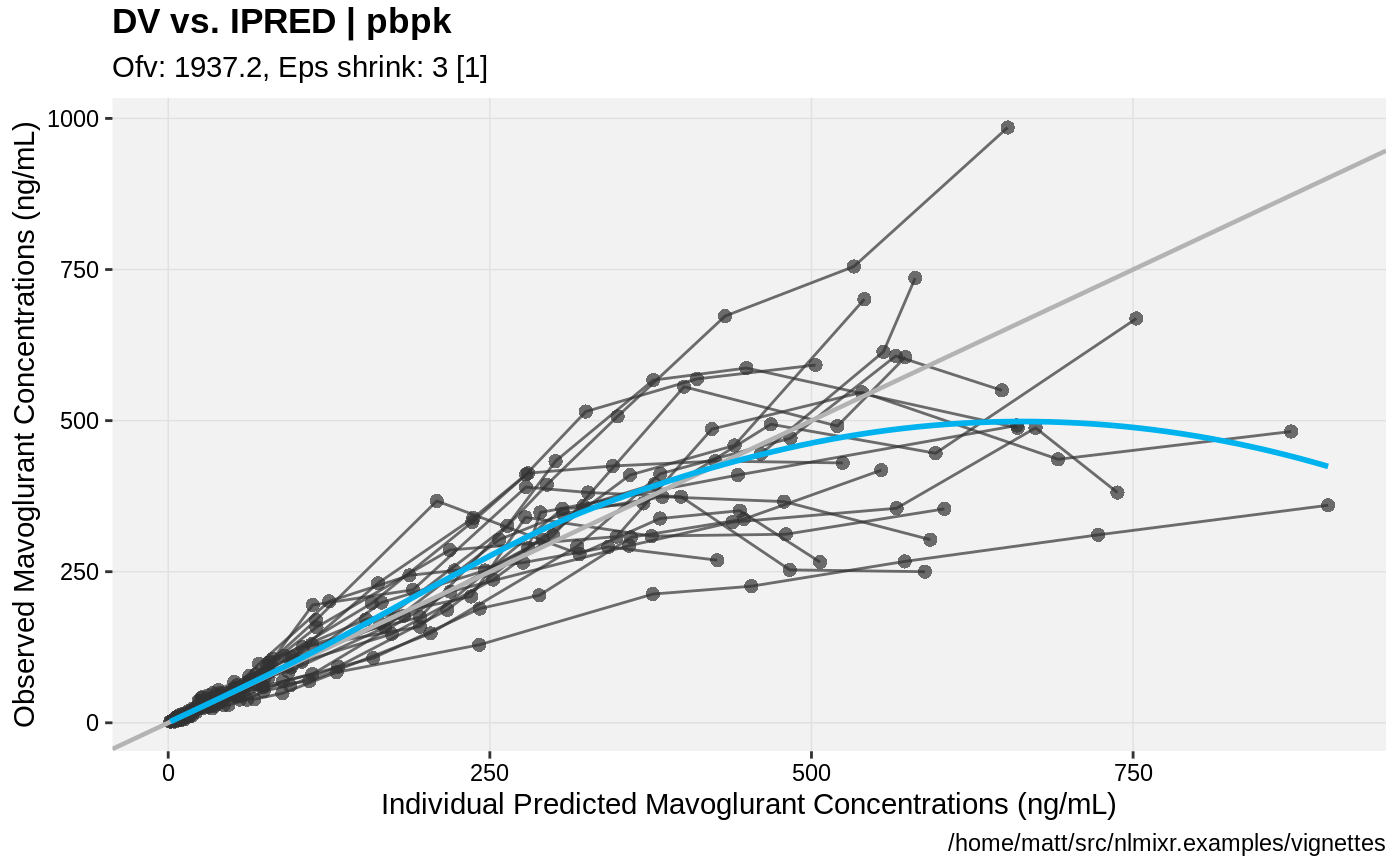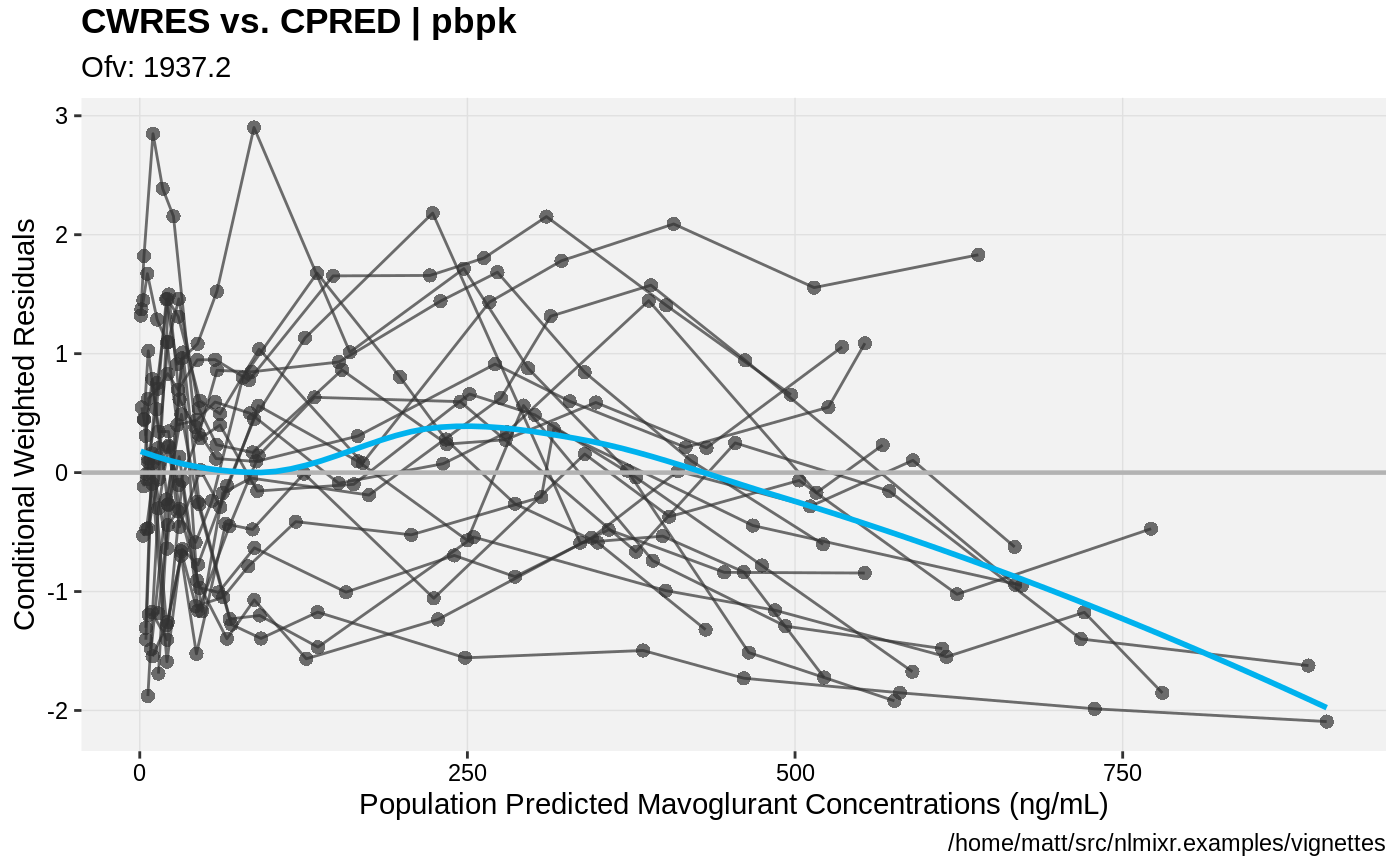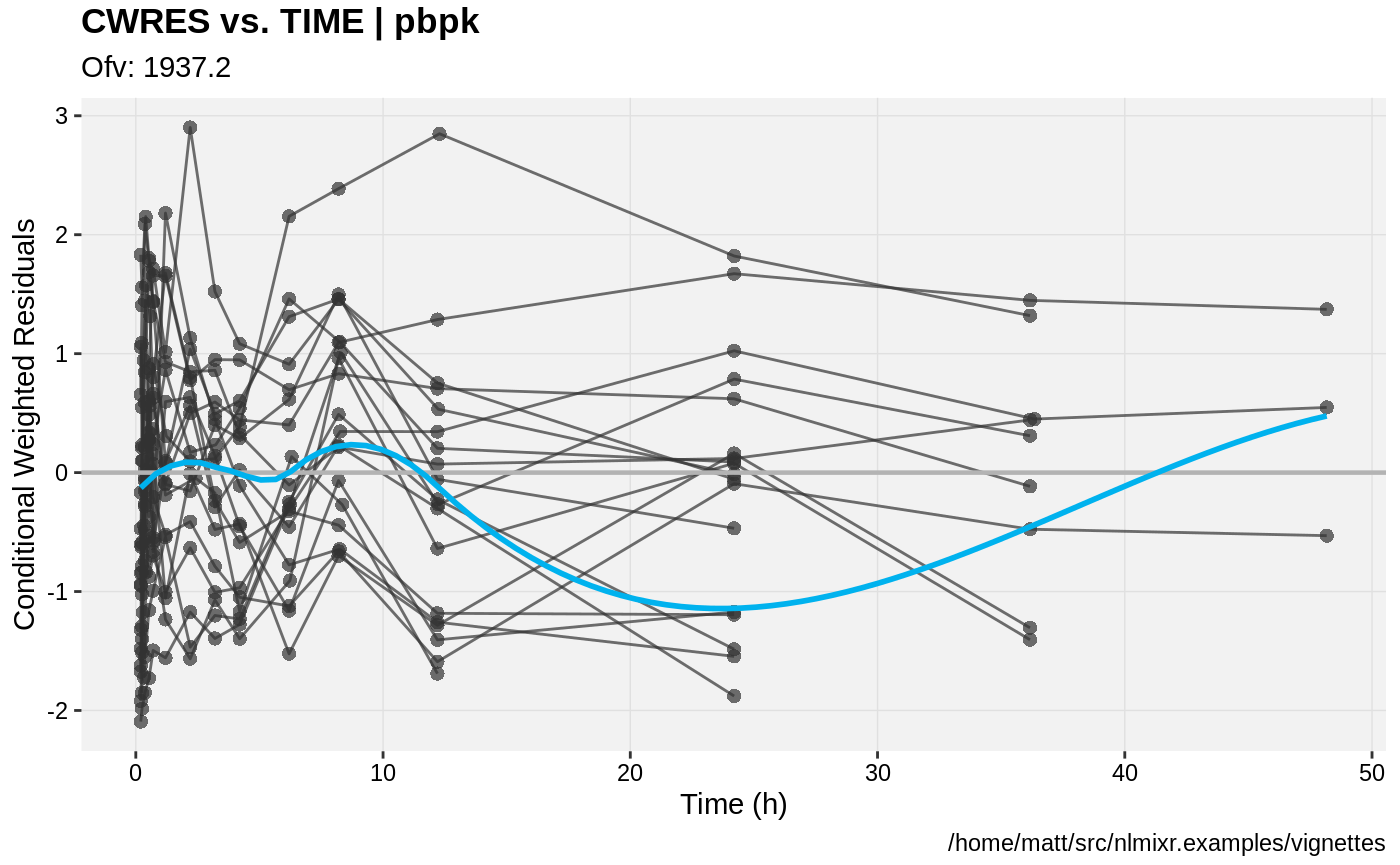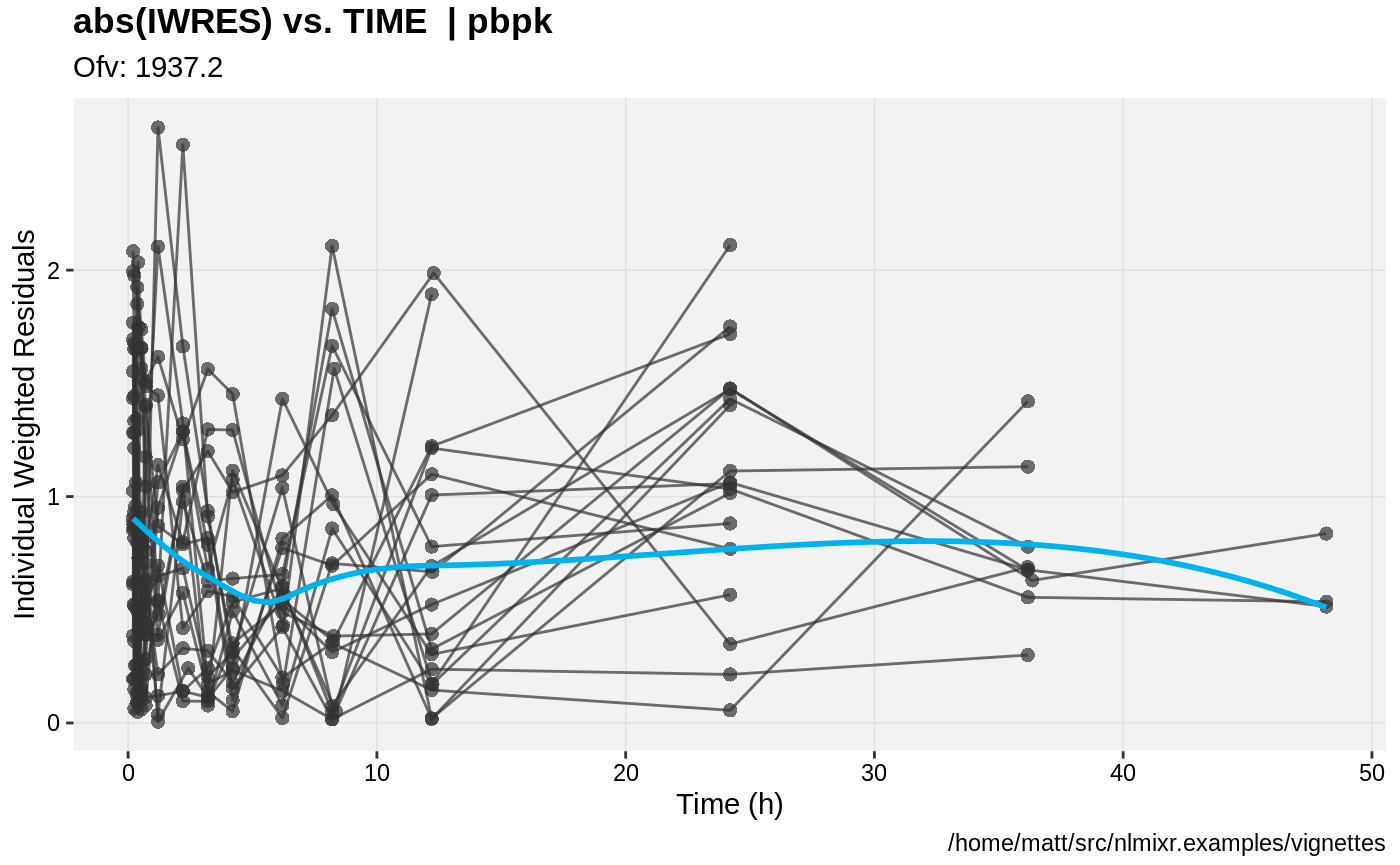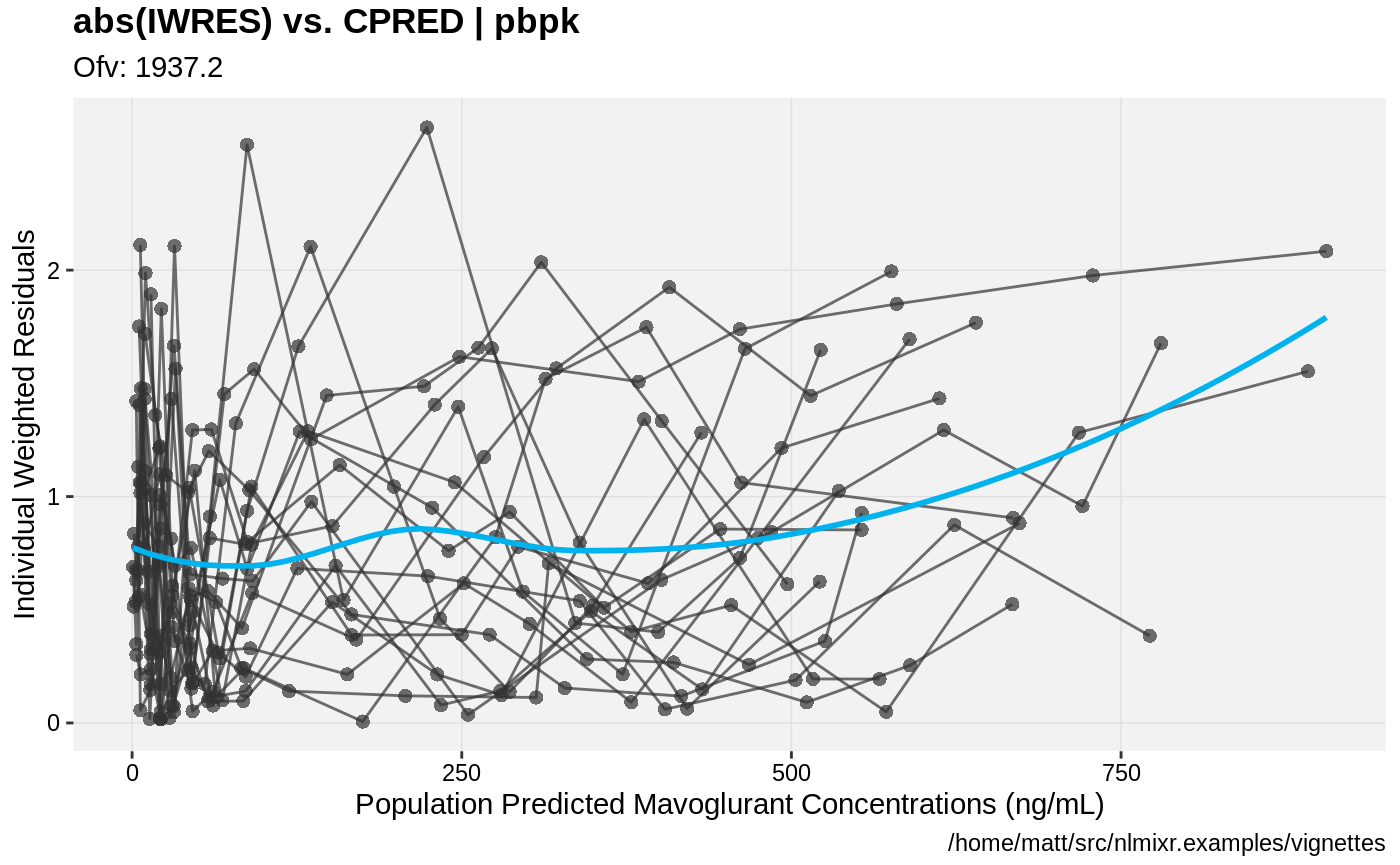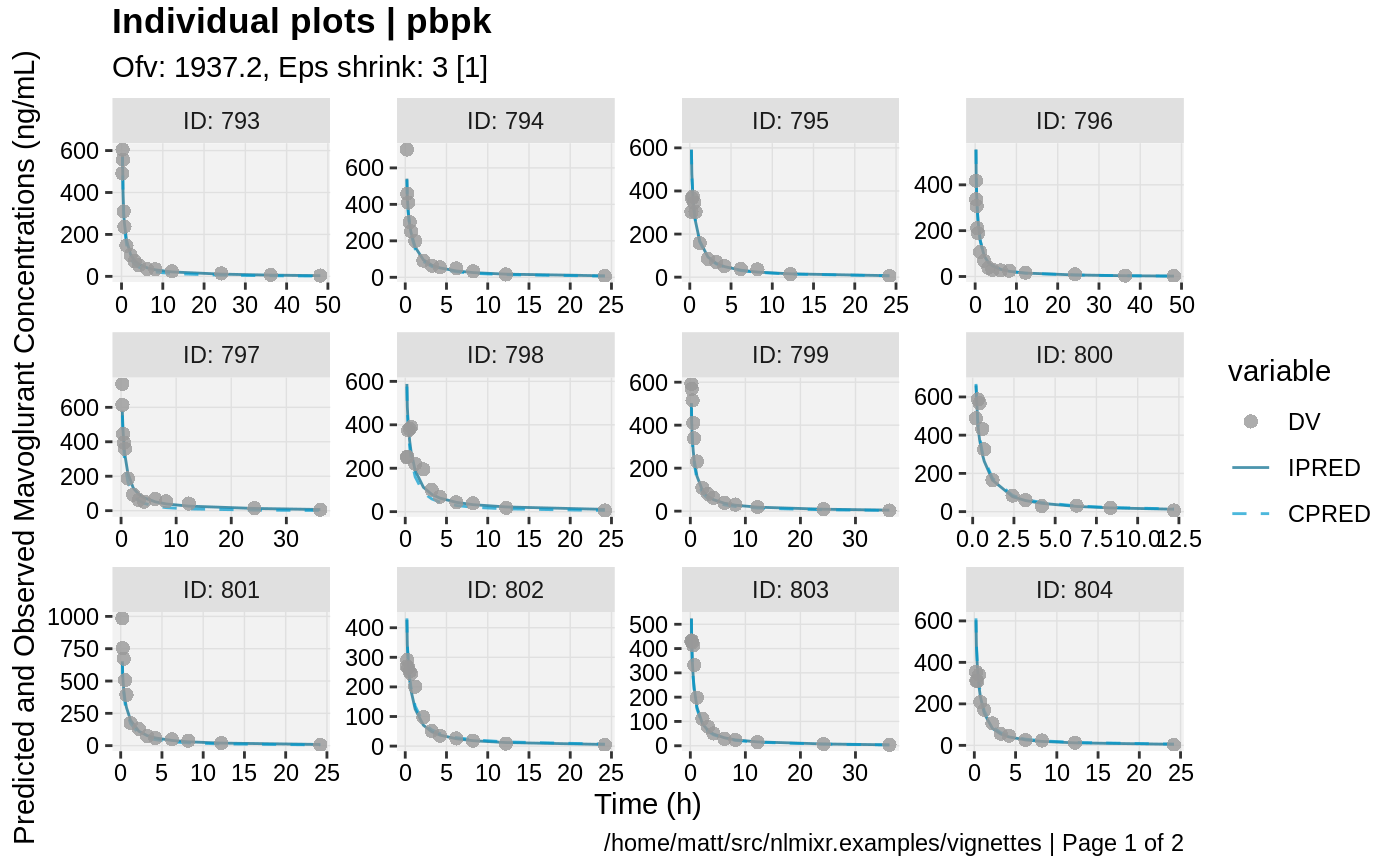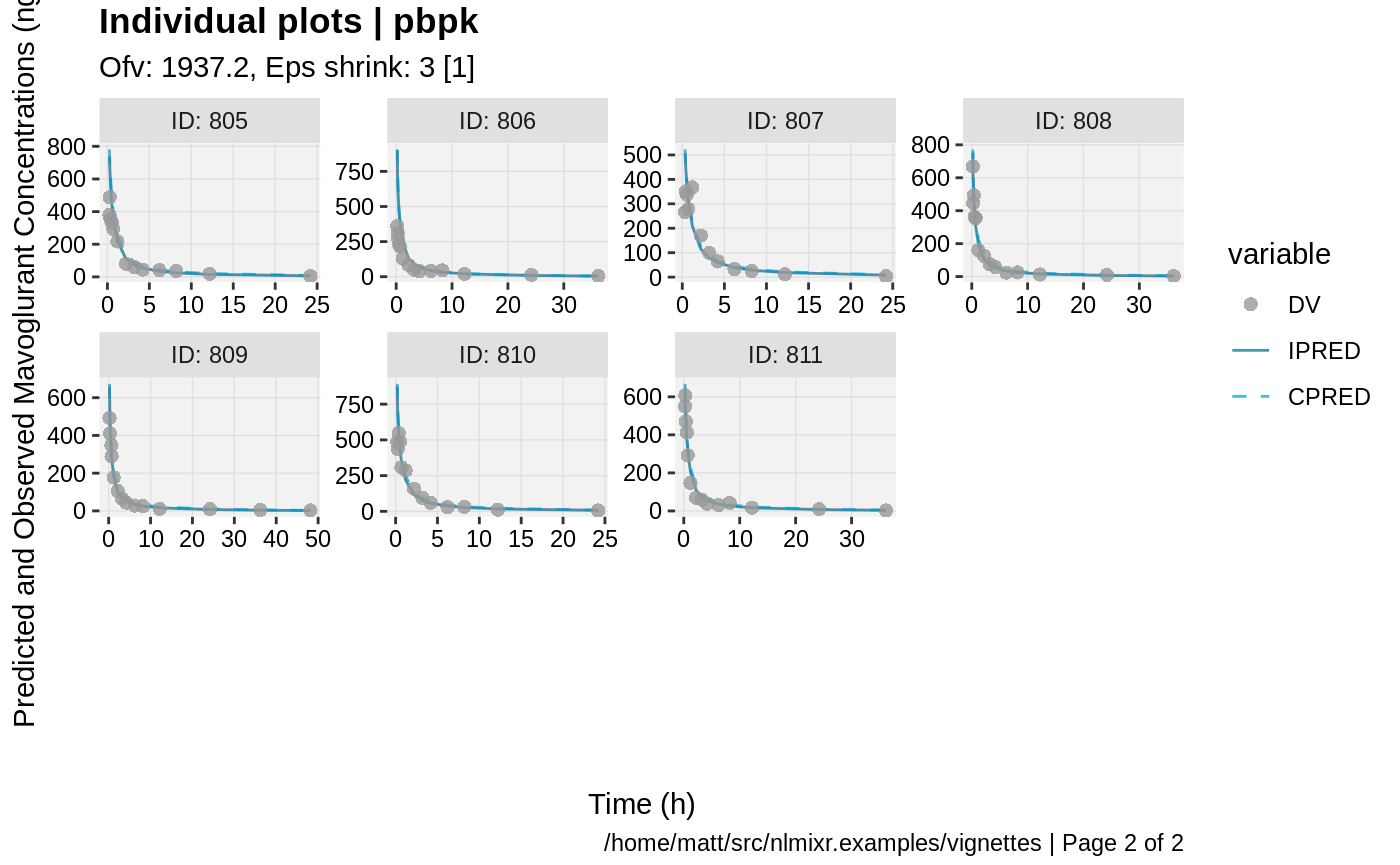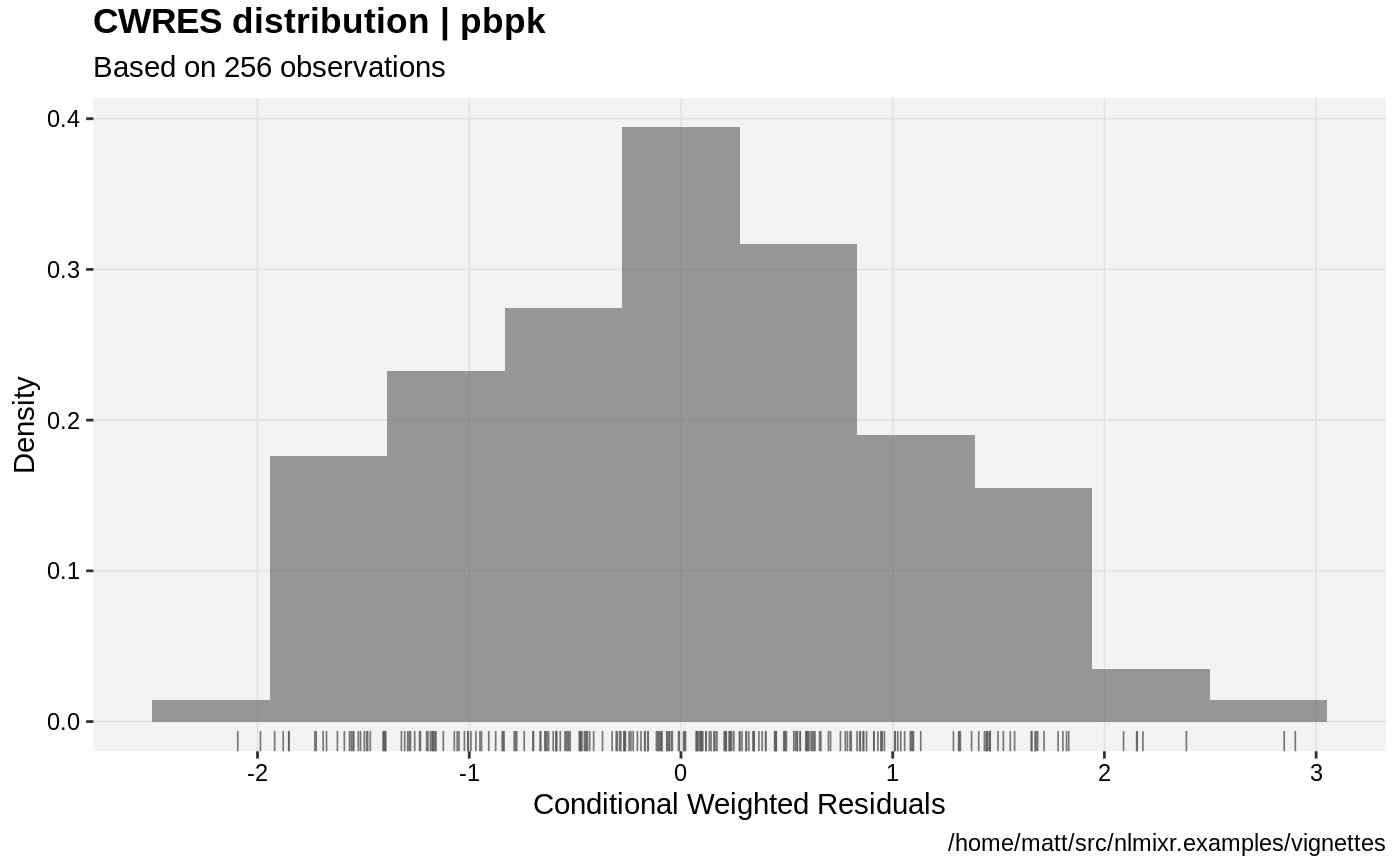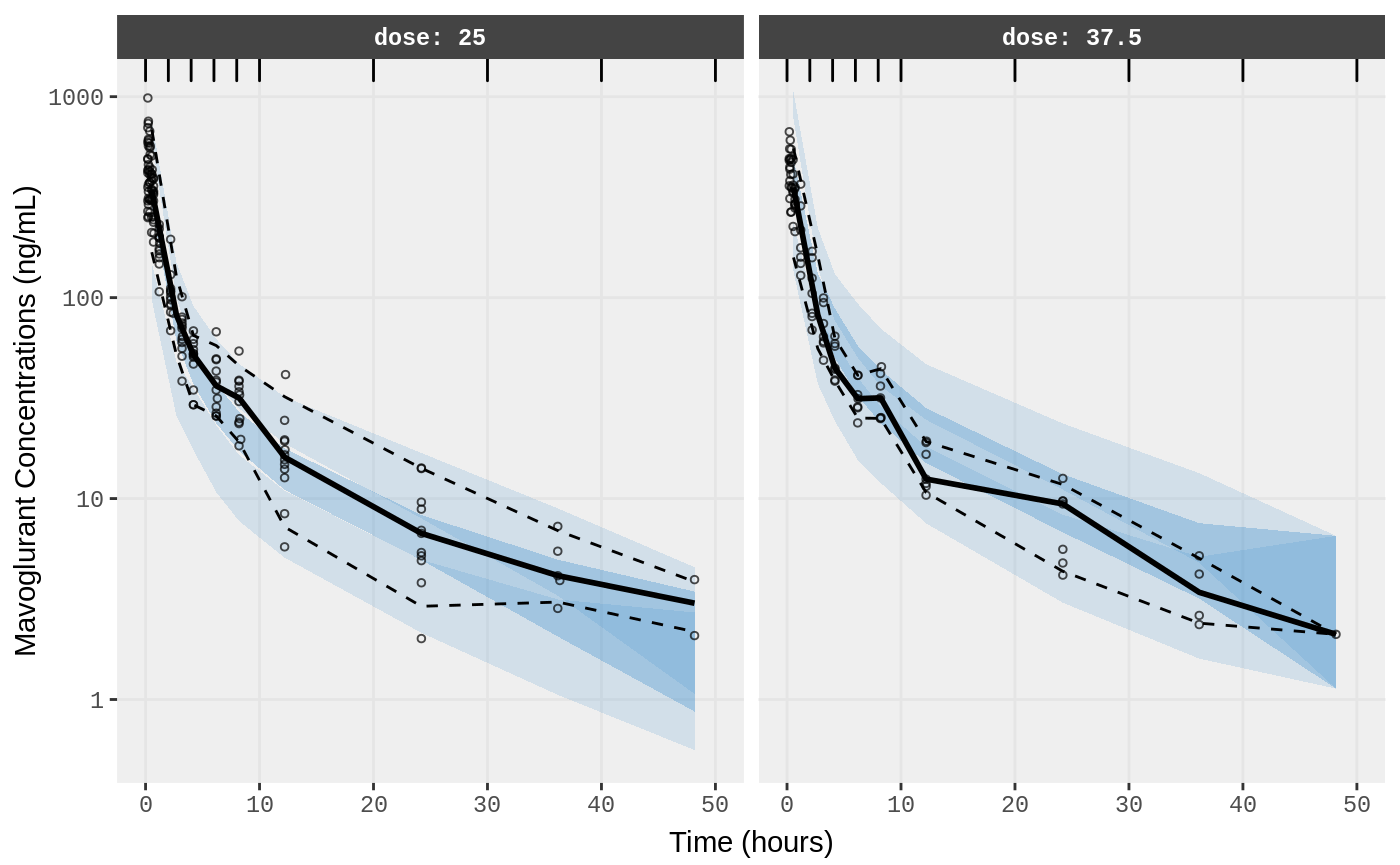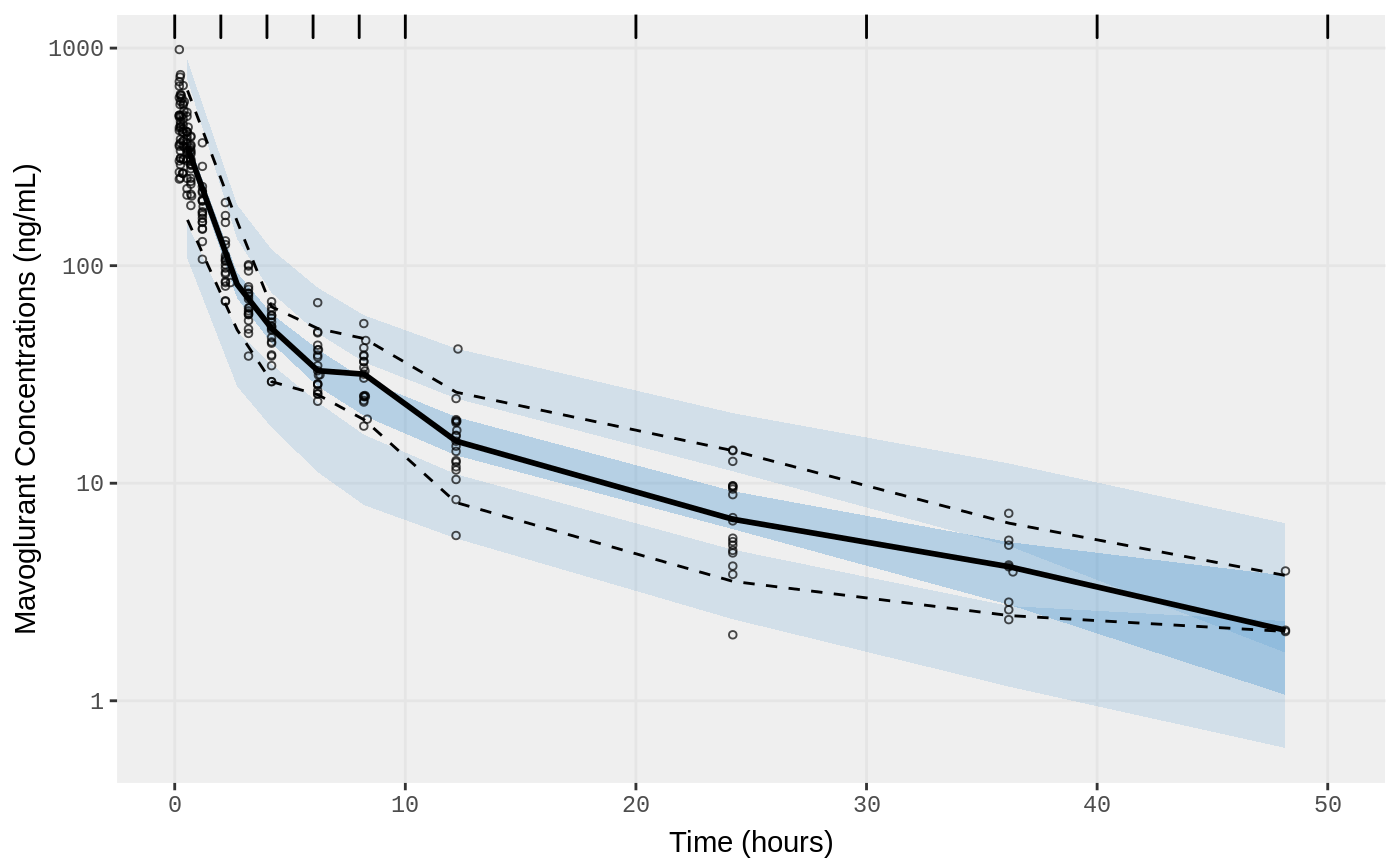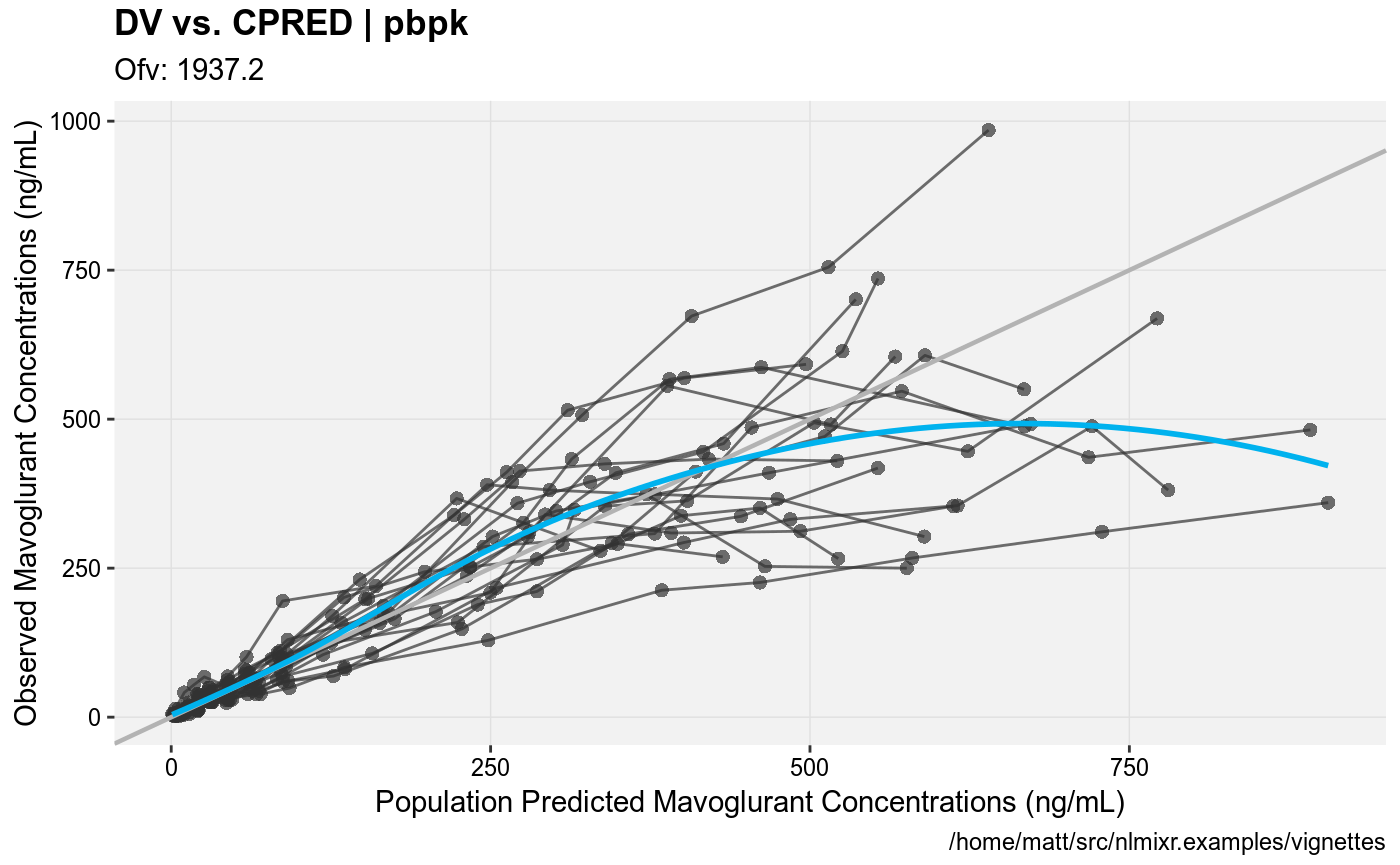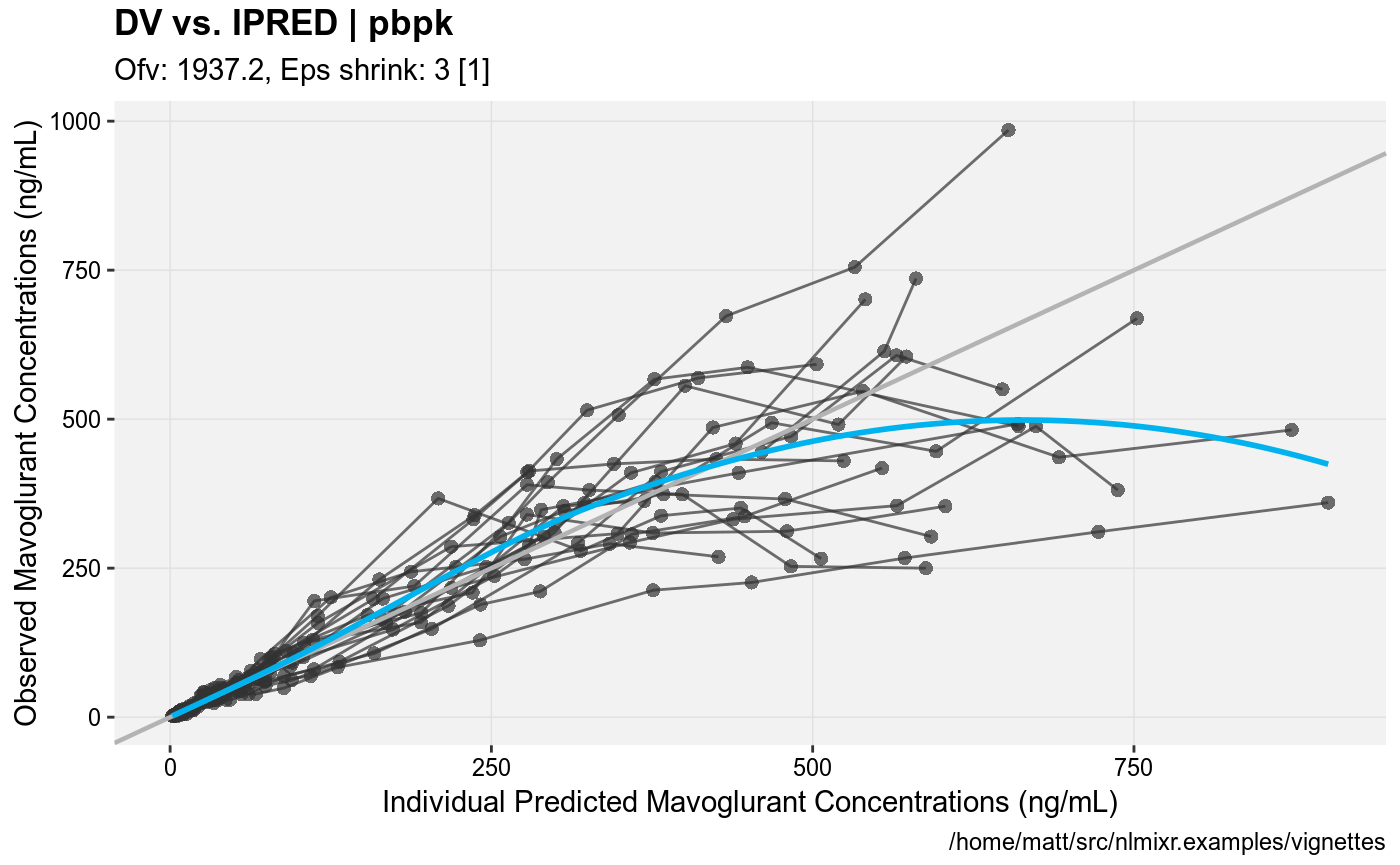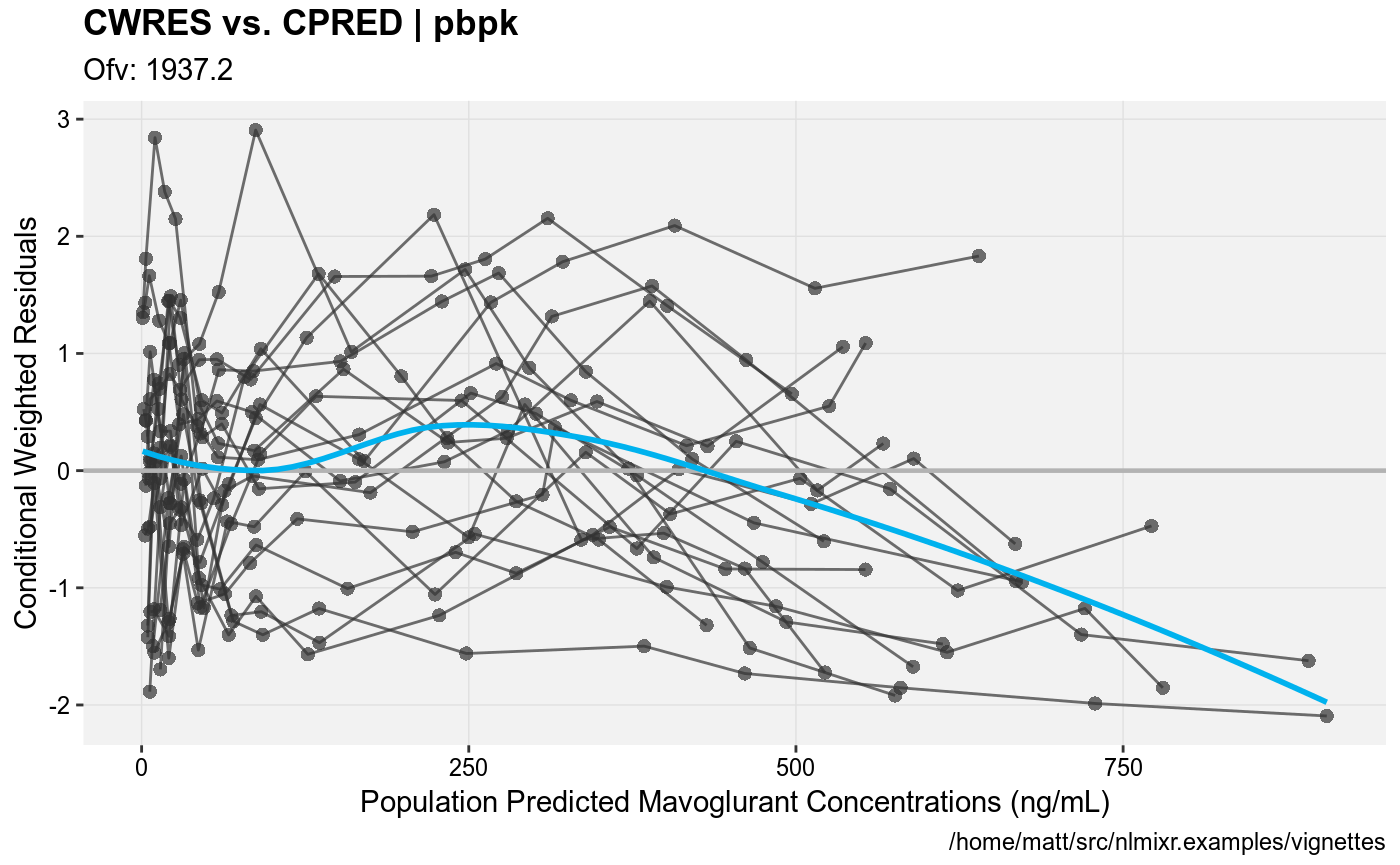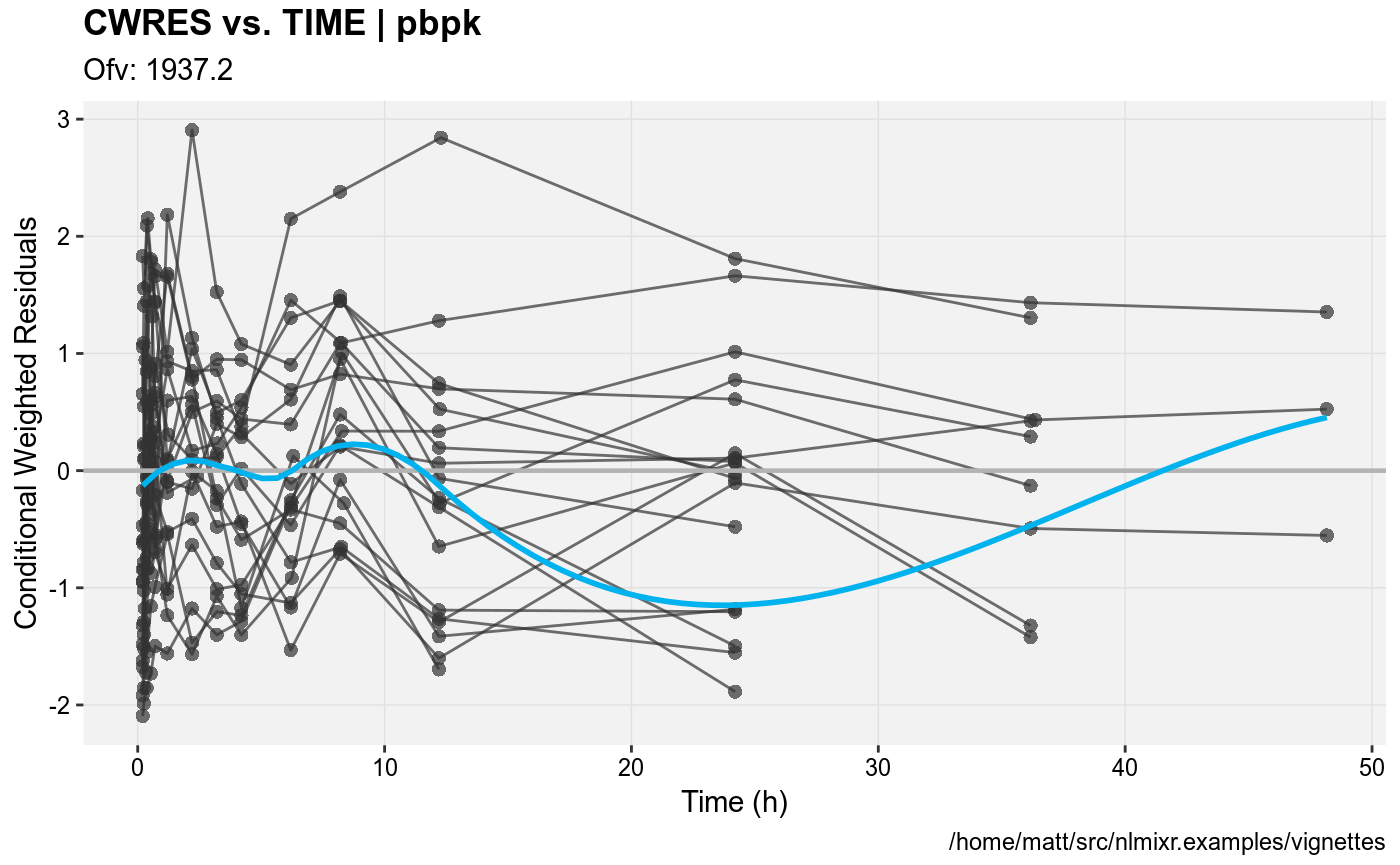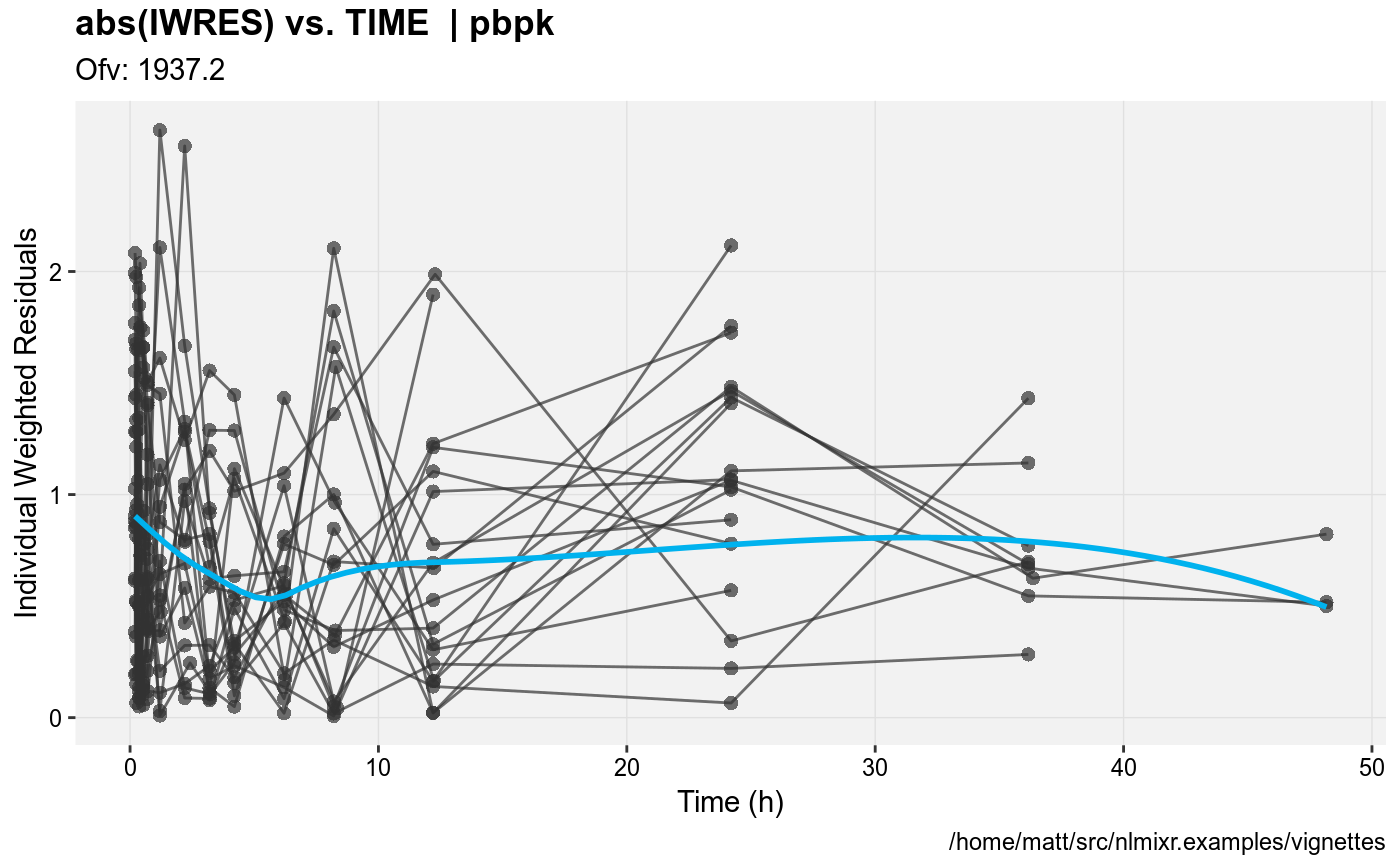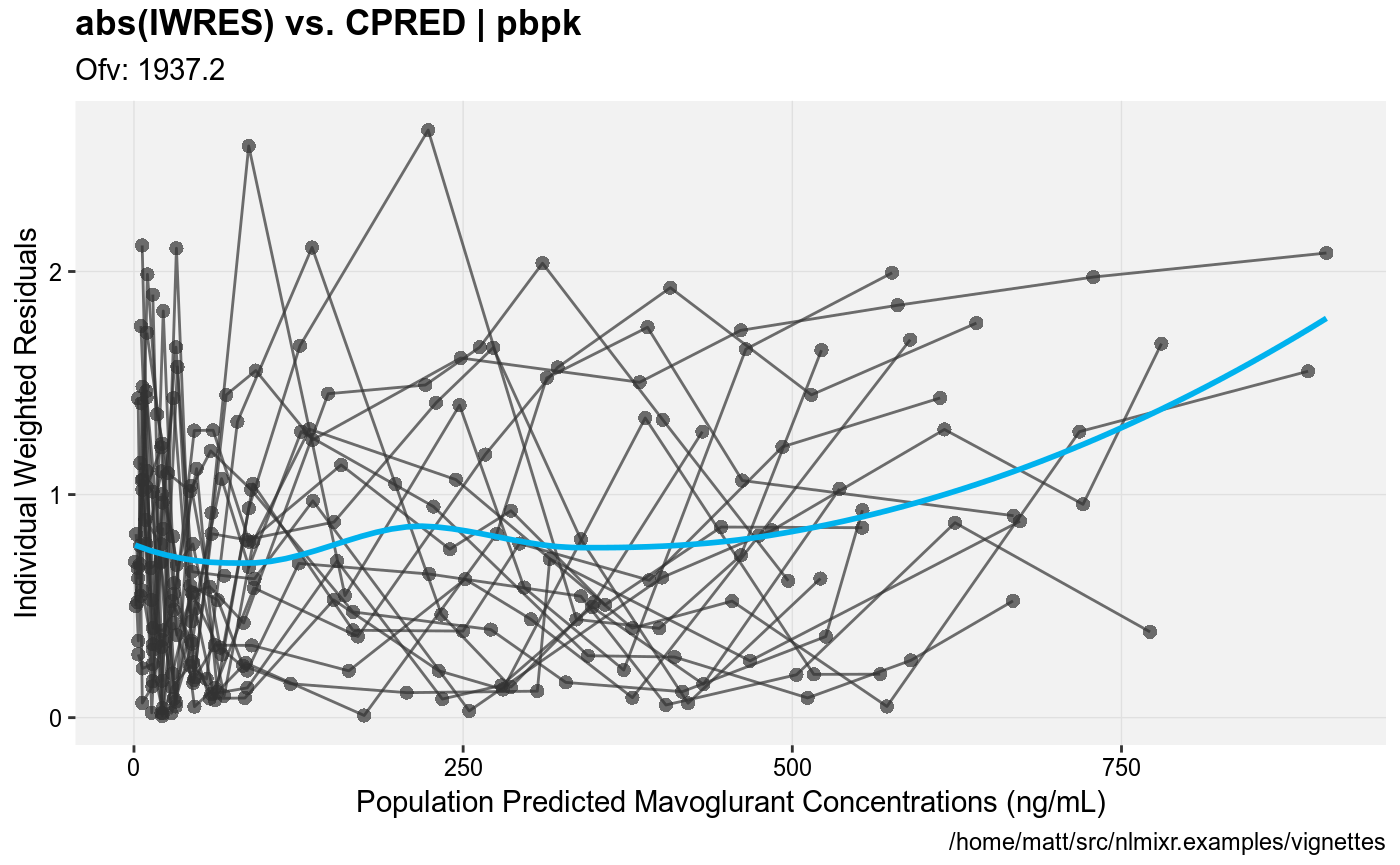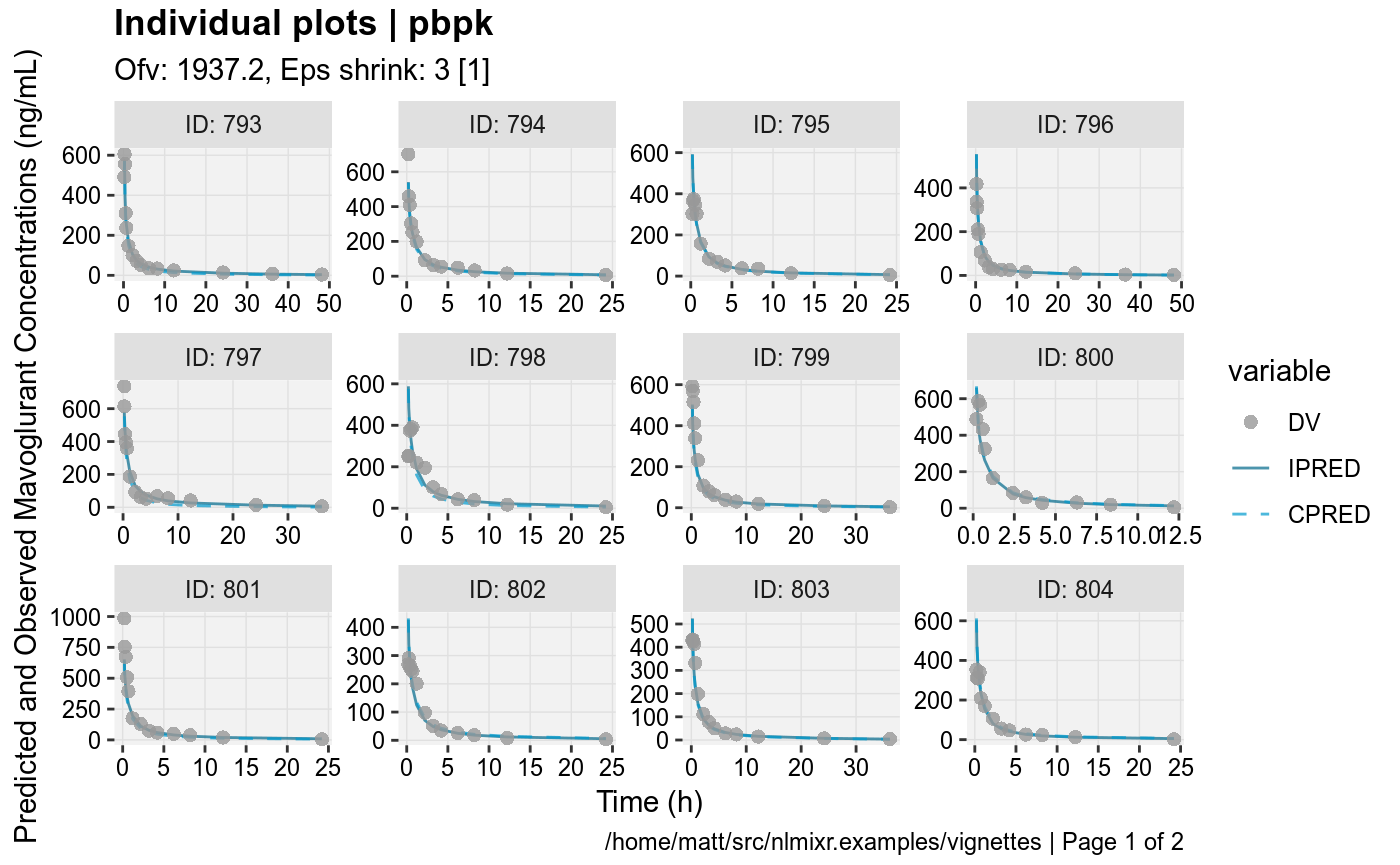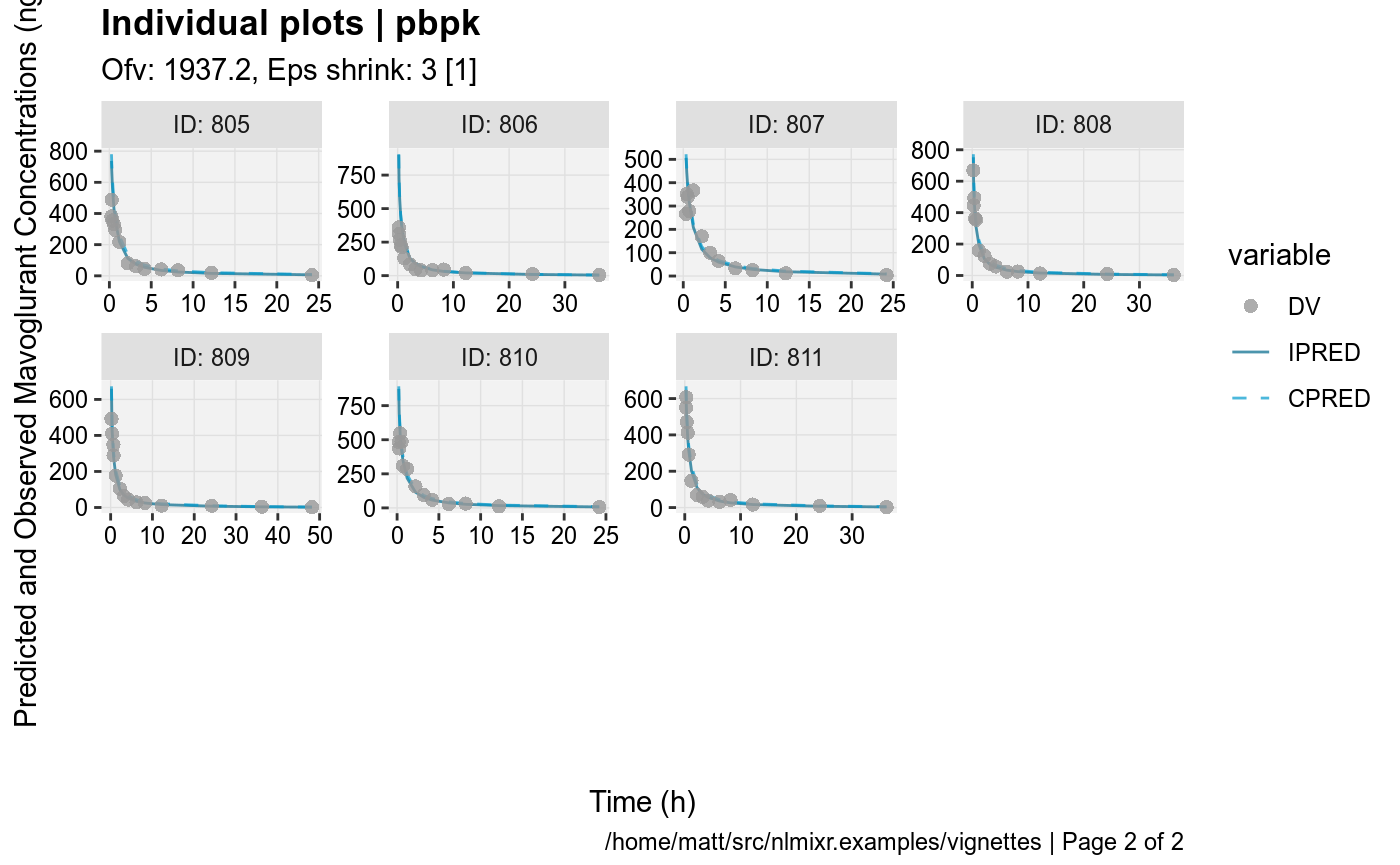#> Compiling VPC model...
#> done
#> done (4.19 sec)
#> Warning in data.frame(..., check.names = FALSE): row names were found from
#> a short variable and have been discarded
#> Compiling VPC model...
#> done
#> done (4.13 sec)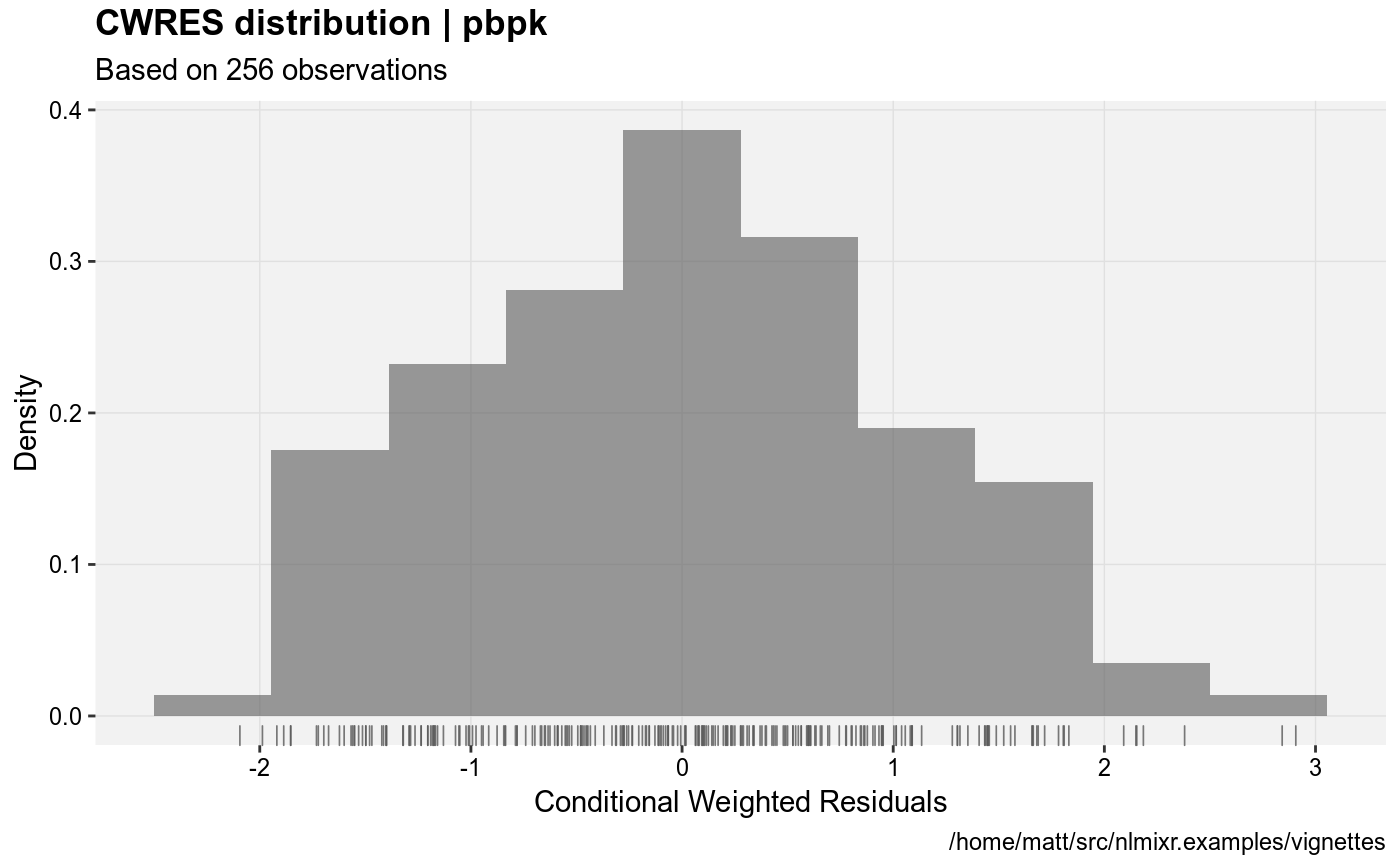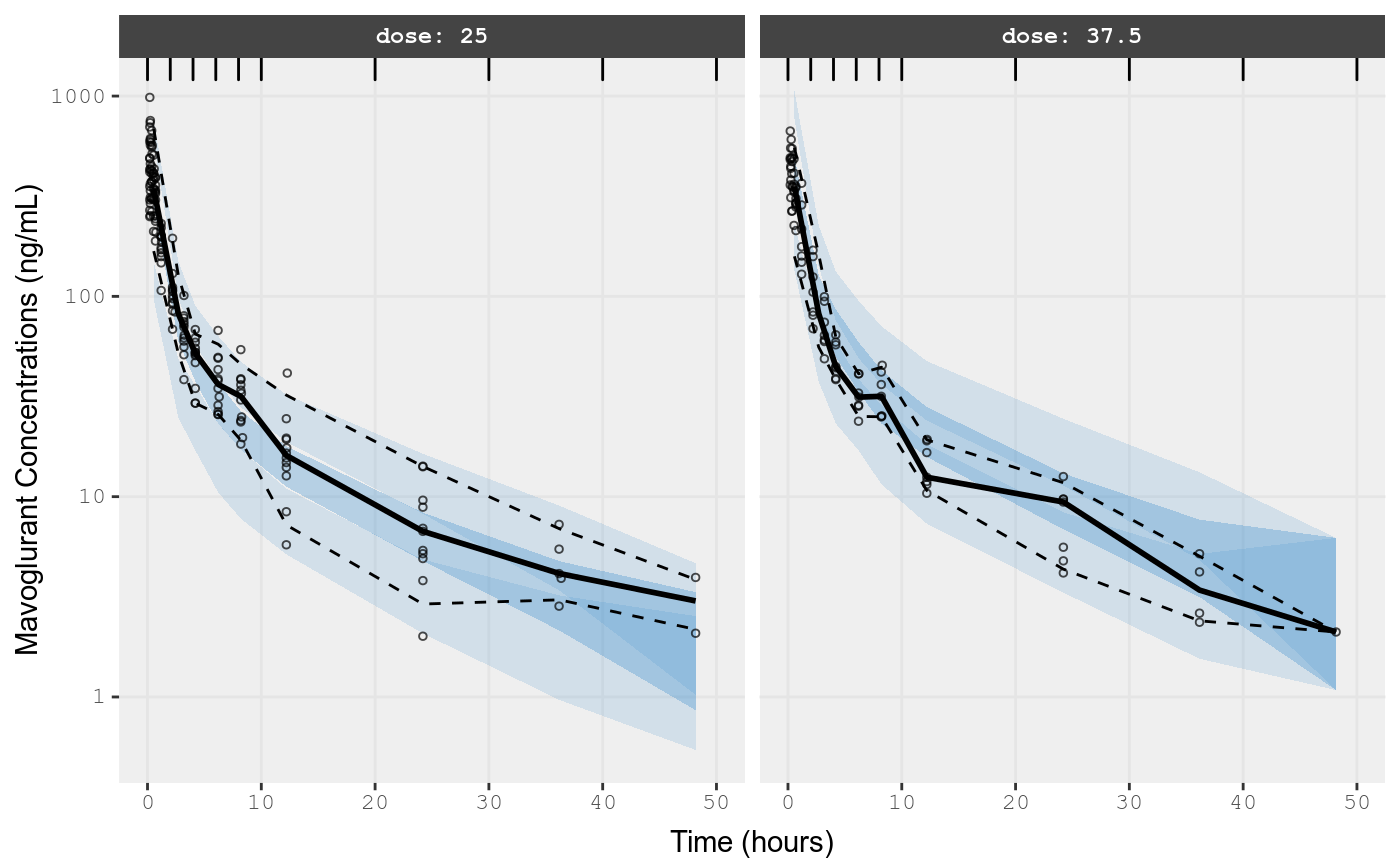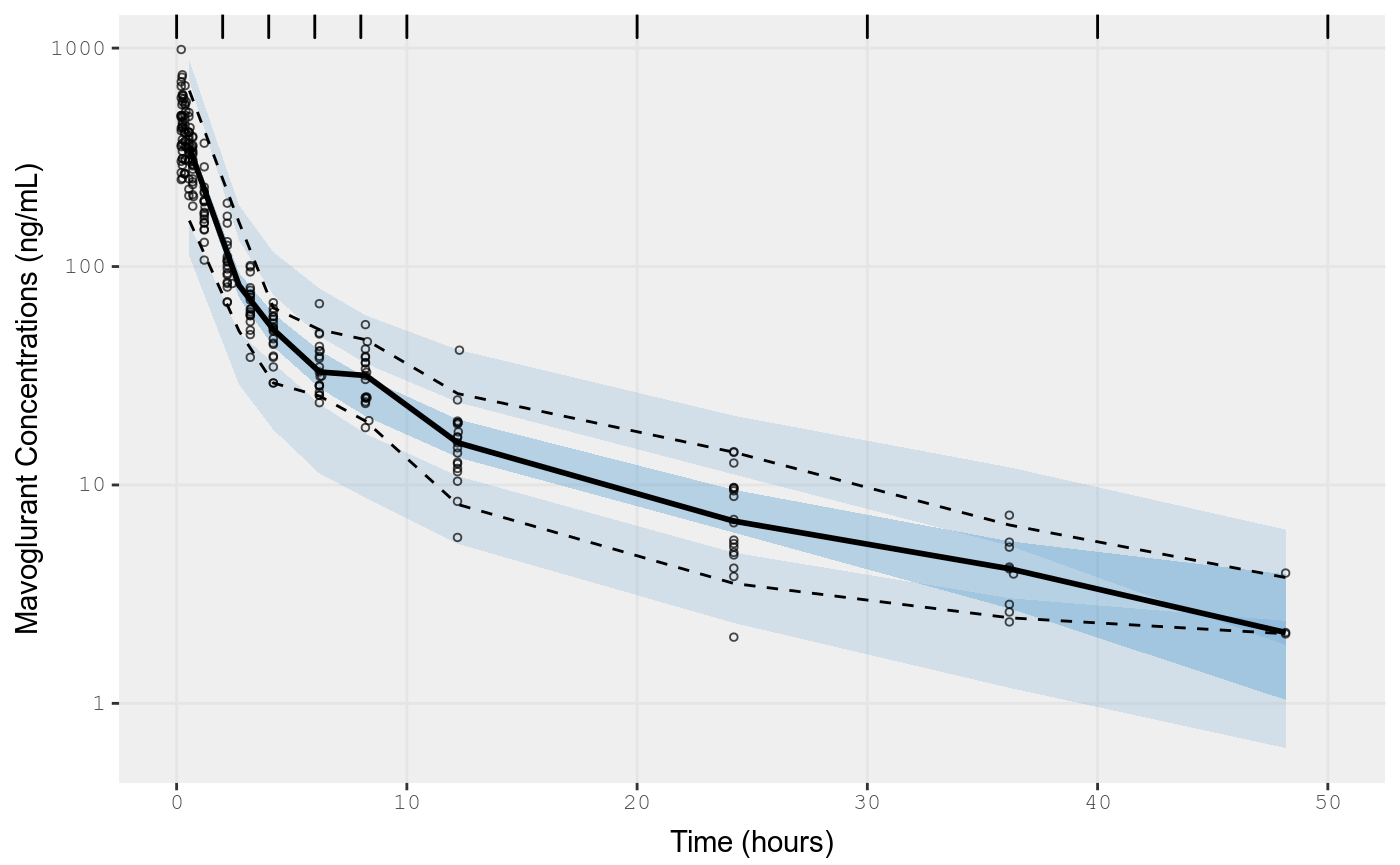## Lognoral

fit.lnorm.F <- fit.addProp.F %>%
model({C15 ~ lnorm(lnorm.err)}) %>%
nlmixr(est="focei"); ## In this model pipline we are changing the fit method to focei.
#> Loading model already run (/home/matt/R/x86_64-pc-linux-gnu-library/3.5/nlmixr.examples/nlmixr-focei-1bcc21b514be5224431362bc688180b2.rds)

gofs(fit.lnorm.F);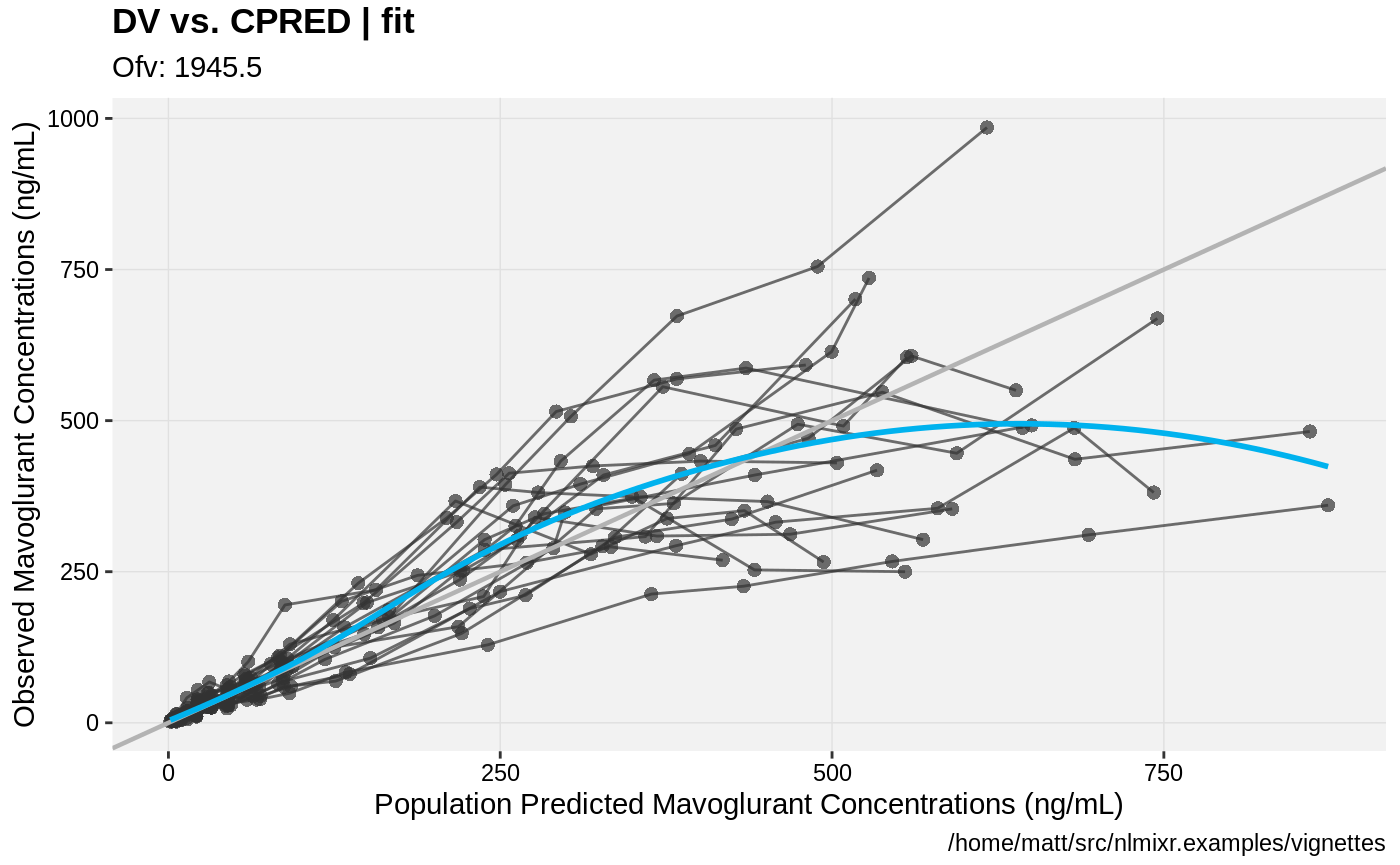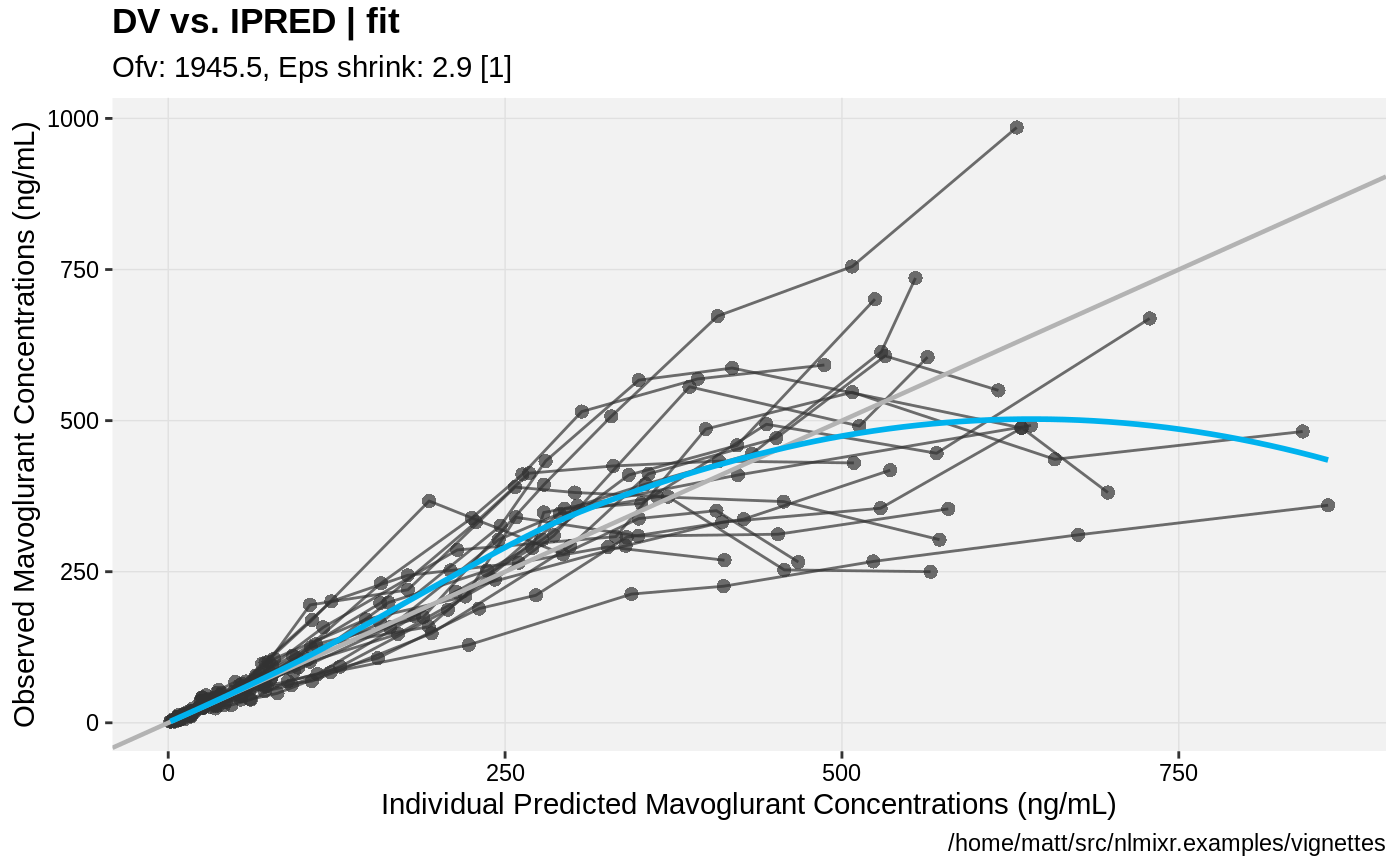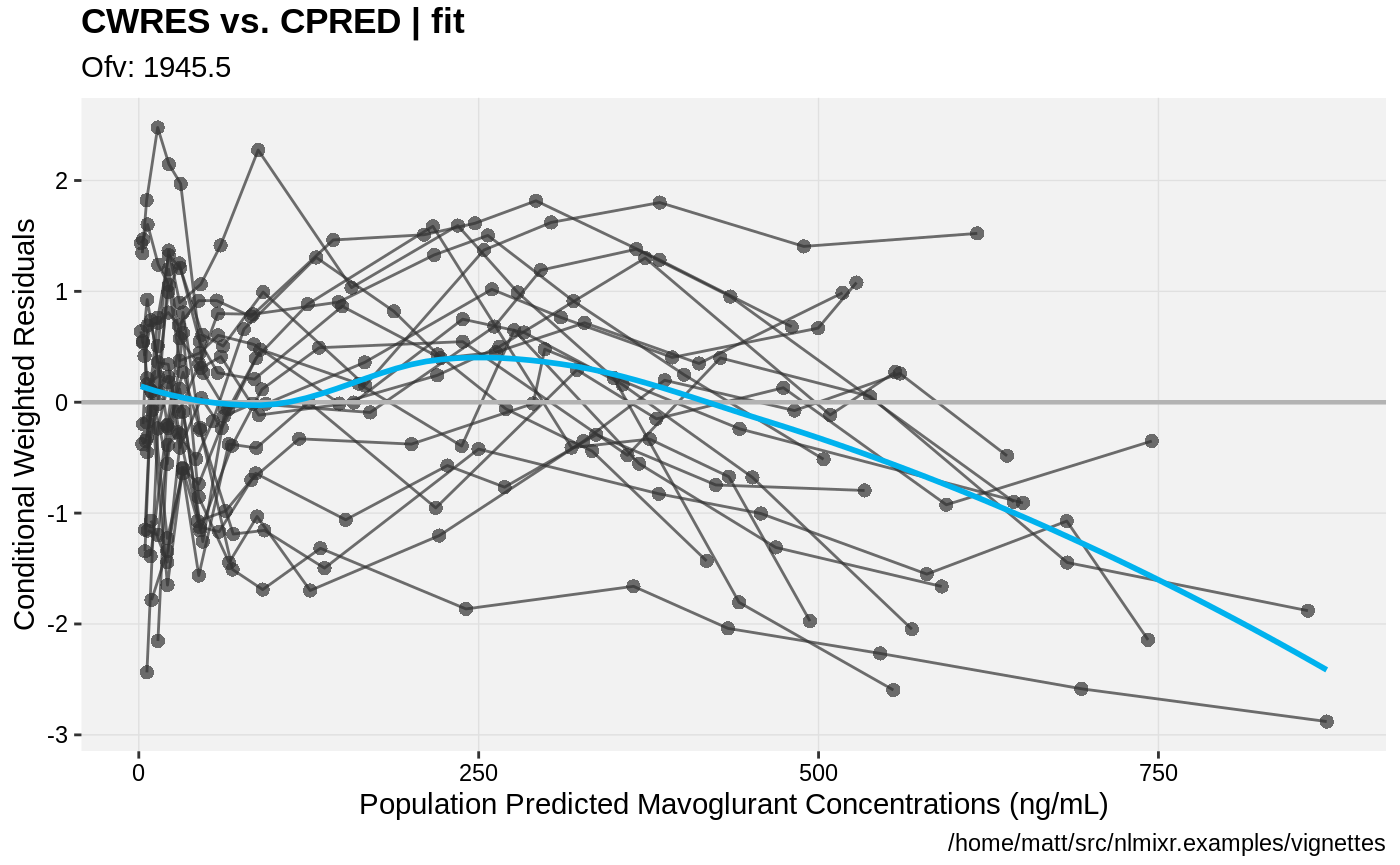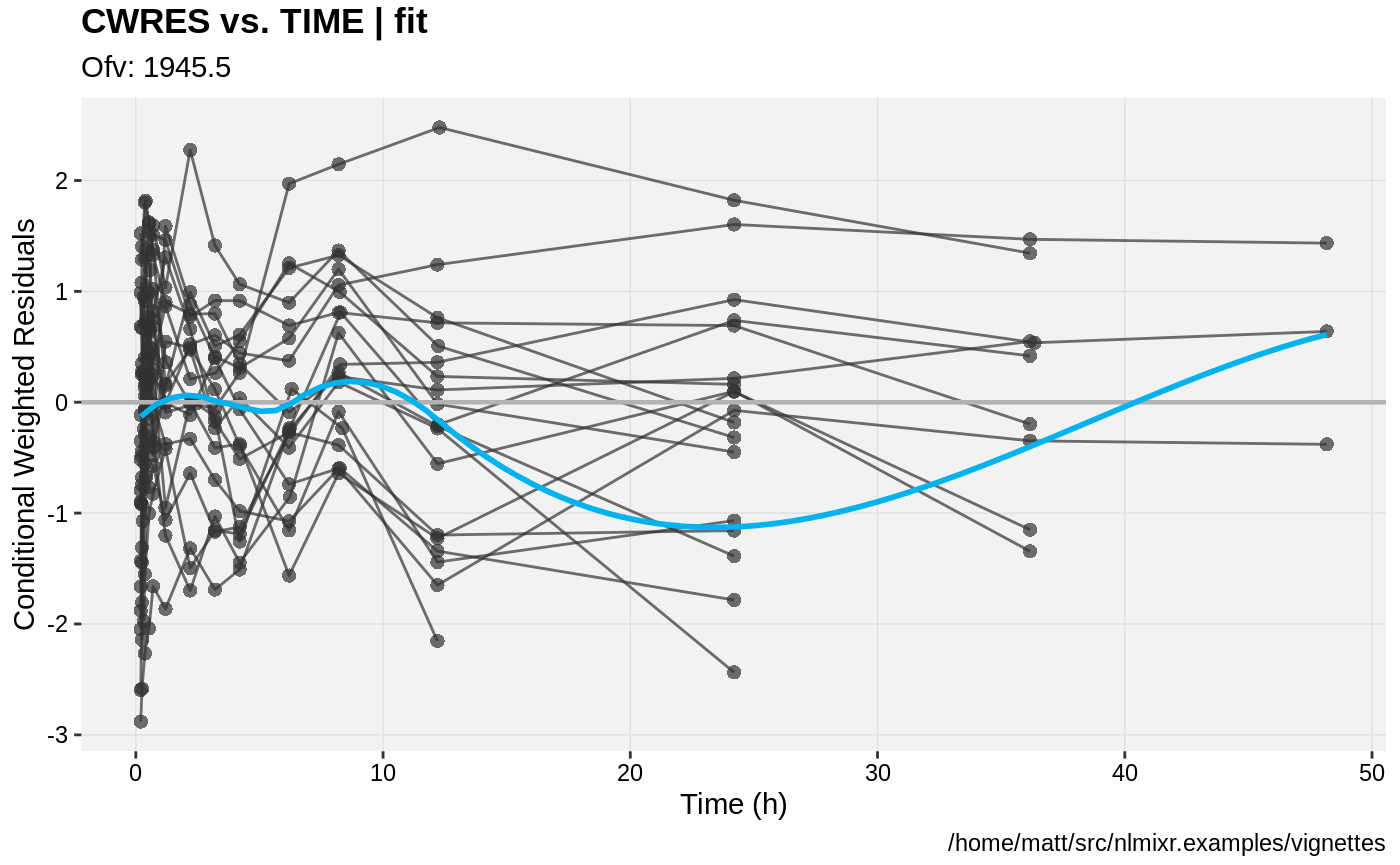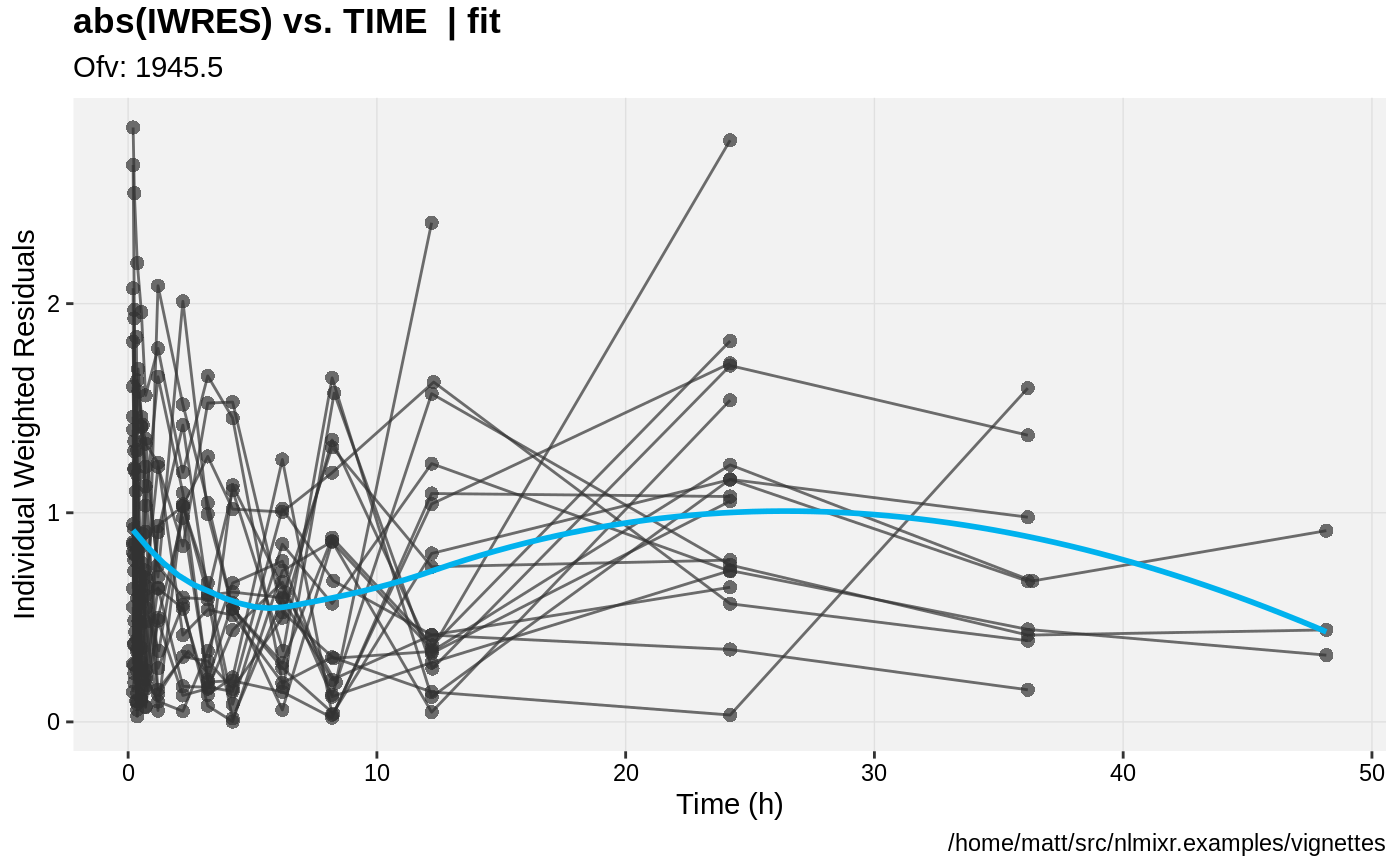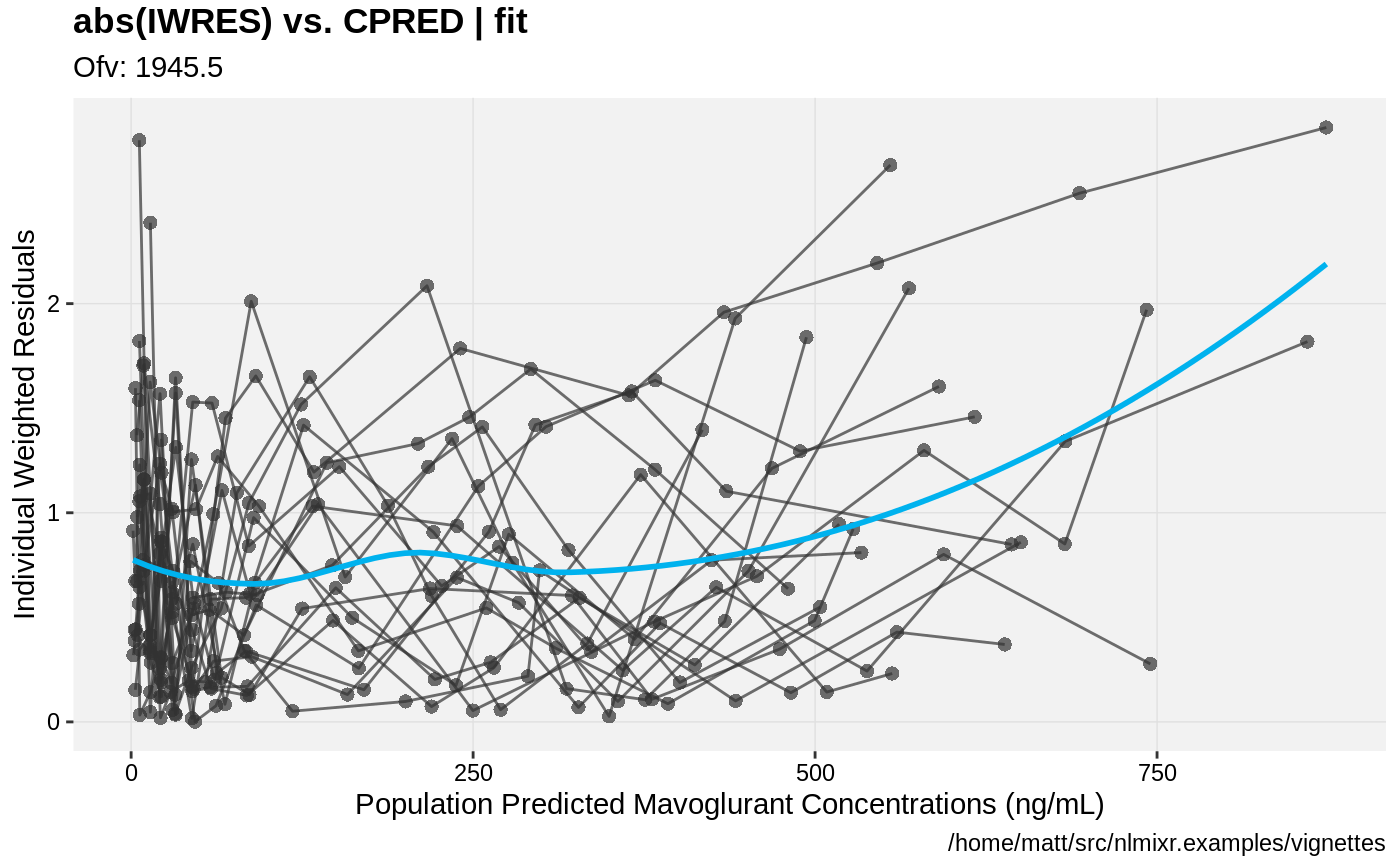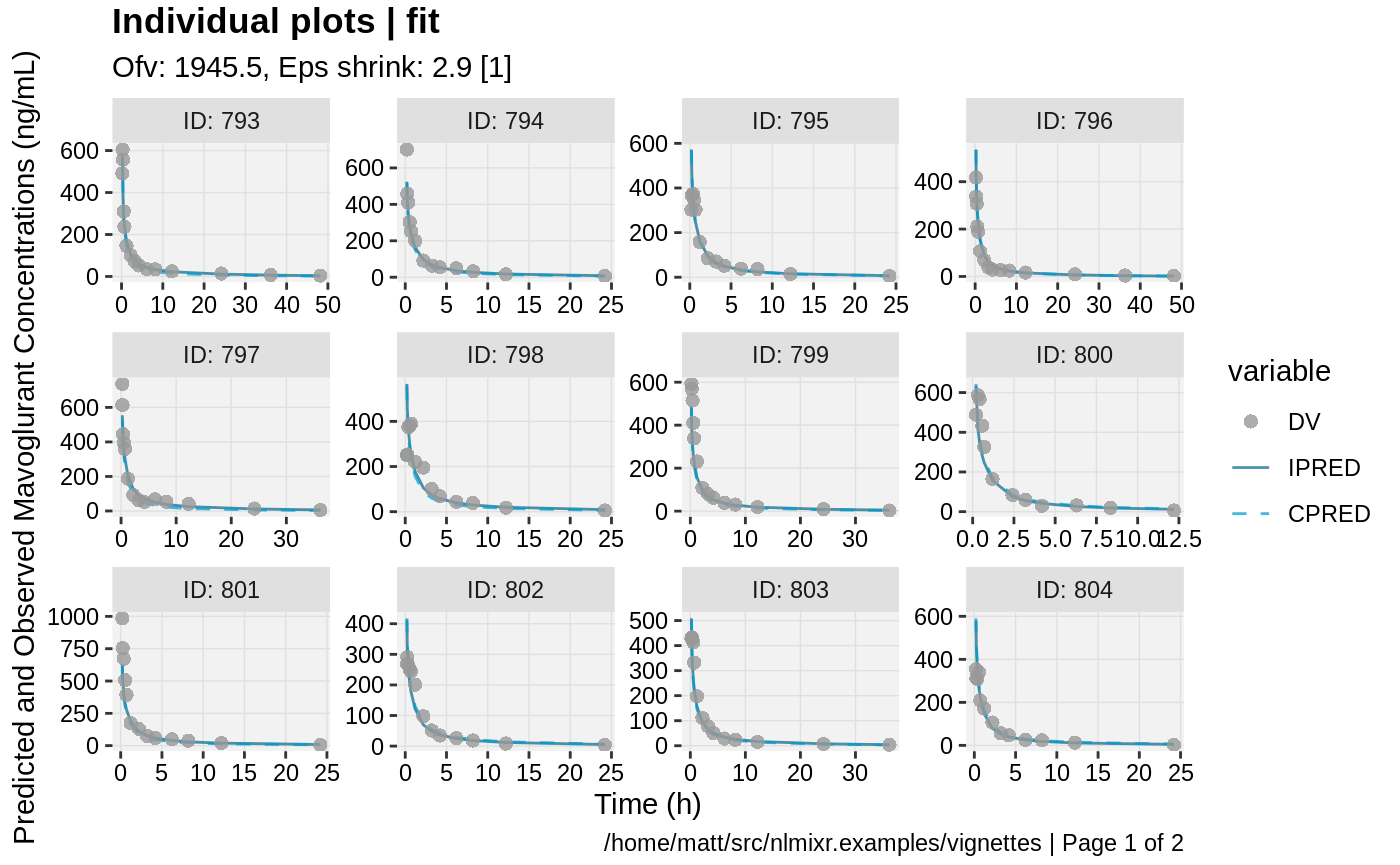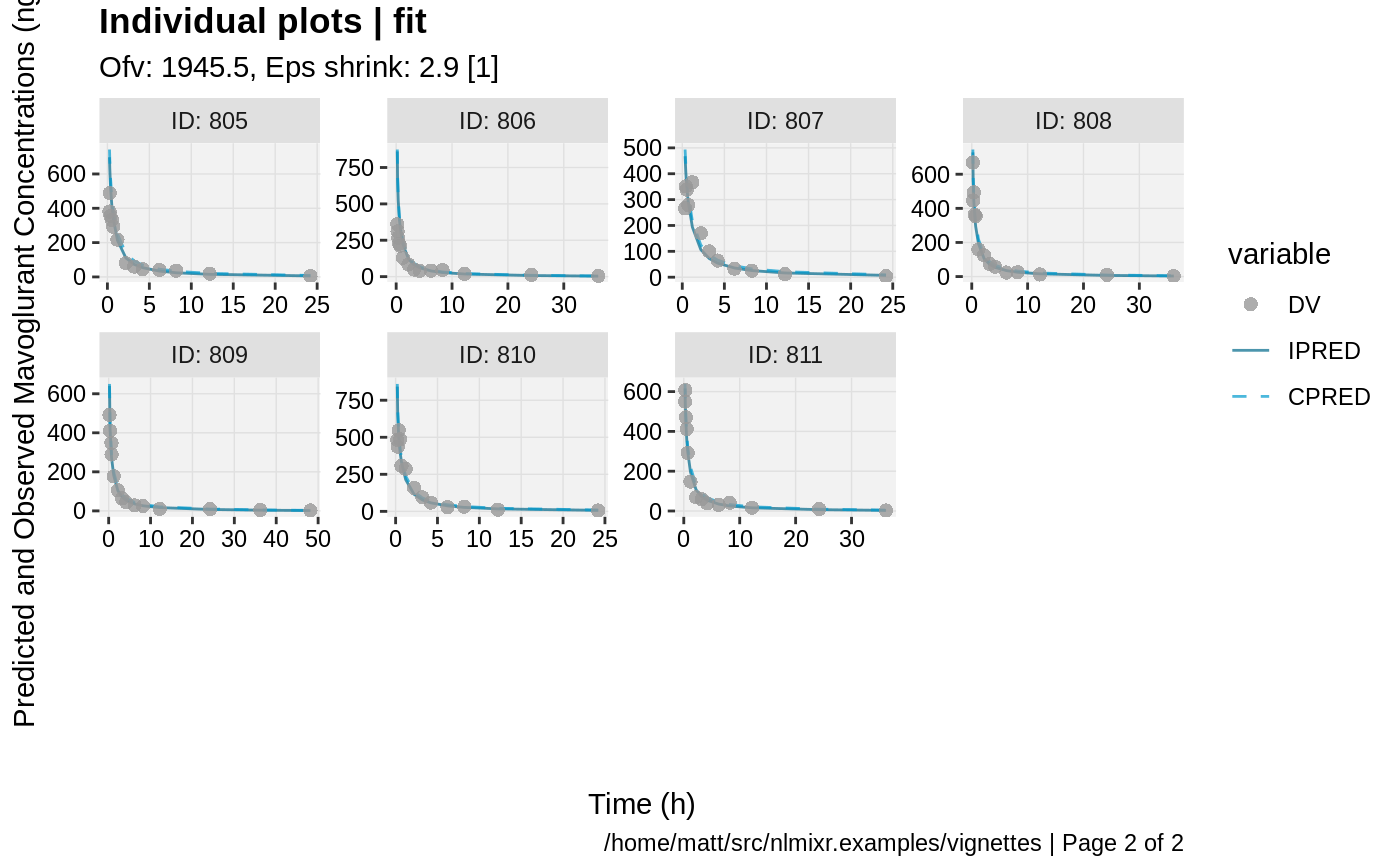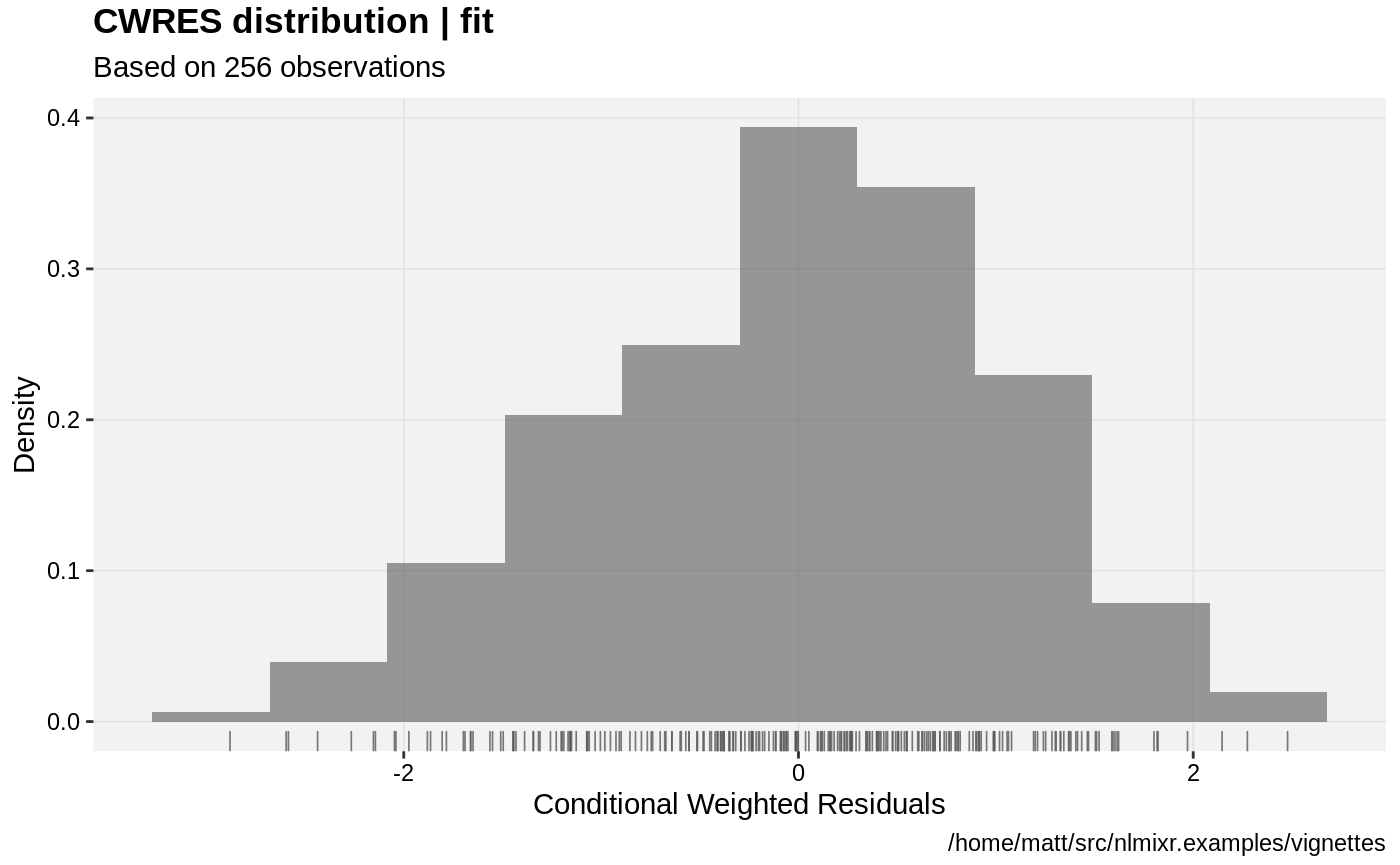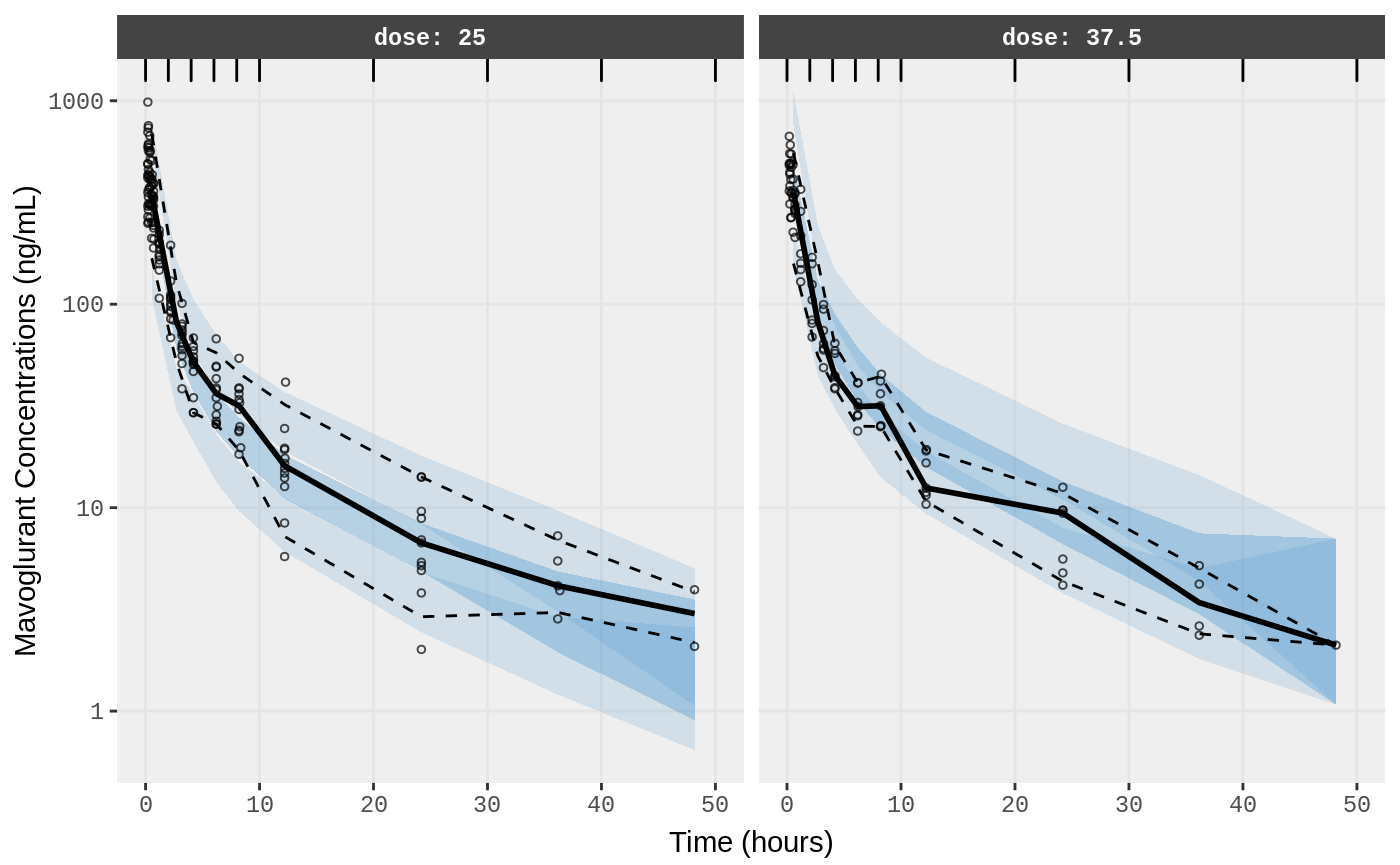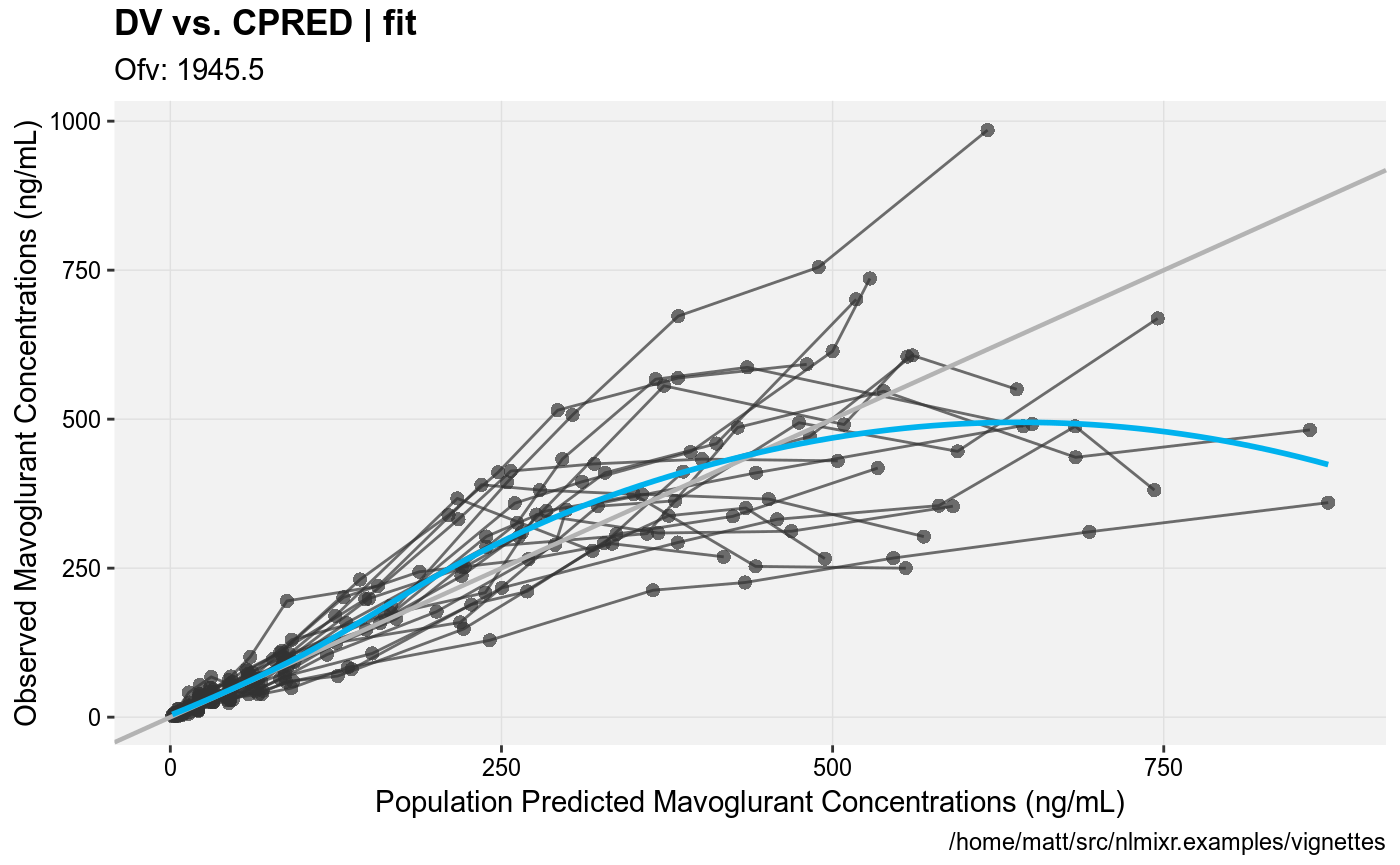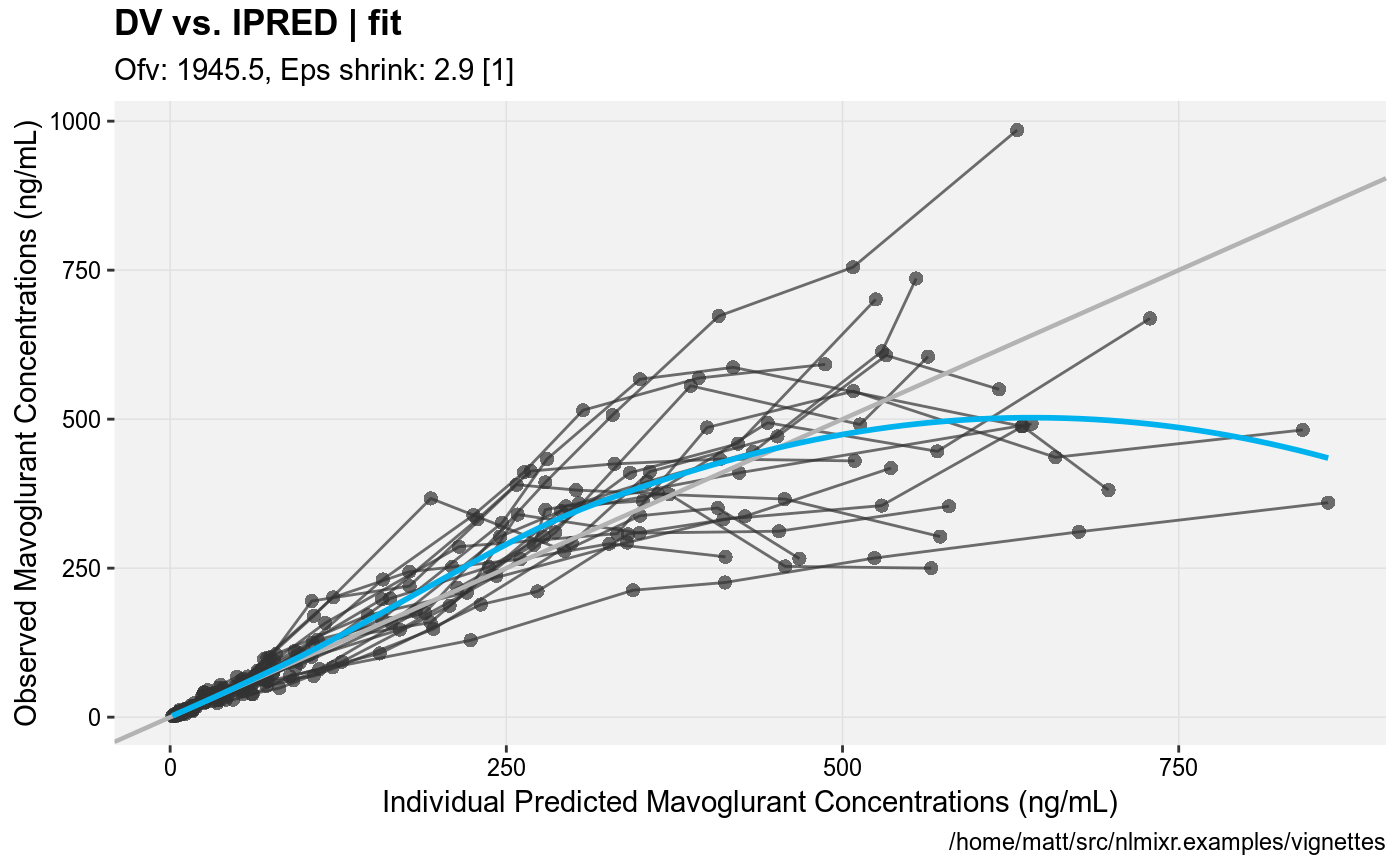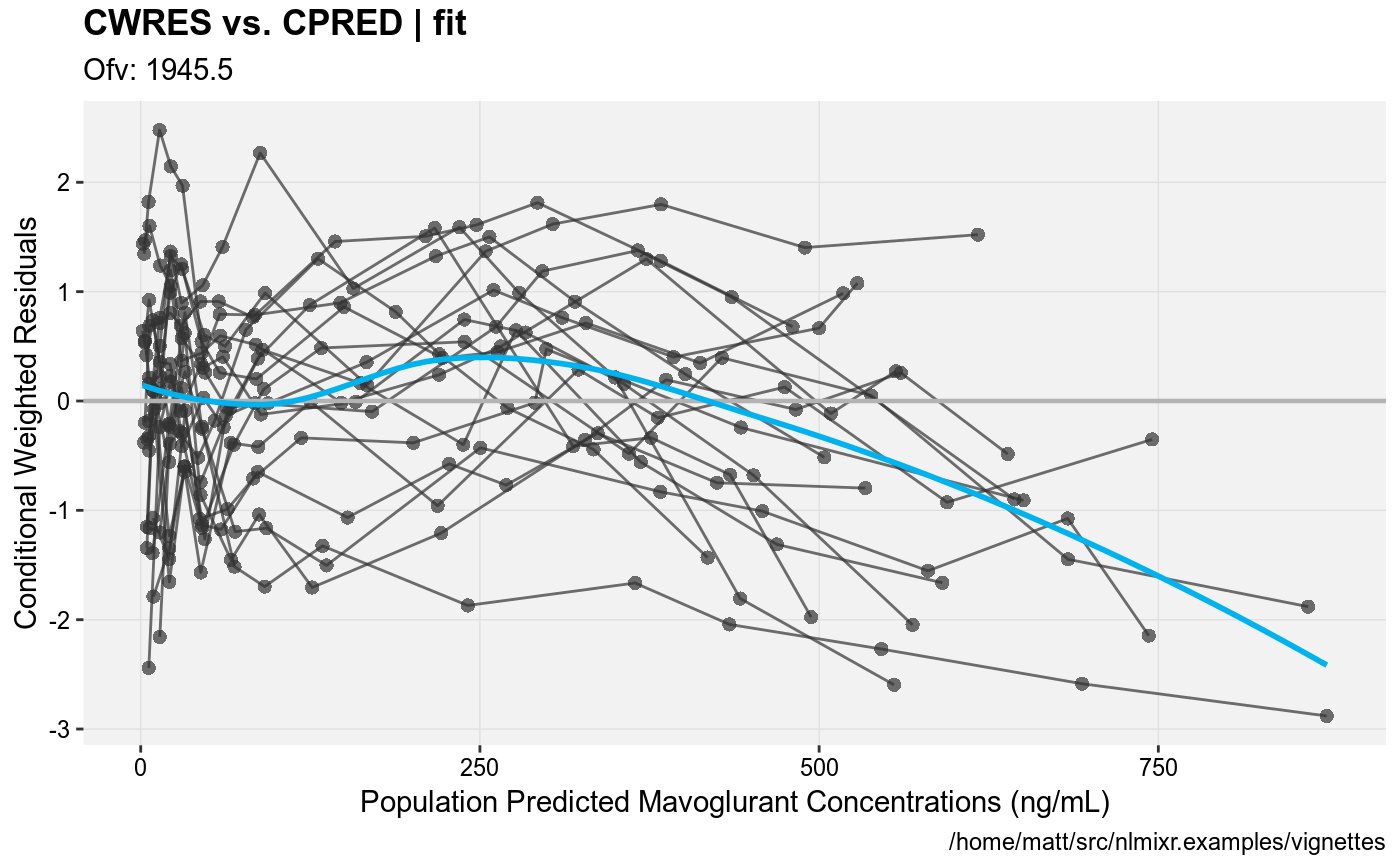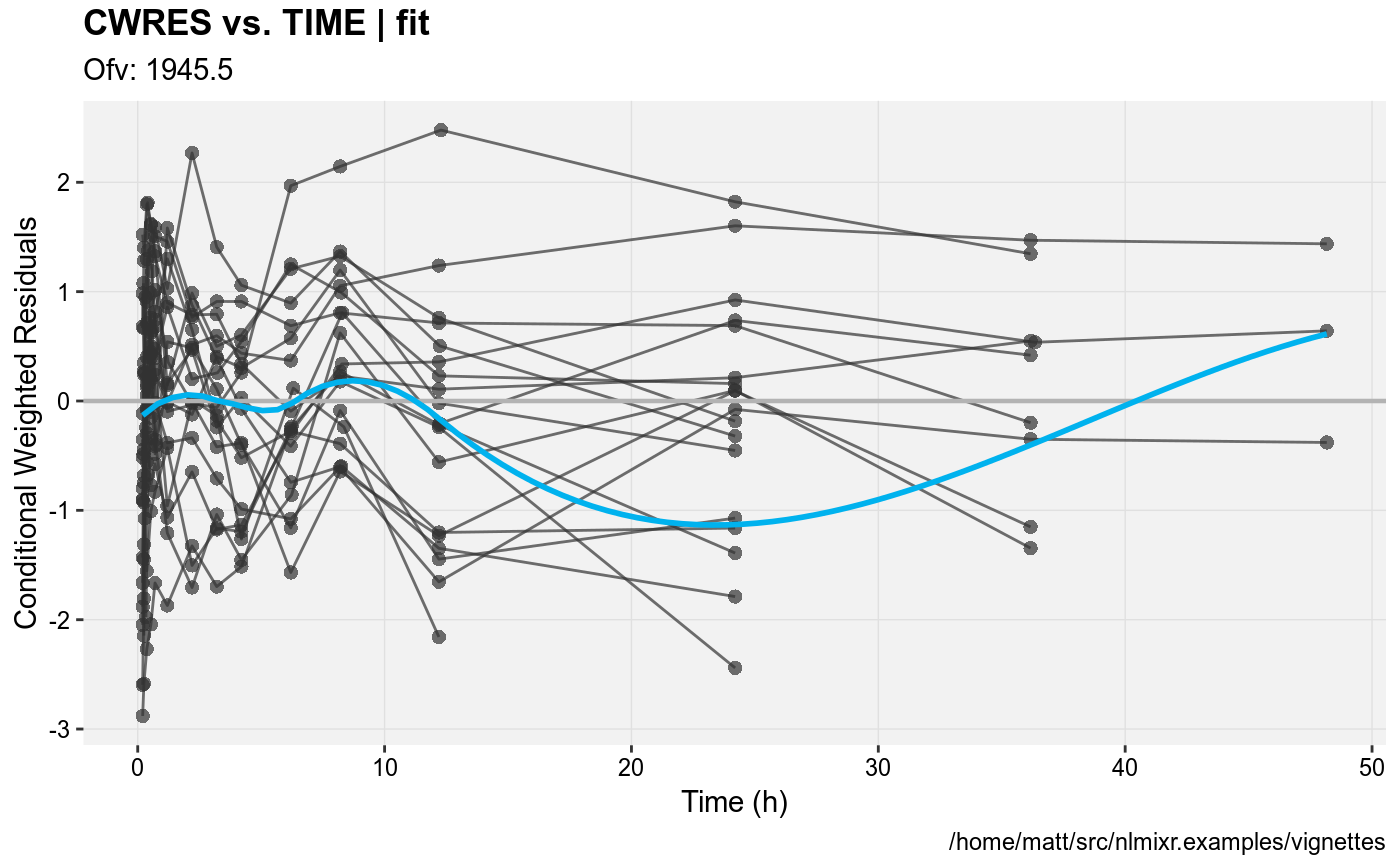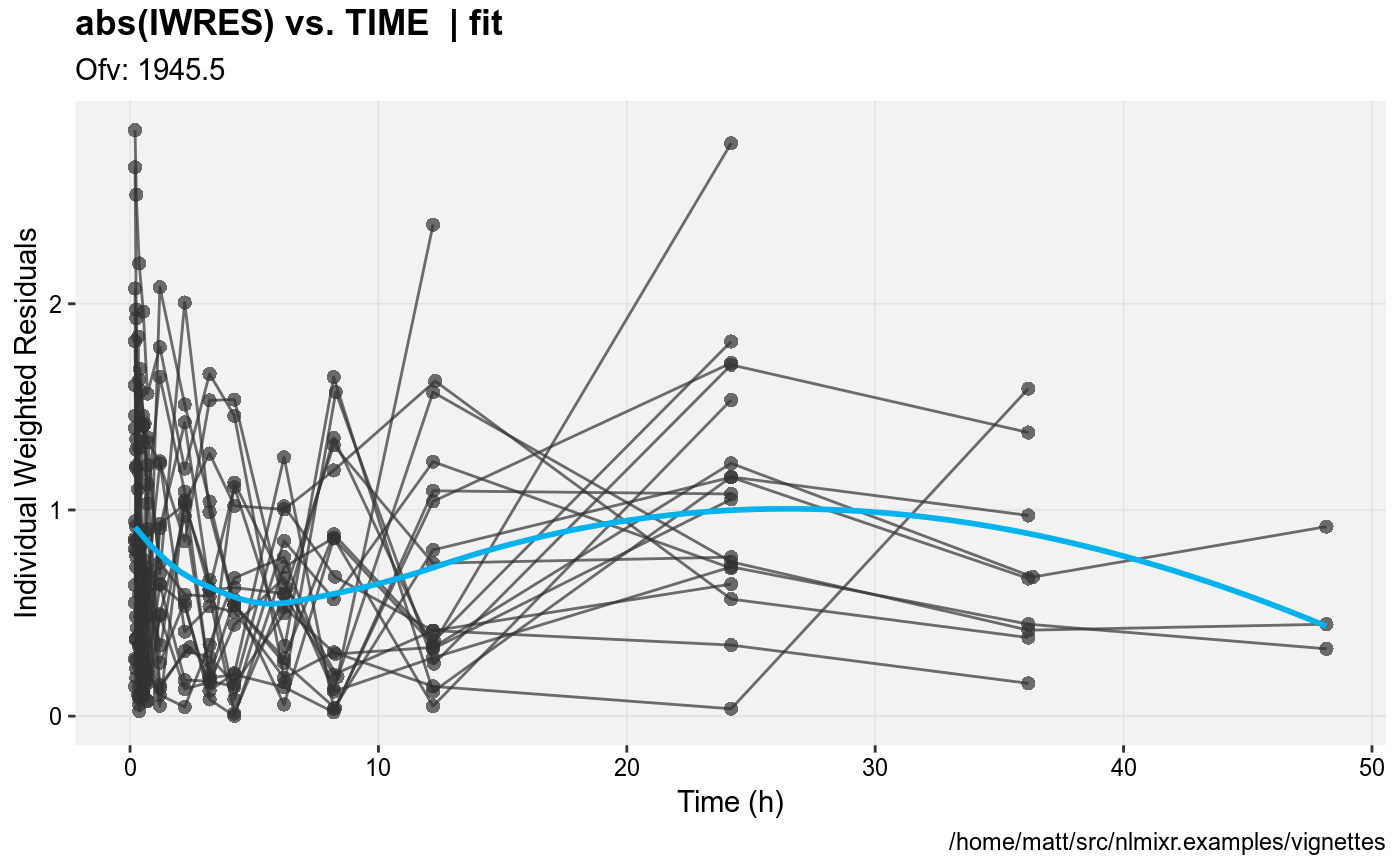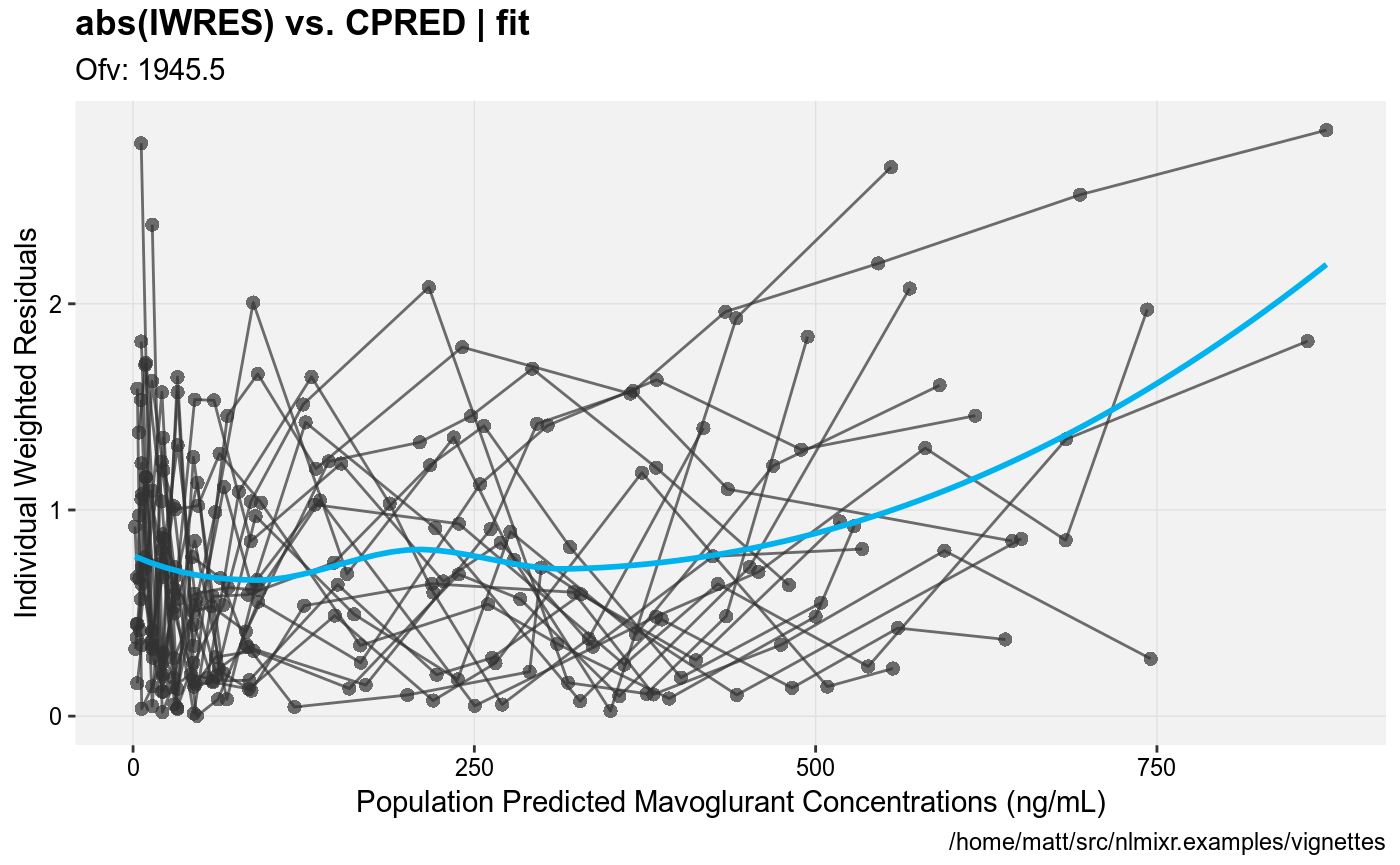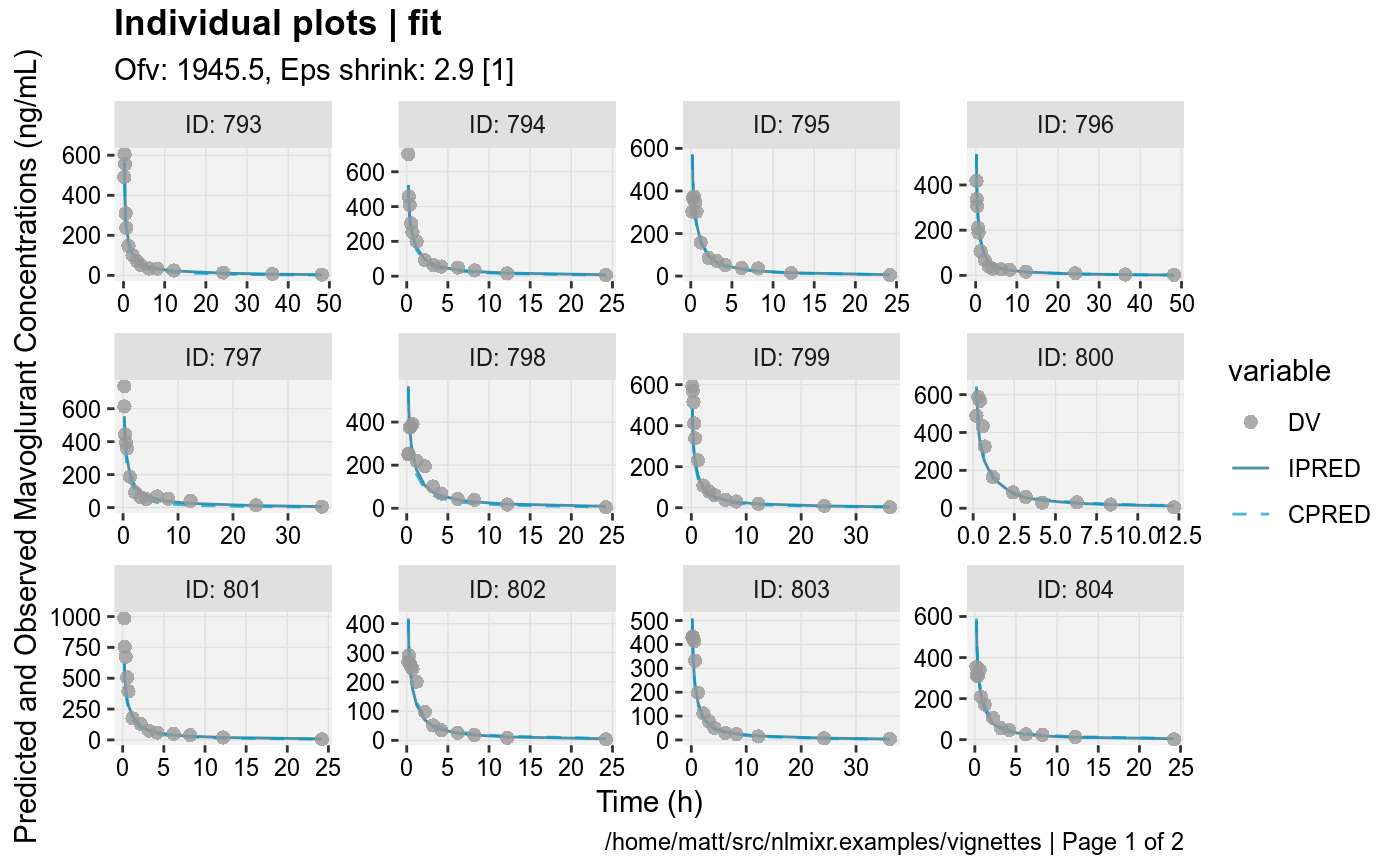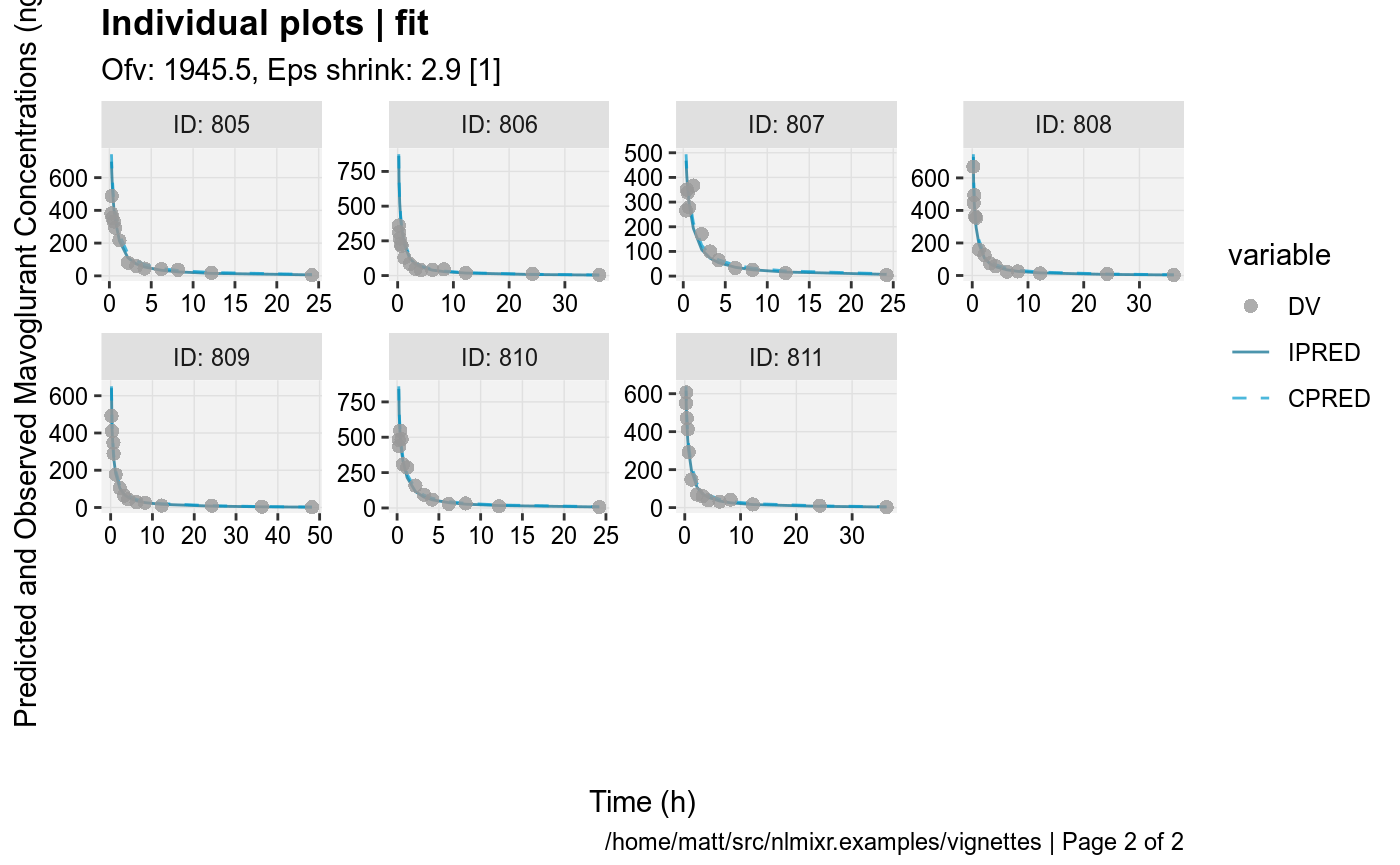#> Compiling VPC model...
#> done
#> done (4.42 sec)
#> Warning in data.frame(..., check.names = FALSE): row names were found from
#> a short variable and have been discarded
#> Compiling VPC model...
#> done
#> done (4.30 sec)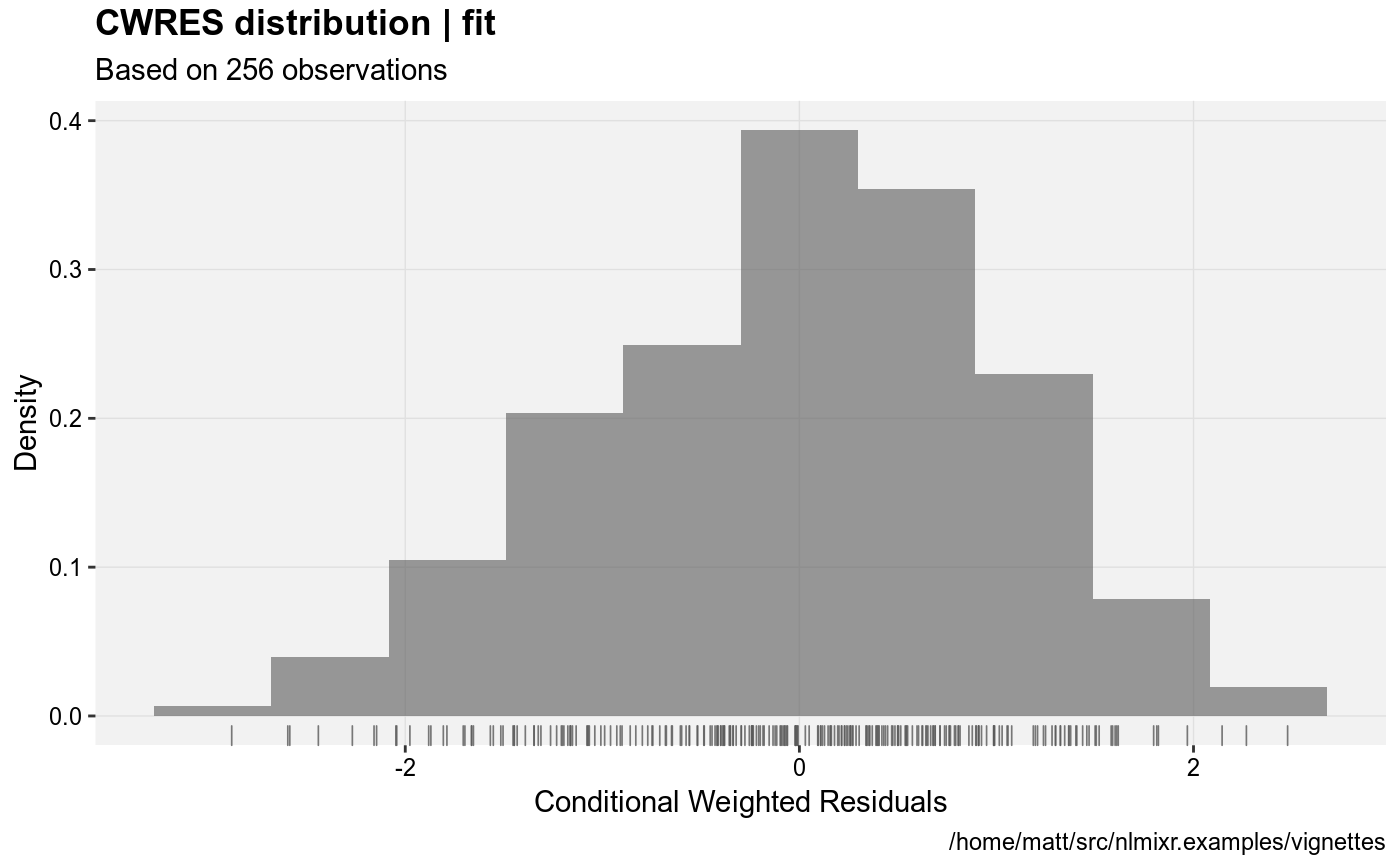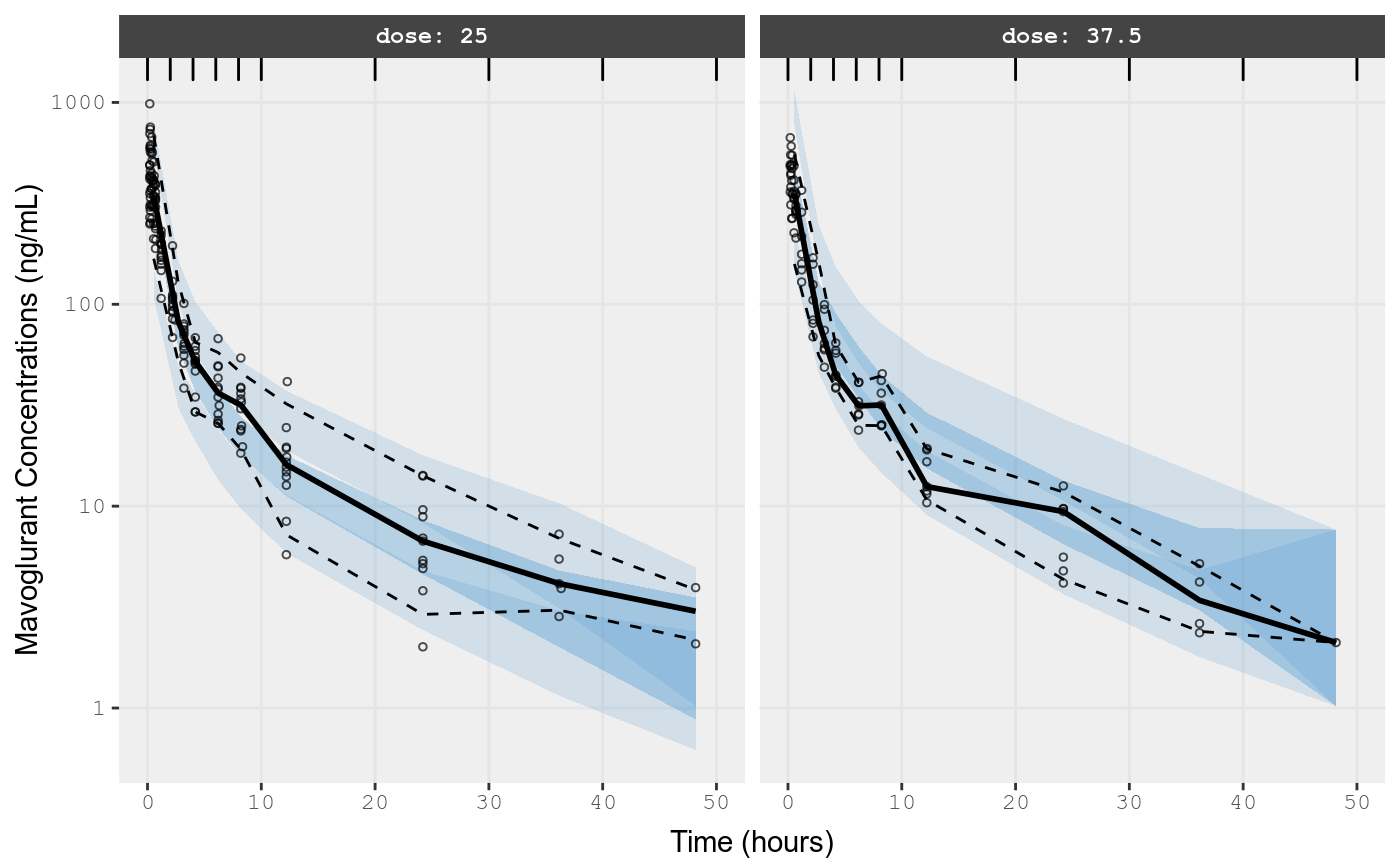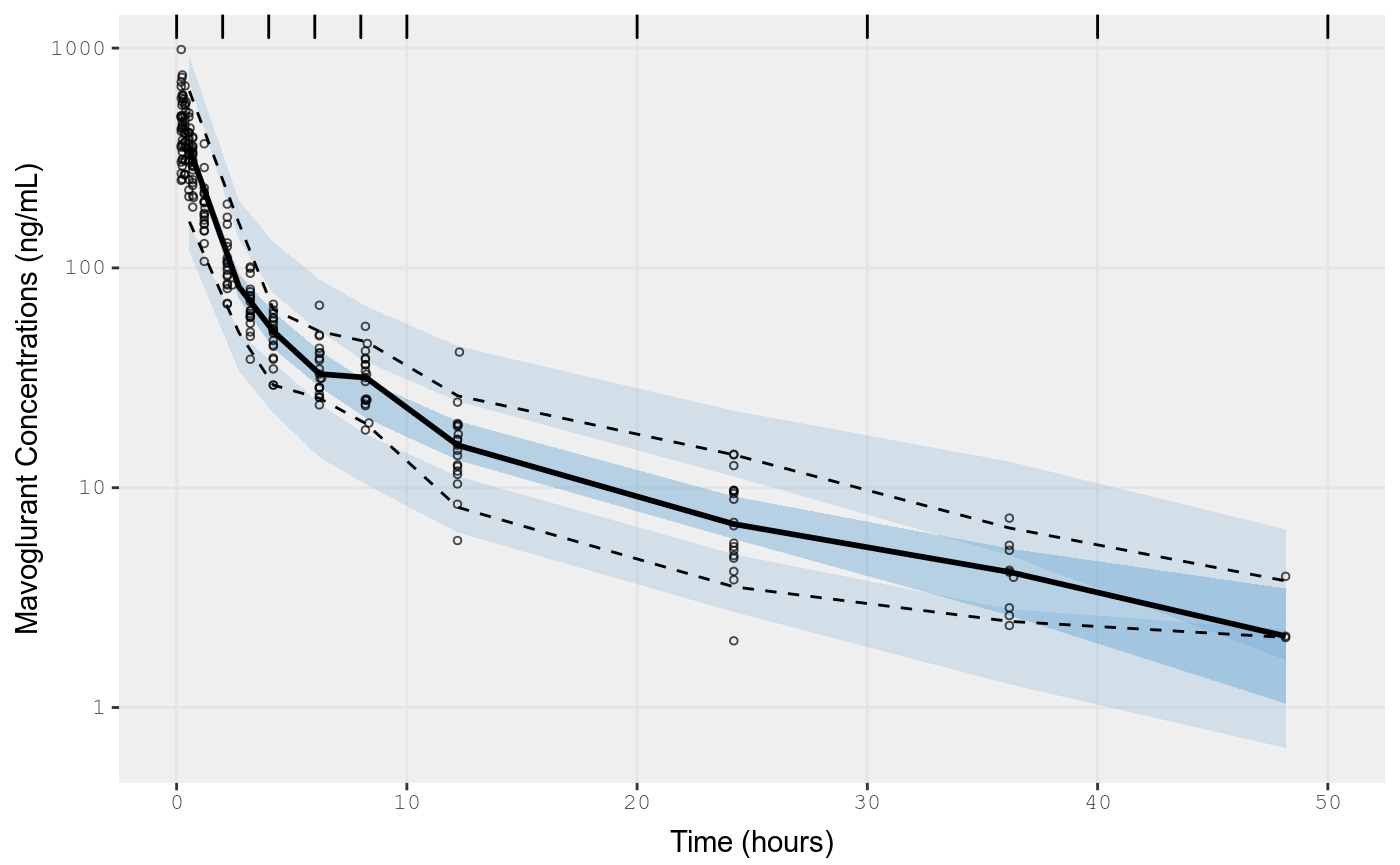# Traditional lognormal estimates are identical

datL  <- dat
datL$DV <- log(datL$DV);

pbpkL <- function(){
ini({
##theta=exp(c(1.1, .3, 2, 7.6, .003, .3))
lKbBR = 1.1
lKbMU = 0.3
lKbAD = 2
lCLint = 7.6
lKbBO = 0.03
lKbRB = 0.3
eta.LClint ~ 4
add.err <- 1
})
model({
KbBR = exp(lKbBR)
KbMU = exp(lKbMU)
KbAD = exp(lKbAD)
CLint= exp(lCLint + eta.LClint)
KbBO = exp(lKbBO)
KbRB = exp(lKbRB)

## Regional blood flows
CO  = (187.00*WT^0.81)*60/1000;         # Cardiac output (L/h) from White et al (1968)
QHT = 4.0 *CO/100;
QBR = 12.0*CO/100;
QMU = 17.0*CO/100;
QAD = 5.0 *CO/100;
QSK = 5.0 *CO/100;
QSP = 3.0 *CO/100;
QPA = 1.0 *CO/100;
QLI = 25.5*CO/100;
QST = 1.0 *CO/100;
QGU = 14.0*CO/100;
QHA = QLI - (QSP + QPA + QST + QGU); # Hepatic artery blood flow
QBO = 5.0 *CO/100;
QKI = 19.0*CO/100;
QRB = CO - (QHT + QBR + QMU + QAD + QSK + QLI + QBO + QKI);
QLU = QHT + QBR + QMU + QAD + QSK + QLI + QBO + QKI + QRB;

## Organs' volumes = organs' weights / organs' density
VLU = (0.76 *WT/100)/1.051;
VHT = (0.47 *WT/100)/1.030;
VBR = (2.00 *WT/100)/1.036;
VMU = (40.00*WT/100)/1.041;
VAD = (21.42*WT/100)/0.916;
VSK = (3.71 *WT/100)/1.116;
VSP = (0.26 *WT/100)/1.054;
VPA = (0.14 *WT/100)/1.045;
VLI = (2.57 *WT/100)/1.040;
VST = (0.21 *WT/100)/1.050;
VGU = (1.44 *WT/100)/1.043;
VBO = (14.29*WT/100)/1.990;
VKI = (0.44 *WT/100)/1.050;
VAB = (2.81 *WT/100)/1.040;
VVB = (5.62 *WT/100)/1.040;
VRB = (3.86 *WT/100)/1.040;

## Fixed parameters
BP = 0.61;      # Blood:plasma partition coefficient
fup = 0.028;    # Fraction unbound in plasma
fub = fup/BP;   # Fraction unbound in blood

KbLU = exp(0.8334);
KbHT = exp(1.1205);
KbSK = exp(-.5238);
KbSP = exp(0.3224);
KbPA = exp(0.3224);
KbLI = exp(1.7604);
KbST = exp(0.3224);
KbGU = exp(1.2026);
KbKI = exp(1.3171);

##-----------------------------------------
S15 = VVB*BP/1000;
C15 = Venous_Blood/S15
lnC15 = log(C15);

##-----------------------------------------
d/dt(Lungs) = QLU*(Venous_Blood/VVB - Lungs/KbLU/VLU);
d/dt(Heart) = QHT*(Arterial_Blood/VAB - Heart/KbHT/VHT);
d/dt(Brain) = QBR*(Arterial_Blood/VAB - Brain/KbBR/VBR);
d/dt(Muscles) = QMU*(Arterial_Blood/VAB - Muscles/KbMU/VMU);
d/dt(Adipose) = QAD*(Arterial_Blood/VAB - Adipose/KbAD/VAD);
d/dt(Skin) = QSK*(Arterial_Blood/VAB - Skin/KbSK/VSK);
d/dt(Spleen) = QSP*(Arterial_Blood/VAB - Spleen/KbSP/VSP);
d/dt(Pancreas) = QPA*(Arterial_Blood/VAB - Pancreas/KbPA/VPA);
d/dt(Liver) = QHA*Arterial_Blood/VAB + QSP*Spleen/KbSP/VSP + QPA*Pancreas/KbPA/VPA + QST*Stomach/KbST/VST + QGU*Gut/KbGU/VGU - CLint*fub*Liver/KbLI/VLI - QLI*Liver/KbLI/VLI;
d/dt(Stomach) = QST*(Arterial_Blood/VAB - Stomach/KbST/VST);
d/dt(Gut) = QGU*(Arterial_Blood/VAB - Gut/KbGU/VGU);
d/dt(Bones) = QBO*(Arterial_Blood/VAB - Bones/KbBO/VBO);
d/dt(Kidneys) = QKI*(Arterial_Blood/VAB - Kidneys/KbKI/VKI);
d/dt(Arterial_Blood) = QLU*(Lungs/KbLU/VLU - Arterial_Blood/VAB);
d/dt(Venous_Blood) = QHT*Heart/KbHT/VHT + QBR*Brain/KbBR/VBR +
QMU*Muscles/KbMU/VMU + QAD*Adipose/KbAD/VAD +
QSK*Skin/KbSK/VSK + QLI*Liver/KbLI/VLI + QBO*Bones/KbBO/VBO +
QKI*Kidneys/KbKI/VKI + QRB*Rest_of_Body/KbRB/VRB - QLU*Venous_Blood/VVB;
d/dt(Rest_of_Body) = QRB*(Arterial_Blood/VAB - Rest_of_Body/KbRB/VRB);

lnC15 ~ add(add.err)
})
}

fit.lnorm.trans <- pbpkL %>%
nlmixr(datL,est="saem")
#> Loading model already run (/home/matt/R/x86_64-pc-linux-gnu-library/3.5/nlmixr.examples/nlmixr-datL-saem-69231b3c7f750b0de4d4005083cbfeb7.rds)

NOTE: the estimates are the same but the AIC is different since it is calculated on the log scale.